PDFs are great for compatibility and consistency. They work on nearly any device and always maintain the same visual look. However, if you’re creating new content for the web, you should consider using web pages over PDFs.

Below, we’ll explore:

PDFs show in Google search results with a PDF tag.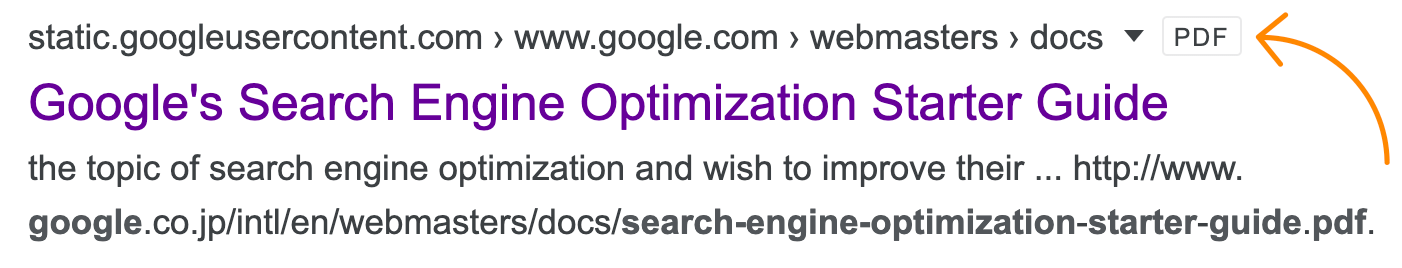Google SEO starter guide PDF in search results.

PDFs are converted to and indexed as HTML. For PDFs where there are images of text, Google uses Optical Character Recognition (OCR) technology to convert the image of text into text. Images in PDFs are also indexed in image search results.

Google chooses pages over PDFs if they’re duplicate. If you have pages and PDFs with the same content, Google tends to prefer the page version of the content as the lead version of the duplicate cluster. This means that signals will be consolidated to the page version and that will be the version that shows in search results.

## Why PDFs aren’t great for SEO

Even though Google indexes and occasionally ranks PDFs, the format has a few disadvantages over web pages:

1. Not mobile-friendly. PDFs are made to have a consistent appearance across devices. That means there is no such thing as a mobile-friendly PDF.
2. Lack of navigation. Most PDFs do not include navigational elements, making it more difficult for people to explore other content.
3. Lack of some SEO attributes. PDF files have equivalent versions of many SEO elements, but there are also many elements missing like individual link attributes like nofollow, UGC, and sponsored.
4. May not be crawled often. Because PDFs rarely change, they tend to be crawled less often than pages that are updated more frequently.
5. Tracking is more difficult. Most common trackers run JavaScript on a web page and don’t work in PDF files.

That said, I’m well aware that there are some situations where there’s no way around using a PDF for your content. If that’s the case for you, keep reading to learn how to optimize your PDFs for search.

Most on-page SEO elements that you’re used to seeing in HTML have an equivalent version in PDFs and are used in the same way you’re used to. Many are also there for accessibility reasons. So let’s discuss a few ways to optimize PDFs for SEO:

### 1. Write good content

Google’s company mission is to organize the world’s information. Even if it’s not a web page, good content is good content. I’ve seen lots of great content in PDFs like technical documentation, whitepapers, etc. Some of the best information on the web is buried in PDFs.

### 2. Add an optimized title

Just like web pages have title tags, PDFs have titles. Note that many search engines use the title to describe the document in their search results. If a PDF does not have a title, the filename appears in the SERP instead.

Here’s how to edit a PDFs title in Adobe Acrobat Pro:

1. Click File > Properties
2. Edit the Title field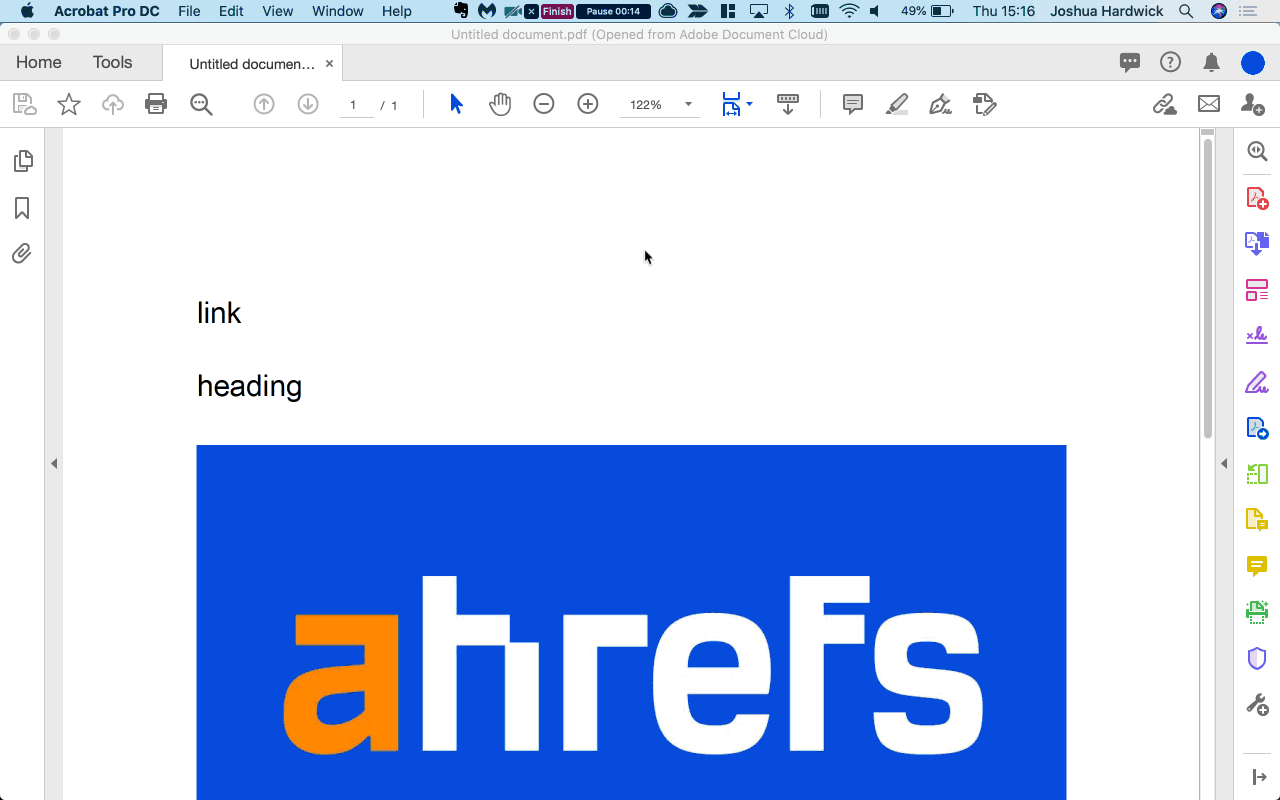### 3. Add an optimized description

As with meta descriptions for web pages, this isn’t a ranking factor but gives you a shot at controlling the text that appears in search results.

1. Click File > Properties
2. Click Additional Metadata
3. Edit Description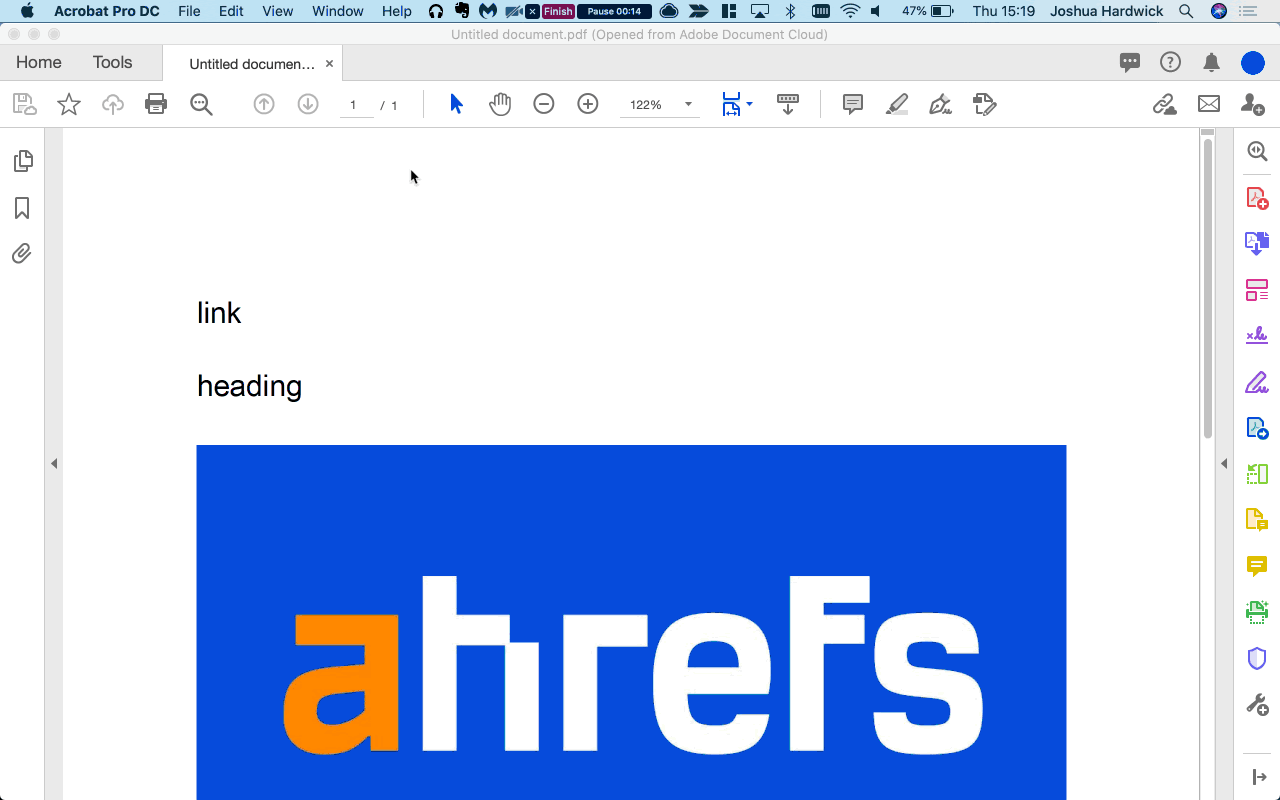### 4. Use a relevant file name

The filename of the PDF will be part of the URL. This will impact the URL shown in the search results and is a small ranking factor.

1. Click File > Save As
2. Edit File Name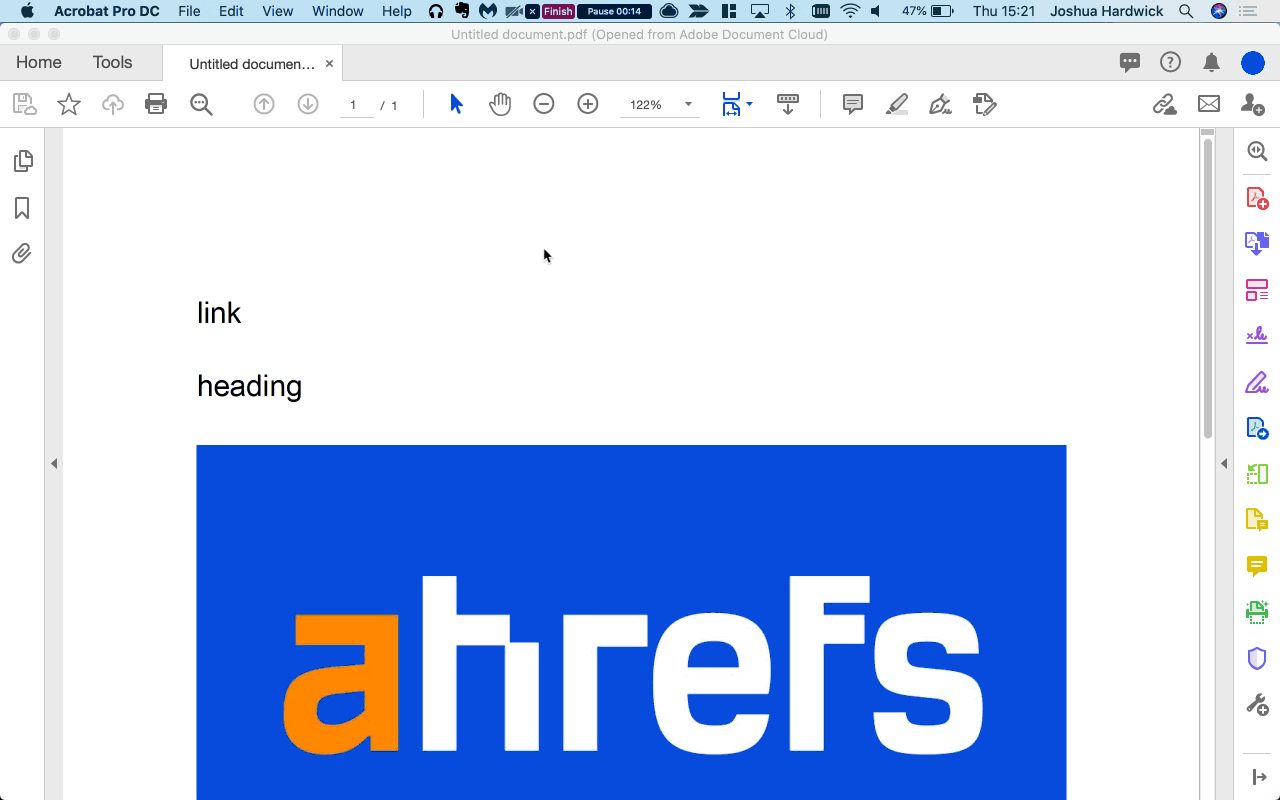### 5. Include image alt attributes

To help search engines understand the content of your images, you can add alt text to the images in your PDF.

1. Click the Tags icon in the left sidebar
2. Find the image you want to add alt text for in the document hierarchy
3. Right-click on the image
4. Click Properties
5. Add relevant alternate text to the box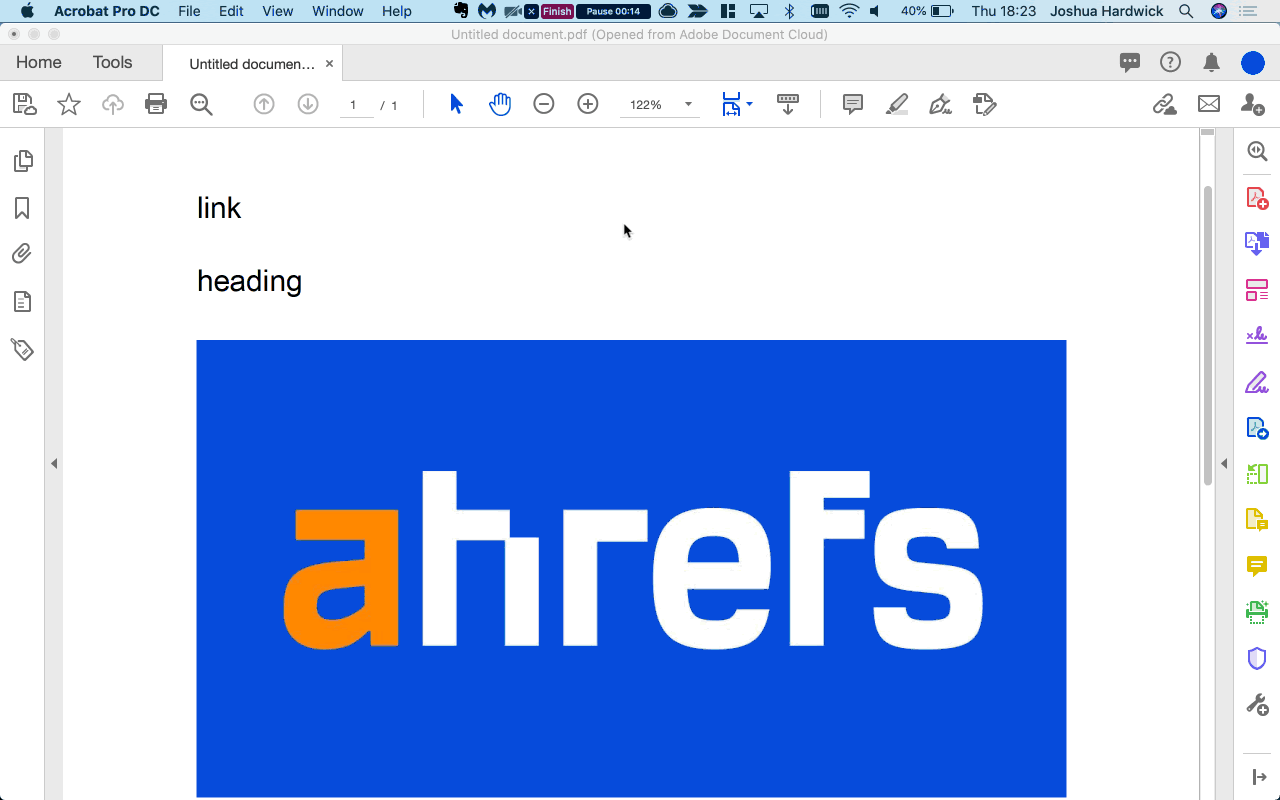### 6. Use headings

Just like your heading tags (H1-H6) in web pages, you can specify that certain text in PDFs are headings.

1. Click the Tags icon in the left sidebar
2. Find the text you want to edit in the document hierarchy
3. Right-click on the tag
4. Click Properties
5. Select the relevant heading level from the dropdown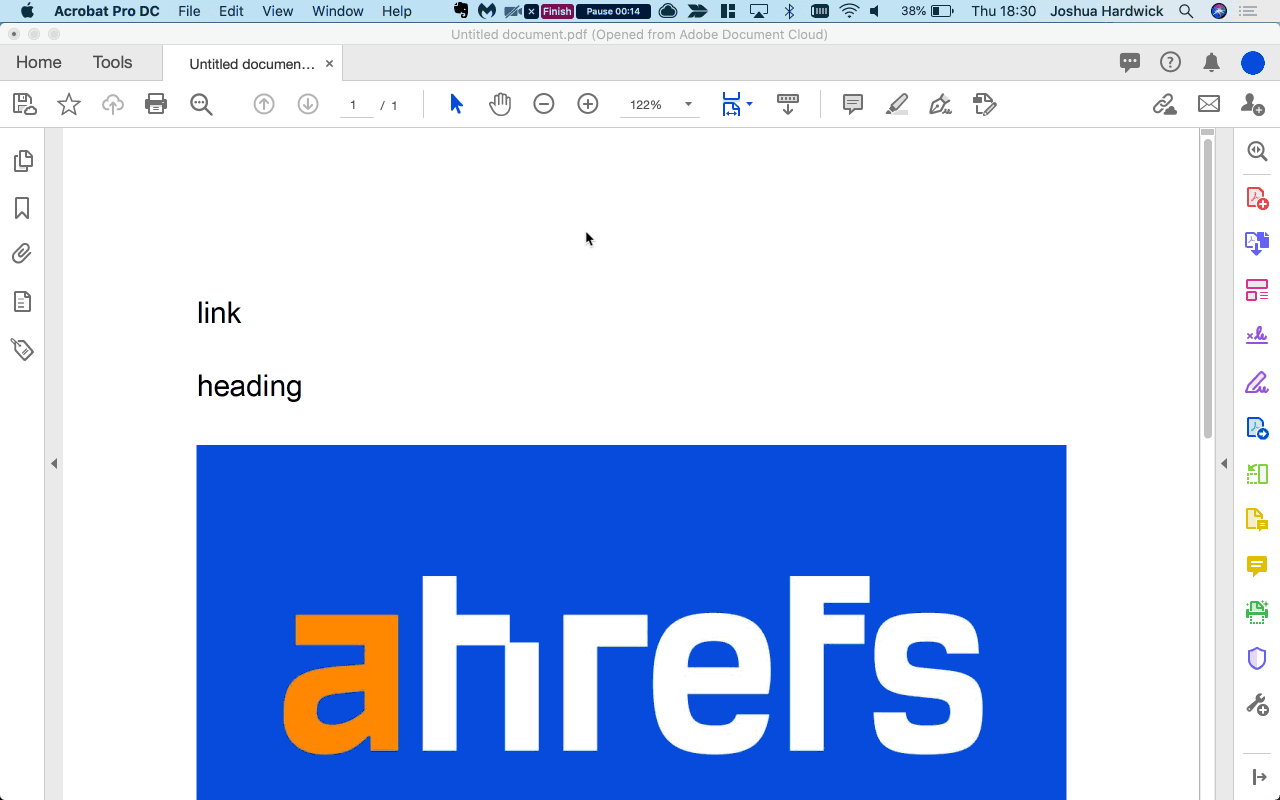Just like any page, internal and external links also impact rankings. Links pass PageRank and their anchor text adds context. By including links to your PDF and links from your PDF to other pages, you are helping PageRank flow through your site rather than creating a dead end. Some PDFs get a lot of links. Larry Page once said “It turns out, people who win the Nobel Prize have citations from 10,000 different papers”

Check out this GDPR document. It has 77K links from 823 referring domains to it but does not link out at all. This is a missed opportunity and adding some internal links from this PDF to other pages on the site might help those pages rank better.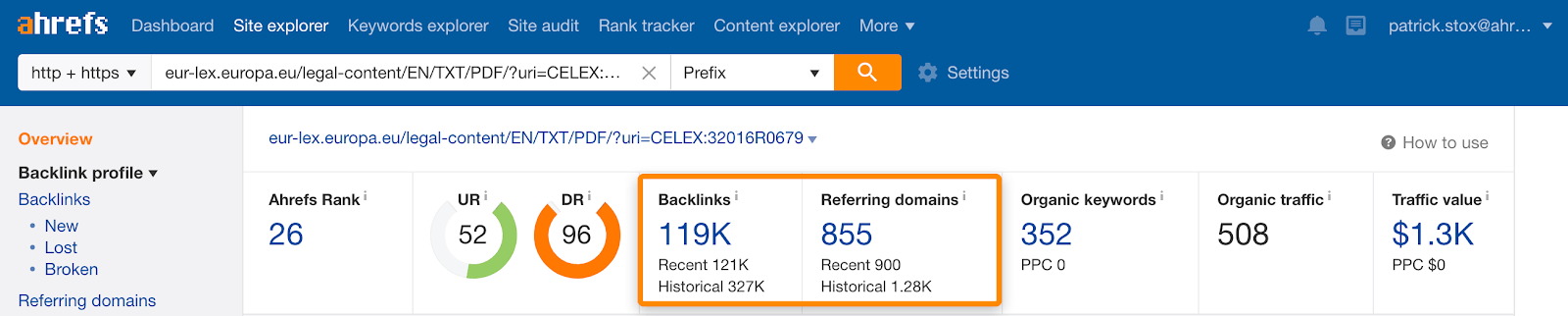Links to GDPR PDF.

This example from Google is better. Their SEO Starter Guide PDF has 3.37K links from 754 referring domains and they do a good job of passing that value to other pages by linking out from the PDF.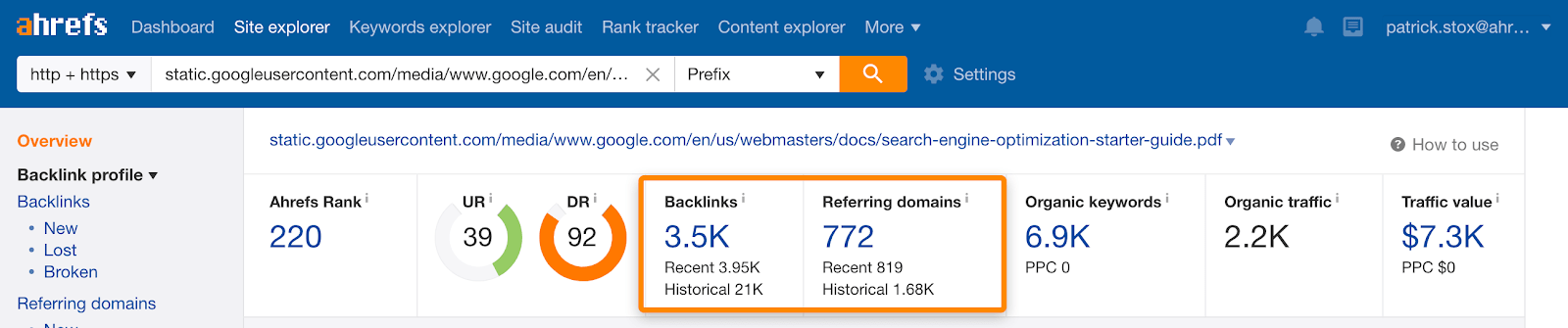Links to Google SEO Starter Guide PDF.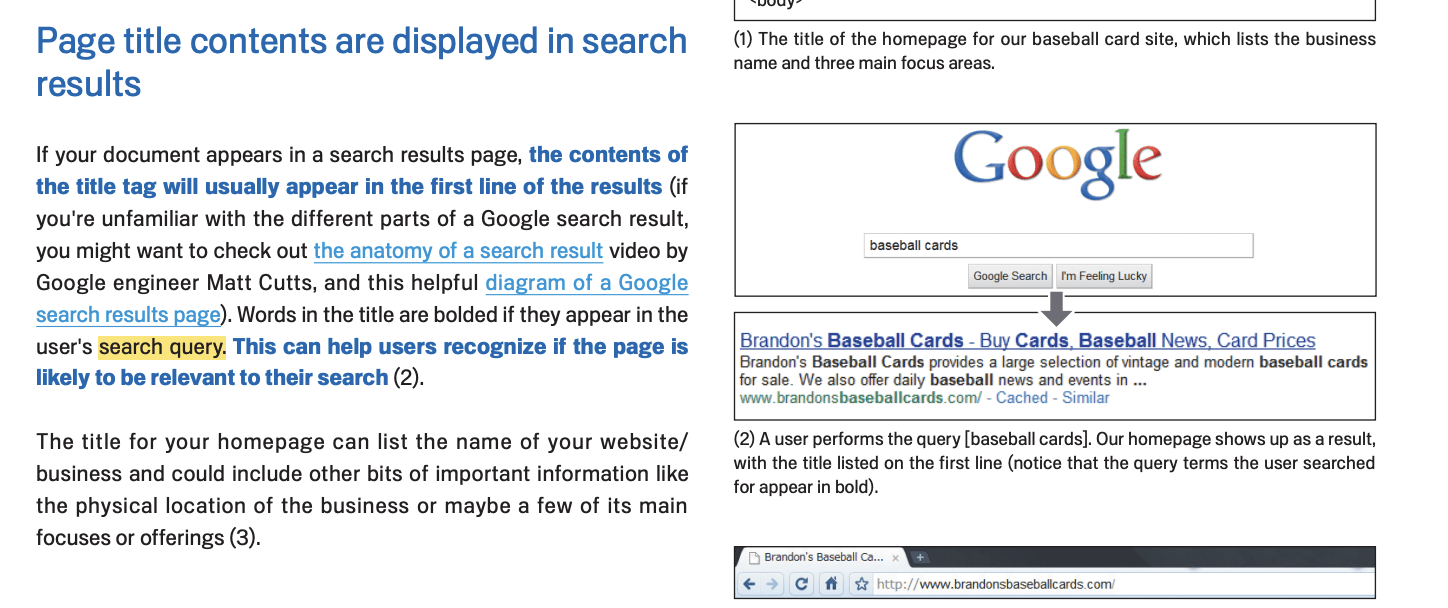Google does a good job of internal linking in the Google SEO Starter Guide PDF.

To add links in a PDF:

1. Click the Edit PDF button on the right sidebar
2. Click the Link dropdown on the Edit menu
3. Click Add/Edit Web or Document Link
4. Draw a rectangle around the text you want to link
5. Set the Link type to Invisible Rectangle
6. Set the Link Action to Open a web page
7. Add your URL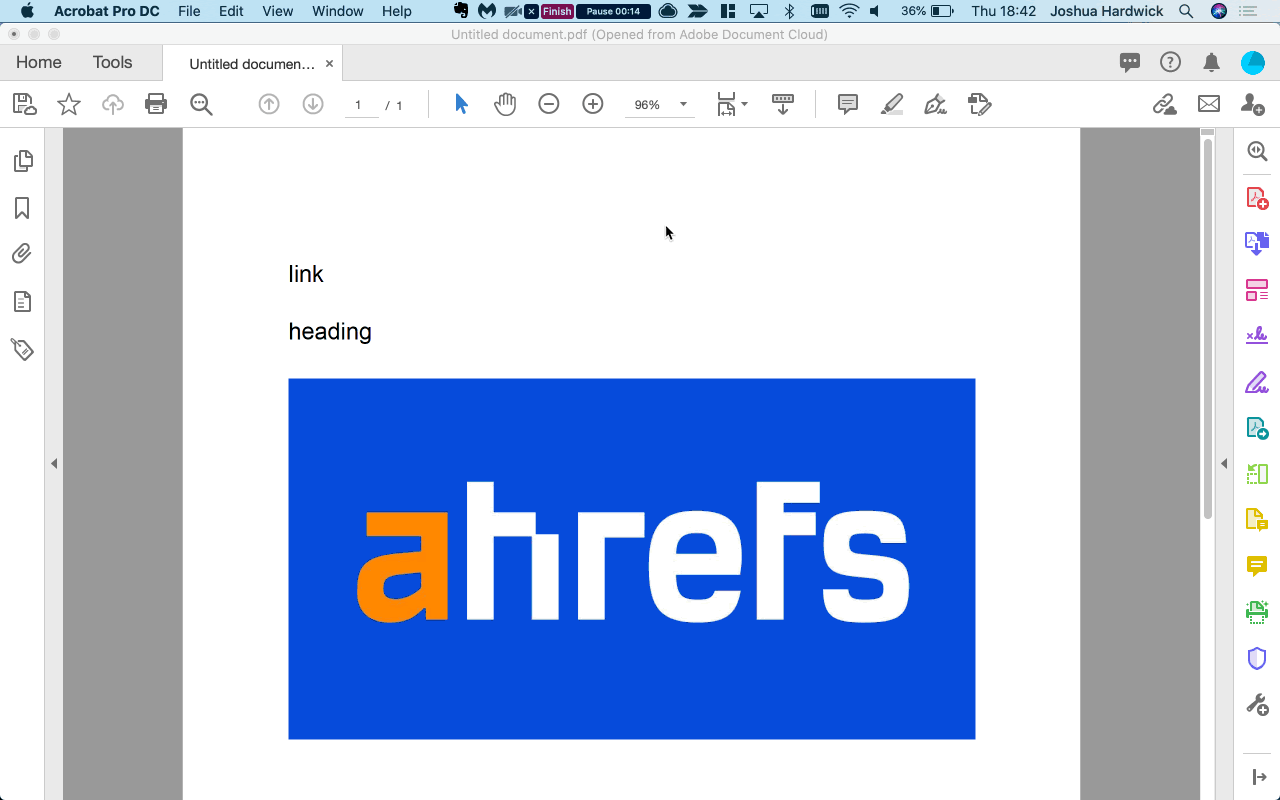Sidenote.

The screenshots and instructions above are for Acrobat Pro DC and may vary depending on the software you use.

As we mentioned previously, PDFs are more difficult to track. Because of this, many marketing teams tend to gate PDFs or make them available only after a user fills out a form. By doing this, they shift the focus from tracking performance to lead generation. However, there are some options to track your PDFs including:

### Event tracking

You can track clicks on PDF links and send them to your analytics system. This allows you to see how many times people clicked on the PDF files to download or open them. You can find out how to set these up here.

### Embeds

If you embed the PDF into a page using JavaScript or an iframe, you can just use the analytics data for the page itself.

### Intermediate tracking script

This is a complex solution, but it’s possible to send PDF clicks through an intermediate tracking script that sends data to your analytics system before sending people to your PDF. You can find one example here.

### Server logs

Because PDF files are stored on a server, any access requests for the files will be recorded in your log files.

### 3rd-party data

Because PDFs are rarely tracked in analytics systems, sometimes the best data you have is from another source like Google Search Console or Ahrefs. Ahrefs can also give you data on which of your competitors’ PDFs get the most organic traffic. Just paste their domain into Site Explorer, then go to the Top Pages report and search for URLs containing `.pdf`

```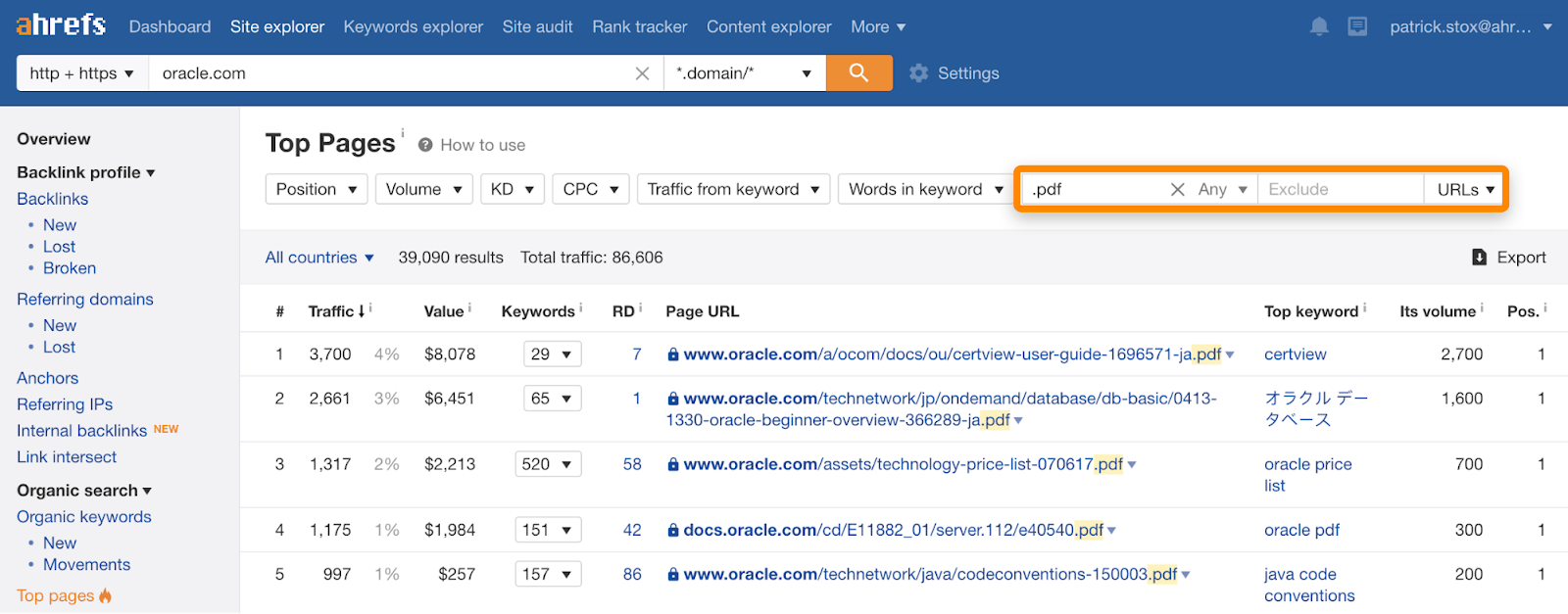Final thoughts Hopefully I’ve convinced you that in most cases you should create new content in web pages and not in PDFs, but what about old PDFs, should you optimize the PDF or change them into pages? In typical SEO fashion, I’m going to go with “it depends”. I really don’t think there’s a right or wrong way to do this. Do what is easier for you. Either way should show a positive impact, but depending on the effort and resources the answer could be optimize PDFs, change PDFs into pages, or do something else instead. Have questions? Let me know on Twitter. ```
``` Source link https://www.sharethelinks.com/2020/07/16/how-to-optimize-pdfs-for-seo-7-steps/ ```
``` ```
``` Marketing Mohamed Elarby A tech blog focused on blogging tips, SEO, social media, mobile gadgets, pc tips, how-to guides and general tips and tricks Previous Post Next Post You might like ```
``` var disqus_shortname = disqusShortname; var disqus_blogger_current_url = "https://www.goodnews4youonline.com/2020/07/how-to-optimize-pdfs-for-seo-7-steps.html"; if (!disqus_blogger_current_url.length) { disqus_blogger_current_url = "https://www.goodnews4youonline.com/2020/07/how-to-optimize-pdfs-for-seo-7-steps.html"; } var disqus_blogger_homepage_url = "https://www.goodnews4youonline.com/"; var disqus_blogger_canonical_homepage_url = "https://www.goodnews4youonline.com/"; //<![CDATA[ if (commentsSystem == 'disqus') { (function() { var bloggerjs = document.createElement('script'); bloggerjs.type = 'text/javascript'; bloggerjs.async = true; bloggerjs.src = '//' + disqus_shortname + '.disqus.com/blogger_item.js'; (document.getElementsByTagName('head') || document.getElementsByTagName('body')).appendChild(bloggerjs); })(); } //]]> Post a Comment Post a Comment BLOG_CMT_createIframe('https://www.blogger.com/rpc_relay.html'); ```
``` var messages = { postedBy: "by", postedOn: "-", postAuthor: "true", postDate: "true" } ```
``` (adsbygoogle = window.adsbygoogle || []).push({}); (adsbygoogle = window.adsbygoogle || []).push({}); (adsbygoogle = window.adsbygoogle || []).push({}); ```
``` Popular Posts 102 Christmas Greeting Cards Collection 6 Unique Animal Designs with Envelopes for Winter Merry Christmas Season, Holiday Giving, Xmas Cute Gifts Cards The 10 Best Websites to Play Free Jigsaw Puzzles Online How to Run Doom on Raspberry Pi (Without an Emulator) Categories infographic(245) Marketing(1407) News(1449) Uncategorized(11) أخبار(157) اخبار الألعاب(10) التدوين(63) التسويق SEO(32) اندرويد(21) انفوجرافيك(55) تقنية(58) جوجل(70) مواقع التواصل الاجتماعي(168) ```
``` ```
``` (adsbygoogle = window.adsbygoogle || []).push({});About Us There are many variations of passages of Lorem Ipsum available, but the majority... Follow Us Design by - Blogger Templates Home About Contact ```
``` //<![CDATA[ /*! Theia Sticky Sidebar | v1.7.0 - https://github.com/WeCodePixels/theia-sticky-sidebar */ (function(\$){\$.fn.theiaStickySidebar=function(options){var defaults={'containerSelector':'','additionalMarginTop':0,'additionalMarginBottom':0,'updateSidebarHeight':true,'minWidth':0,'disableOnResponsiveLayouts':true,'sidebarBehavior':'modern','defaultPosition':'relative','namespace':'TSS'};options=\$.extend(defaults,options);options.additionalMarginTop=parseInt(options.additionalMarginTop)||0;options.additionalMarginBottom=parseInt(options.additionalMarginBottom)||0;tryInitOrHookIntoEvents(options,this);function tryInitOrHookIntoEvents(options,\$that){var success=tryInit(options,\$that);if(!success){console.log('TSS: Body width smaller than options.minWidth. Init is delayed.');\$(document).on('scroll.'+options.namespace,function(options,\$that){return function(evt){var success=tryInit(options,\$that);if(success){\$(this).unbind(evt)}}}(options,\$that));\$(window).on('resize.'+options.namespace,function(options,\$that){return function(evt){var success=tryInit(options,\$that);if(success){\$(this).unbind(evt)}}}(options,\$that))}}function tryInit(options,\$that){if(options.initialized===true){return true}if(\$('body').width()<options.minWidth){return false}init(options,\$that);return true}function init(options,\$that){options.initialized=true;var existingStylesheet=\$('#theia-sticky-sidebar-stylesheet-'+options.namespace);if(existingStylesheet.length===0){\$('head').append(\$('<style id="theia-sticky-sidebar-stylesheet-'+options.namespace+'">.theiaStickySidebar:after {content: ""; display: table; clear: both;}</style>'))}\$that.each(function(){var o={};o.sidebar=\$(this);o.options=options||{};o.container=\$(o.options.containerSelector);if(o.container.length==0){o.container=o.sidebar.parent()}o.sidebar.parents().css('-webkit-transform','none');o.sidebar.css({'position':o.options.defaultPosition,'overflow':'visible','-webkit-box-sizing':'border-box','-moz-box-sizing':'border-box','box-sizing':'border-box'});o.stickySidebar=o.sidebar.find('.theiaStickySidebar');if(o.stickySidebar.length==0){var javaScriptMIMETypes=/(?:text|application)\/(?:x-)?(?:javascript|ecmascript)/i;o.sidebar.find('script').filter(function(index,script){return script.type.length===0||script.type.match(javaScriptMIMETypes)}).remove();o.stickySidebar=\$('<div>').addClass('theiaStickySidebar').append(o.sidebar.children());o.sidebar.append(o.stickySidebar)}o.marginBottom=parseInt(o.sidebar.css('margin-bottom'));o.paddingTop=parseInt(o.sidebar.css('padding-top'));o.paddingBottom=parseInt(o.sidebar.css('padding-bottom'));var collapsedTopHeight=o.stickySidebar.offset().top;var collapsedBottomHeight=o.stickySidebar.outerHeight();o.stickySidebar.css('padding-top',1);o.stickySidebar.css('padding-bottom',1);collapsedTopHeight-=o.stickySidebar.offset().top;collapsedBottomHeight=o.stickySidebar.outerHeight()-collapsedBottomHeight-collapsedTopHeight;if(collapsedTopHeight==0){o.stickySidebar.css('padding-top',0);o.stickySidebarPaddingTop=0}else{o.stickySidebarPaddingTop=1}if(collapsedBottomHeight==0){o.stickySidebar.css('padding-bottom',0);o.stickySidebarPaddingBottom=0}else{o.stickySidebarPaddingBottom=1}o.previousScrollTop=null;o.fixedScrollTop=0;resetSidebar();o.onScroll=function(o){if(!o.stickySidebar.is(":visible")){return}if(\$('body').width()<o.options.minWidth){resetSidebar();return}if(o.options.disableOnResponsiveLayouts){var sidebarWidth=o.sidebar.outerWidth(o.sidebar.css('float')=='none');if(sidebarWidth+50>o.container.width()){resetSidebar();return}}var scrollTop=\$(document).scrollTop();var position='static';if(scrollTop>=o.sidebar.offset().top+(o.paddingTop-o.options.additionalMarginTop)){var offsetTop=o.paddingTop+options.additionalMarginTop;var offsetBottom=o.paddingBottom+o.marginBottom+options.additionalMarginBottom;var containerTop=o.sidebar.offset().top;var containerBottom=o.sidebar.offset().top+getClearedHeight(o.container);var windowOffsetTop=0+options.additionalMarginTop;var windowOffsetBottom;var sidebarSmallerThanWindow=(o.stickySidebar.outerHeight()+offsetTop+offsetBottom)<\$(window).height();if(sidebarSmallerThanWindow){windowOffsetBottom=windowOffsetTop+o.stickySidebar.outerHeight()}else{windowOffsetBottom=\$(window).height()-o.marginBottom-o.paddingBottom-options.additionalMarginBottom}var staticLimitTop=containerTop-scrollTop+o.paddingTop;var staticLimitBottom=containerBottom-scrollTop-o.paddingBottom-o.marginBottom;var top=o.stickySidebar.offset().top-scrollTop;var scrollTopDiff=o.previousScrollTop-scrollTop;if(o.stickySidebar.css('position')=='fixed'){if(o.options.sidebarBehavior=='modern'){top+=scrollTopDiff}}if(o.options.sidebarBehavior=='stick-to-top'){top=options.additionalMarginTop}if(o.options.sidebarBehavior=='stick-to-bottom'){top=windowOffsetBottom-o.stickySidebar.outerHeight()}if(scrollTopDiff>0){top=Math.min(top,windowOffsetTop)}else{top=Math.max(top,windowOffsetBottom-o.stickySidebar.outerHeight())}top=Math.max(top,staticLimitTop);top=Math.min(top,staticLimitBottom-o.stickySidebar.outerHeight());var sidebarSameHeightAsContainer=o.container.height()==o.stickySidebar.outerHeight();if(!sidebarSameHeightAsContainer&&top==windowOffsetTop){position='fixed'}else if(!sidebarSameHeightAsContainer&&top==windowOffsetBottom-o.stickySidebar.outerHeight()){position='fixed'}else if(scrollTop+top-o.sidebar.offset().top-o.paddingTop<=options.additionalMarginTop){position='static'}else{position='absolute'}}if(position=='fixed'){var scrollLeft=\$(document).scrollLeft();o.stickySidebar.css({'position':'fixed','width':getWidthForObject(o.stickySidebar)+'px','transform':'translateY('+top+'px)','left':(o.sidebar.offset().left+parseInt(o.sidebar.css('padding-left'))-scrollLeft)+'px','top':'0px'})}else if(position=='absolute'){var css={};if(o.stickySidebar.css('position')!='absolute'){css.position='absolute';css.transform='translateY('+(scrollTop+top-o.sidebar.offset().top-o.stickySidebarPaddingTop-o.stickySidebarPaddingBottom)+'px)';css.top='0px'}css.width=getWidthForObject(o.stickySidebar)+'px';css.left='';o.stickySidebar.css(css)}else if(position=='static'){resetSidebar()}if(position!='static'){if(o.options.updateSidebarHeight==true){o.sidebar.css({'min-height':o.stickySidebar.outerHeight()+o.stickySidebar.offset().top-o.sidebar.offset().top+o.paddingBottom})}}o.previousScrollTop=scrollTop};o.onScroll(o);\$(document).on('scroll.'+o.options.namespace,function(o){return function(){o.onScroll(o)}}(o));\$(window).on('resize.'+o.options.namespace,function(o){return function(){o.stickySidebar.css({'position':'static'});o.onScroll(o)}}(o));if(typeof ResizeSensor!=='undefined'){new ResizeSensor(o.stickySidebar,function(o){return function(){o.onScroll(o)}}(o))}function resetSidebar(){o.fixedScrollTop=0;o.sidebar.css({'min-height':'1px'});o.stickySidebar.css({'position':'static','width':'','transform':'none'})}function getClearedHeight(e){var height=e.height();e.children().each(function(){height=Math.max(height,\$(this).height())});return height}})}function getWidthForObject(object){var width;try{width=object.getBoundingClientRect().width}catch(err){}if(typeof width==="undefined"){width=object.width()}return width}return this}})(jQuery); /*! Shortcode.js by @nicinabox | v1.1.0 - https://github.com/nicinabox/shortcode.js */ var Shortcode=function(el,tags){if(!el){return}this.el=el;this.tags=tags;this.matches=[];this.regex='\\[{name}(\\s[\\s\\S]*?)?\\]'+'(?:((?!\\s*?(?:\\[{name}|\\[\\/(?!{name})))[\\s\\S]*?)'+'(\\[\/{name}\\]))?';if(this.el.jquery){this.el=this.el}this.matchTags();this.convertMatchesToNodes();this.replaceNodes()};Shortcode.prototype.matchTags=function(){var html=this.el.outerHTML,instances,match,re,contents,regex,tag,options;for(var key in this.tags){if(!this.tags.hasOwnProperty(key)){return}re=this.template(this.regex,{name:key});instances=html.match(new RegExp(re,'g'))||[];for(var i=0,len=instances.length;i<len;i++){match=instances[i].match(new RegExp(re));contents=match?'':undefined;tag=match;regex=this.escapeTagRegExp(tag);options=this.parseOptions(match);if(match){contents=match.trim();tag=tag.replace(contents,'').replace(/\n\s*/g,'');regex=this.escapeTagRegExp(tag).replace('\\]\\[','\\]([\\s\\S]*?)\\[')}this.matches.push({name:key,tag:tag,regex:regex,options:options,contents:contents})}}};Shortcode.prototype.convertMatchesToNodes=function(){var html=this.el.innerHTML,excludes,re,replacer;replacer=function(match,p1,p2,p3,p4,offset,string){if(p1){return match}else{var node=document.createElement('span');node.setAttribute('data-sc-tag',this.tag);node.className='templateify-sc-node templateify-sc-node-'+this.name;return node.outerHTML}};for(var i=0,len=this.matches.length;i<len;i++){excludes='((data-sc-tag=")|(<pre.*)|(<code.*))?';re=new RegExp(excludes+this.matches[i].regex,'g');html=html.replace(re,replacer.bind(this.matches[i]))}this.el.innerHTML=html};Shortcode.prototype.replaceNodes=function(){var self=this,html,match,result,done,node,fn,replacer,nodes=this.el.querySelectorAll('.templateify-sc-node');replacer=function(result){if(result.jquery){result=result}result=self.parseCallbackResult(result);node.parentNode.replaceChild(result,node)};for(var i=0,len=this.matches.length;i<len;i++){match=this.matches[i];node=this.el.querySelector('.templateify-sc-node-'+match.name);if(node&&node.dataset.scTag===match.tag){fn=this.tags[match.name].bind(match);done=replacer.bind(match);result=fn(done);if(result!==undefined){done(result)}}}};Shortcode.prototype.parseCallbackResult=function(result){var container,fragment,children;switch(typeof result){case'function':result=document.createTextNode(result());break;case'string':container=document.createElement('div');fragment=document.createDocumentFragment();container.innerHTML=result;children=container.childNodes;if(children.length){for(var i=0,len=children.length;i<len;i++){fragment.appendChild(children[i].cloneNode(true))}result=fragment}else{result=document.createTextNode(result)}break;case'object':if(!result.nodeType){result=JSON.stringify(result);result=document.createTextNode(result)}break;case'default':break}return result};Shortcode.prototype.parseOptions=function(stringOptions){var options={},set;if(!stringOptions){return}set=stringOptions.replace(/(\w+=)/g,'\n\$1').split('\n');set.shift();for(var i=0;i<set.length;i++){var kv=set[i].split('=');options[kv]=kv.replace(/\'|\"/g,'').trim()}return options};Shortcode.prototype.escapeTagRegExp=function(regex){return regex.replace(/[\[\]\/]/g,'\\\$&')};Shortcode.prototype.template=function(s,d){for(var p in d){s=s.replace(new RegExp('{'+p+'}','g'),d[p])}return s};String.prototype.trim=String.prototype.trim||function(){return this.replace(/^\s+|\s+\$/g,'')};if(window.jQuery){var pluginName='shortcode';\$.fn[pluginName]=function(tags){this.each(function(){if(!\$.data(this,pluginName)){\$.data(this,pluginName,new Shortcode(this,tags))}});return this}} /*! ResizeIfy - LazyIfy on Scroll by Templateify | v1.2.0 - https://www.templateify.com */ !function(a){a.fn.lazyify=function(){return this.each(function(){var t=a(this),dImg=t.attr('data-image'),iWid=Math.round(t.width()),iHei=Math.round(t.height()),iSiz='w'+iWid+'-h'+iHei+'-p-k-no-nu',img='';if(dImg.match('/s72-c')){img=dImg.replace('/s72-c','/'+iSiz);}else if(dImg.match('/w72-h')){img=dImg.replace('/w72-h72-p-k-no-nu','/'+iSiz);}else if(dImg.match('=w72-h')){img=dImg.replace('=w72-h72-p-k-no-nu','='+iSiz);}else{img=dImg;} a(window).on('load resize scroll',lazyOnScroll);function lazyOnScroll(){var wHeight=a(window).height(),scrTop=a(window).scrollTop(),offTop=t.offset().top;if(scrTop+wHeight>offTop){var n=new Image();n.onload=function(){t.attr('style','background-image:url('+this.src+')').addClass('lazy-ify');},n.src=img;}} lazyOnScroll();});}}(jQuery); /*! jQuery replaceText by "Cowboy" Ben Alman | v1.1.0 - http://benalman.com/projects/jquery-replacetext-plugin/ */ (function(\$){\$.fn.replaceText=function(b,a,c){return this.each(function(){var f=this.firstChild,g,e,d=[];if(f){do{if(f.nodeType===3){g=f.nodeValue;e=g.replace(b,a);if(e!==g){if(!c&&/</.test(e)){\$(f).before(e);d.push(f)}else{f.nodeValue=e}}}}while(f=f.nextSibling)}d.length&&\$(d).remove()})}})(jQuery); //]]> //<![CDATA[ var _\$_obify3=["\x4B\x20\x61\x24\x68\x3D\x5B\x22\x5C\x64\x5C\x6A\x5C\x6B\x5C\x71\x22\x2C\x22\x5C\x6F\x5C\x65\x5C\x64\x5C\x65\x5C\x6D\x5C\x64\x5C\x6B\x5C\x64\x5C\x6C\x5C\x63\x22\x2C\x22\x5C\x65\x5C\x64\x5C\x64\x5C\x6A\x22\x2C\x22\x5C\x64\x5C\x63\x5C\x56\x5C\x64\x22\x2C\x22\x5C\x78\x5C\x71\x5C\x63\x5C\x6E\x5C\x45\x5C\x6D\x5C\x64\x5C\x69\x5C\x46\x5C\x46\x5C\x6C\x5C\x63\x22\x2C\x22\x5C\x63\x5C\x65\x5C\x74\x5C\x41\x22\x2C\x22\x5C\x51\x5C\x71\x5C\x65\x5C\x6B\x5C\x6E\x5C\x6D\x5C\x71\x5C\x63\x5C\x6E\x5C\x45\x5C\x6D\x5C\x45\x5C\x6C\x22\x2C\x22\x5C\x74\x5C\x6C\x5C\x6B\x5C\x74\x5C\x59\x22\x2C\x22\x5C\x66\x5C\x63\x5C\x65\x5C\x6A\x5C\x74\x5C\x41\x5C\x6D\x5C\x65\x5C\x74\x5C\x64\x5C\x6B\x5C\x50\x5C\x63\x22\x2C\x22\x5C\x64\x5C\x69\x5C\x46\x5C\x46\x5C\x6C\x5C\x63\x5C\x31\x6D\x5C\x6C\x5C\x65\x5C\x66\x5C\x66\x22\x2C\x22\x5C\x79\x5C\x69\x5C\x6F\x5C\x47\x22\x2C\x22\x5C\x69\x5C\x6E\x22\x2C\x22\x5C\x78\x5C\x66\x5C\x63\x5C\x65\x5C\x6A\x5C\x74\x5C\x41\x5C\x6D\x5C\x64\x5C\x69\x5C\x46\x5C\x46\x5C\x6C\x5C\x63\x22\x2C\x22\x5C\x6F\x5C\x65\x5C\x64\x5C\x65\x5C\x6D\x5C\x64\x5C\x63\x5C\x56\x5C\x64\x22\x2C\x22\x22\x2C\x22\x5C\x41\x5C\x6B\x5C\x6F\x5C\x63\x22\x2C\x22\x5C\x78\x5C\x44\x5C\x69\x5C\x6C\x5C\x6C\x5C\x69\x5C\x4D\x5C\x6D\x5C\x79\x5C\x47\x5C\x6D\x5C\x63\x5C\x71\x5C\x65\x5C\x6B\x5C\x6C\x5C\x6D\x5C\x64\x5C\x63\x5C\x56\x5C\x64\x22\x2C\x22\x5C\x69\x5C\x72\x5C\x64\x5C\x6B\x5C\x69\x5C\x6E\x5C\x66\x22\x2C\x22\x5C\x6B\x5C\x6F\x22\x2C\x22\x5C\x43\x5C\x6F\x5C\x6B\x5C\x50\x5C\x70\x5C\x6B\x5C\x6F\x5C\x48\x5C\x75\x5C\x6E\x5C\x63\x5C\x4D\x5C\x6D\x5C\x79\x5C\x63\x5C\x44\x5C\x69\x5C\x6A\x5C\x63\x5C\x6D\x5C\x65\x5C\x6F\x5C\x75\x5C\x7A\x5C\x42\x22\x2C\x22\x5C\x65\x5C\x6F\x5C\x66\x5C\x31\x6A\x22\x2C\x22\x5C\x43\x5C\x6F\x5C\x6B\x5C\x50\x5C\x70\x5C\x6B\x5C\x6F\x5C\x48\x5C\x75\x5C\x6E\x5C\x63\x5C\x4D\x5C\x6D\x5C\x65\x5C\x44\x5C\x64\x5C\x63\x5C\x6A\x5C\x6D\x5C\x65\x5C\x6F\x5C\x75\x5C\x7A\x5C\x42\x22\x2C\x22\x5C\x65\x5C\x6F\x5C\x66\x5C\x31\x6C\x22\x2C\x22\x5C\x66\x5C\x41\x5C\x69\x5C\x6A\x5C\x64\x5C\x74\x5C\x69\x5C\x6F\x5C\x63\x22\x2C\x22\x5C\x51\x5C\x72\x5C\x69\x5C\x66\x5C\x64\x5C\x6D\x5C\x79\x5C\x69\x5C\x6F\x5C\x47\x22\x2C\x22\x5C\x6C\x5C\x63\x5C\x6E\x5C\x46\x5C\x64\x5C\x41\x22\x2C\x22\x5C\x65\x5C\x72\x5C\x72\x5C\x63\x5C\x6E\x5C\x6F\x5C\x31\x73\x5C\x69\x22\x2C\x22\x5C\x51\x5C\x79\x5C\x63\x5C\x44\x5C\x69\x5C\x6A\x5C\x63\x5C\x6D\x5C\x65\x5C\x6F\x22\x2C\x22\x5C\x51\x5C\x6E\x5C\x63\x5C\x4D\x5C\x6D\x5C\x79\x5C\x63\x5C\x44\x5C\x69\x5C\x6A\x5C\x63\x5C\x6D\x5C\x65\x5C\x6F\x22\x2C\x22\x5C\x51\x5C\x65\x5C\x44\x5C\x64\x5C\x63\x5C\x6A\x5C\x6D\x5C\x65\x5C\x6F\x22\x2C\x22\x5C\x51\x5C\x6E\x5C\x63\x5C\x4D\x5C\x6D\x5C\x65\x5C\x44\x5C\x64\x5C\x63\x5C\x6A\x5C\x6D\x5C\x65\x5C\x6F\x22\x2C\x22\x5C\x51\x5C\x71\x5C\x65\x5C\x6B\x5C\x6E\x5C\x6D\x5C\x79\x5C\x63\x5C\x44\x5C\x69\x5C\x6A\x5C\x63\x5C\x6D\x5C\x65\x5C\x6F\x5C\x70\x5C\x78\x5C\x4D\x5C\x6B\x5C\x6F\x5C\x46\x5C\x63\x5C\x64\x22\x2C\x22\x5C\x51\x5C\x71\x5C\x65\x5C\x6B\x5C\x6E\x5C\x6D\x5C\x65\x5C\x44\x5C\x64\x5C\x63\x5C\x6A\x5C\x6D\x5C\x65\x5C\x6F\x5C\x70\x5C\x78\x5C\x4D\x5C\x6B\x5C\x6F\x5C\x46\x5C\x63\x5C\x64\x22\x2C\x22\x5C\x51\x5C\x65\x5C\x6A\x5C\x63\x5C\x6C\x5C\x6D\x5C\x65\x5C\x6F\x22\x2C\x22\x5C\x51\x5C\x71\x5C\x65\x5C\x6B\x5C\x6E\x5C\x6D\x5C\x65\x5C\x6A\x5C\x63\x5C\x6C\x5C\x6D\x5C\x65\x5C\x6F\x5C\x70\x5C\x78\x5C\x4D\x5C\x6B\x5C\x6F\x5C\x46\x5C\x63\x5C\x64\x22\x2C\x22\x5C\x66\x5C\x6A\x5C\x74\x22\x2C\x22\x5C\x7A\x5C\x7A\x5C\x6A\x5C\x63\x5C\x66\x5C\x69\x5C\x45\x5C\x6A\x5C\x74\x5C\x63\x5C\x66\x5C\x78\x5C\x79\x5C\x6C\x5C\x69\x5C\x46\x5C\x79\x5C\x6C\x5C\x69\x5C\x46\x5C\x78\x5C\x74\x5C\x69\x5C\x71\x5C\x7A\x5C\x6B\x5C\x71\x5C\x46\x5C\x7A\x5C\x79\x5C\x6C\x5C\x65\x5C\x6E\x5C\x59\x5C\x78\x5C\x46\x5C\x6B\x5C\x44\x22\x2C\x22\x5C\x7A\x5C\x7A\x5C\x31\x6A\x5C\x78\x5C\x79\x5C\x72\x5C\x78\x5C\x79\x5C\x6C\x5C\x69\x5C\x46\x5C\x66\x5C\x72\x5C\x69\x5C\x64\x5C\x78\x5C\x74\x5C\x69\x5C\x71\x5C\x7A\x5C\x6D\x5C\x69\x5C\x31\x7A\x5C\x31\x75\x5C\x33\x6D\x5C\x34\x59\x5C\x31\x76\x5C\x31\x66\x5C\x32\x72\x5C\x32\x59\x5C\x56\x5C\x69\x5C\x7A\x5C\x33\x42\x5C\x47\x5C\x31\x6A\x5C\x34\x63\x5C\x32\x72\x5C\x6F\x5C\x72\x5C\x32\x79\x5C\x79\x5C\x31\x66\x5C\x32\x68\x5C\x7A\x5C\x57\x5C\x57\x5C\x57\x5C\x57\x5C\x57\x5C\x57\x5C\x57\x5C\x57\x5C\x31\x6D\x5C\x31\x76\x5C\x31\x66\x5C\x7A\x5C\x46\x5C\x46\x5C\x74\x5C\x35\x63\x5C\x44\x5C\x31\x53\x5C\x31\x6D\x5C\x31\x76\x5C\x32\x65\x5C\x32\x72\x5C\x66\x5C\x31\x6C\x5C\x31\x7A\x5C\x31\x7A\x5C\x65\x5C\x63\x5C\x31\x53\x5C\x32\x72\x5C\x31\x54\x5C\x31\x76\x5C\x31\x7A\x5C\x33\x7A\x5C\x31\x6C\x5C\x4D\x5C\x47\x5C\x31\x73\x5C\x31\x43\x5C\x31\x55\x5C\x32\x65\x5C\x65\x5C\x31\x73\x5C\x47\x5C\x32\x65\x5C\x31\x6D\x5C\x34\x50\x5C\x31\x45\x5C\x31\x54\x5C\x33\x58\x5C\x57\x5C\x31\x43\x5C\x31\x43\x5C\x31\x6D\x5C\x4D\x5C\x7A\x5C\x66\x5C\x33\x72\x5C\x32\x7A\x5C\x6D\x5C\x6A\x5C\x7A\x5C\x65\x5C\x50\x5C\x65\x5C\x64\x5C\x65\x5C\x6A\x5C\x78\x5C\x31\x75\x5C\x72\x5C\x46\x22\x2C\x22\x5C\x6A\x5C\x63\x5C\x72\x5C\x6C\x5C\x65\x5C\x74\x5C\x63\x22\x2C\x22\x5C\x7A\x5C\x7A\x5C\x6B\x5C\x71\x5C\x46\x5C\x31\x6A\x5C\x78\x5C\x79\x5C\x6C\x5C\x69\x5C\x46\x5C\x79\x5C\x6C\x5C\x69\x5C\x46\x5C\x78\x5C\x74\x5C\x69\x5C\x71\x5C\x7A\x5C\x6B\x5C\x71\x5C\x46\x5C\x7A\x5C\x79\x5C\x6C\x5C\x65\x5C\x6E\x5C\x59\x5C\x78\x5C\x46\x5C\x6B\x5C\x44\x22\x2C\x22\x5C\x78\x5C\x65\x5C\x50\x5C\x65\x5C\x64\x5C\x65\x5C\x6A\x5C\x6D\x5C\x6B\x5C\x71\x5C\x65\x5C\x46\x5C\x63\x5C\x6D\x5C\x74\x5C\x69\x5C\x6E\x5C\x64\x5C\x65\x5C\x6B\x5C\x6E\x5C\x63\x5C\x6A\x5C\x70\x5C\x6B\x5C\x71\x5C\x46\x22\x2C\x22\x5C\x7A\x22\x2C\x22\x5C\x66\x5C\x72\x5C\x6C\x5C\x6B\x5C\x64\x22\x2C\x22\x5C\x72\x5C\x69\x5C\x72\x22\x2C\x22\x5C\x79\x5C\x45\x5C\x64\x5C\x64\x5C\x69\x5C\x6E\x22\x2C\x22\x5C\x71\x5C\x65\x5C\x64\x5C\x74\x5C\x41\x22\x2C\x22\x5C\x65\x5C\x6F\x5C\x6F\x5C\x31\x6D\x5C\x6C\x5C\x65\x5C\x66\x5C\x66\x22\x2C\x22\x5C\x74\x5C\x66\x5C\x66\x22\x2C\x22\x5C\x74\x5C\x69\x5C\x6C\x5C\x69\x5C\x6A\x5C\x63\x5C\x6F\x5C\x6D\x5C\x79\x5C\x45\x5C\x64\x5C\x64\x5C\x69\x5C\x6E\x22\x2C\x22\x5C\x78\x5C\x72\x5C\x69\x5C\x66\x5C\x64\x5C\x6D\x5C\x79\x5C\x69\x5C\x6F\x5C\x47\x5C\x70\x5C\x65\x22\x2C\x22\x5C\x41\x5C\x64\x5C\x71\x5C\x6C\x22\x2C\x22\x5C\x74\x5C\x69\x5C\x6E\x5C\x64\x5C\x65\x5C\x74\x5C\x64\x5C\x6D\x5C\x44\x5C\x69\x5C\x6A\x5C\x71\x22\x2C\x22\x5C\x43\x5C\x6F\x5C\x6B\x5C\x50\x5C\x70\x5C\x74\x5C\x6C\x5C\x65\x5C\x66\x5C\x66\x5C\x48\x5C\x75\x5C\x74\x5C\x69\x5C\x6E\x5C\x64\x5C\x65\x5C\x74\x5C\x64\x5C\x6D\x5C\x44\x5C\x69\x5C\x6A\x5C\x71\x5C\x75\x5C\x7A\x5C\x42\x22\x2C\x22\x5C\x6A\x5C\x63\x5C\x72\x5C\x6C\x5C\x65\x5C\x74\x5C\x63\x5C\x33\x42\x5C\x6B\x5C\x64\x5C\x41\x22\x2C\x22\x5C\x51\x5C\x31\x6D\x5C\x69\x5C\x6E\x5C\x64\x5C\x65\x5C\x74\x5C\x64\x5C\x31\x76\x5C\x69\x5C\x6A\x5C\x71\x5C\x31\x6A\x22\x2C\x22\x5C\x65\x5C\x72\x5C\x72\x5C\x63\x5C\x6E\x5C\x6F\x22\x2C\x22\x5C\x78\x5C\x74\x5C\x69\x5C\x6E\x5C\x64\x5C\x65\x5C\x74\x5C\x64\x5C\x6D\x5C\x44\x5C\x69\x5C\x6A\x5C\x71\x22\x2C\x22\x5C\x65\x5C\x6C\x5C\x63\x5C\x6A\x5C\x64\x5C\x6D\x5C\x66\x5C\x45\x5C\x74\x5C\x74\x5C\x63\x5C\x66\x5C\x66\x22\x2C\x22\x5C\x43\x5C\x6F\x5C\x6B\x5C\x50\x5C\x70\x5C\x74\x5C\x6C\x5C\x65\x5C\x66\x5C\x66\x5C\x48\x5C\x75\x5C\x65\x5C\x6C\x5C\x63\x5C\x6A\x5C\x64\x5C\x6D\x5C\x71\x5C\x63\x5C\x66\x5C\x66\x5C\x65\x5C\x46\x5C\x63\x5C\x70\x5C\x65\x5C\x6C\x5C\x63\x5C\x6A\x5C\x64\x5C\x6D\x5C\x66\x5C\x45\x5C\x74\x5C\x74\x5C\x63\x5C\x66\x5C\x66\x5C\x70\x5C\x66\x5C\x41\x5C\x69\x5C\x6A\x5C\x64\x5C\x6D\x5C\x79\x5C\x75\x5C\x42\x22\x2C\x22\x5C\x43\x5C\x7A\x5C\x6F\x5C\x6B\x5C\x50\x5C\x42\x22\x2C\x22\x5C\x65\x5C\x6C\x5C\x63\x5C\x6A\x5C\x64\x5C\x6D\x5C\x6B\x5C\x6E\x5C\x44\x5C\x69\x22\x2C\x22\x5C\x43\x5C\x6F\x5C\x6B\x5C\x50\x5C\x70\x5C\x74\x5C\x6C\x5C\x65\x5C\x66\x5C\x66\x5C\x48\x5C\x75\x5C\x65\x5C\x6C\x5C\x63\x5C\x6A\x5C\x64\x5C\x6D\x5C\x71\x5C\x63\x5C\x66\x5C\x66\x5C\x65\x5C\x46\x5C\x63\x5C\x70\x5C\x65\x5C\x6C\x5C\x63\x5C\x6A\x5C\x64\x5C\x6D\x5C\x6B\x5C\x6E\x5C\x44\x5C\x69\x5C\x70\x5C\x66\x5C\x41\x5C\x69\x5C\x6A\x5C\x64\x5C\x6D\x5C\x79\x5C\x75\x5C\x42\x22\x2C\x22\x5C\x65\x5C\x6C\x5C\x63\x5C\x6A\x5C\x64\x5C\x6D\x5C\x4D\x5C\x65\x5C\x6A\x5C\x6E\x5C\x6B\x5C\x6E\x5C\x46\x22\x2C\x22\x5C\x43\x5C\x6F\x5C\x6B\x5C\x50\x5C\x70\x5C\x74\x5C\x6C\x5C\x65\x5C\x66\x5C\x66\x5C\x48\x5C\x75\x5C\x65\x5C\x6C\x5C\x63\x5C\x6A\x5C\x64\x5C\x6D\x5C\x71\x5C\x63\x5C\x66\x5C\x66\x5C\x65\x5C\x46\x5C\x63\x5C\x70\x5C\x65\x5C\x6C\x5C\x63\x5C\x6A\x5C\x64\x5C\x6D\x5C\x4D\x5C\x65\x5C\x6A\x5C\x6E\x5C\x6B\x5C\x6E\x5C\x46\x5C\x70\x5C\x66\x5C\x41\x5C\x69\x5C\x6A\x5C\x64\x5C\x6D\x5C\x79\x5C\x75\x5C\x42\x22\x2C\x22\x5C\x65\x5C\x6C\x5C\x63\x5C\x6A\x5C\x64\x5C\x6D\x5C\x63\x5C\x6A\x5C\x6A\x5C\x69\x5C\x6A\x22\x2C\x22\x5C\x43\x5C\x6F\x5C\x6B\x5C\x50\x5C\x70\x5C\x74\x5C\x6C\x5C\x65\x5C\x66\x5C\x66\x5C\x48\x5C\x75\x5C\x65\x5C\x6C\x5C\x63\x5C\x6A\x5C\x64\x5C\x6D\x5C\x71\x5C\x63\x5C\x66\x5C\x66\x5C\x65\x5C\x46\x5C\x63\x5C\x70\x5C\x65\x5C\x6C\x5C\x63\x5C\x6A\x5C\x64\x5C\x6D\x5C\x63\x5C\x6A\x5C\x6A\x5C\x69\x5C\x6A\x5C\x70\x5C\x66\x5C\x41\x5C\x69\x5C\x6A\x5C\x64\x5C\x6D\x5C\x79\x5C\x75\x5C\x42\x22\x2C\x22\x5C\x6C\x5C\x63\x5C\x44\x5C\x64\x5C\x6D\x5C\x66\x5C\x6B\x5C\x6F\x5C\x63\x5C\x79\x5C\x65\x5C\x6A\x22\x2C\x22\x5C\x43\x5C\x66\x5C\x64\x5C\x47\x5C\x6C\x5C\x63\x5C\x42\x5C\x78\x5C\x6B\x5C\x64\x5C\x63\x5C\x71\x5C\x70\x5C\x51\x5C\x71\x5C\x65\x5C\x6B\x5C\x6E\x5C\x6D\x5C\x4D\x5C\x6A\x5C\x65\x5C\x72\x5C\x72\x5C\x63\x5C\x6A\x5C\x31\x4C\x5C\x44\x5C\x6C\x5C\x69\x5C\x65\x5C\x64\x5C\x5A\x5C\x6A\x5C\x6B\x5C\x46\x5C\x41\x5C\x64\x5C\x31\x4D\x5C\x78\x5C\x6B\x5C\x64\x5C\x63\x5C\x71\x5C\x70\x5C\x51\x5C\x66\x5C\x6B\x5C\x6F\x5C\x63\x5C\x79\x5C\x65\x5C\x6A\x5C\x6D\x5C\x4D\x5C\x6A\x5C\x65\x5C\x72\x5C\x72\x5C\x63\x5C\x6A\x5C\x31\x4C\x5C\x44\x5C\x6C\x5C\x69\x5C\x65\x5C\x64\x5C\x5A\x5C\x6C\x5C\x63\x5C\x44\x5C\x64\x5C\x31\x4D\x5C\x43\x5C\x7A\x5C\x66\x5C\x64\x5C\x47\x5C\x6C\x5C\x63\x5C\x42\x22\x2C\x22\x5C\x6A\x5C\x6B\x5C\x46\x5C\x41\x5C\x64\x5C\x6D\x5C\x66\x5C\x6B\x5C\x6F\x5C\x63\x5C\x79\x5C\x65\x5C\x6A\x22\x2C\x22\x5C\x43\x5C\x66\x5C\x64\x5C\x47\x5C\x6C\x5C\x63\x5C\x42\x5C\x78\x5C\x6B\x5C\x64\x5C\x63\x5C\x71\x5C\x70\x5C\x51\x5C\x71\x5C\x65\x5C\x6B\x5C\x6E\x5C\x6D\x5C\x4D\x5C\x6A\x5C\x65\x5C\x72\x5C\x72\x5C\x63\x5C\x6A\x5C\x31\x4C\x5C\x44\x5C\x6C\x5C\x69\x5C\x65\x5C\x64\x5C\x5A\x5C\x6C\x5C\x63\x5C\x44\x5C\x64\x5C\x31\x4D\x5C\x78\x5C\x6B\x5C\x64\x5C\x63\x5C\x71\x5C\x70\x5C\x51\x5C\x66\x5C\x6B\x5C\x6F\x5C\x63\x5C\x79\x5C\x65\x5C\x6A\x5C\x6D\x5C\x4D\x5C\x6A\x5C\x65\x5C\x72\x5C\x72\x5C\x63\x5C\x6A\x5C\x31\x4C\x5C\x44\x5C\x6C\x5C\x69\x5C\x65\x5C\x64\x5C\x5A\x5C\x6A\x5C\x6B\x5C\x46\x5C\x41\x5C\x64\x5C\x31\x4D\x5C\x43\x5C\x7A\x5C\x66\x5C\x64\x5C\x47\x5C\x6C\x5C\x63\x5C\x42\x22\x2C\x22\x5C\x44\x5C\x45\x5C\x6C\x5C\x6C\x5C\x6D\x5C\x4D\x5C\x6B\x5C\x6F\x5C\x64\x5C\x41\x22\x2C\x22\x5C\x43\x5C\x66\x5C\x64\x5C\x47\x5C\x6C\x5C\x63\x5C\x42\x5C\x78\x5C\x6B\x5C\x64\x5C\x63\x5C\x71\x5C\x70\x5C\x51\x5C\x71\x5C\x65\x5C\x6B\x5C\x6E\x5C\x6D\x5C\x4D\x5C\x6A\x5C\x65\x5C\x72\x5C\x72\x5C\x63\x5C\x6A\x5C\x31\x4C\x5C\x4D\x5C\x6B\x5C\x6F\x5C\x64\x5C\x41\x5C\x5A\x5C\x31\x6A\x5C\x31\x66\x5C\x31\x66\x5C\x33\x4C\x5C\x31\x4D\x5C\x78\x5C\x6B\x5C\x64\x5C\x63\x5C\x71\x5C\x70\x5C\x51\x5C\x66\x5C\x6B\x5C\x6F\x5C\x63\x5C\x79\x5C\x65\x5C\x6A\x5C\x6D\x5C\x4D\x5C\x6A\x5C\x65\x5C\x72\x5C\x72\x5C\x63\x5C\x6A\x5C\x31\x4C\x5C\x6F\x5C\x6B\x5C\x66\x5C\x72\x5C\x6C\x5C\x65\x5C\x47\x5C\x5A\x5C\x6E\x5C\x69\x5C\x6E\x5C\x63\x5C\x31\x4D\x5C\x43\x5C\x7A\x5C\x66\x5C\x64\x5C\x47\x5C\x6C\x5C\x63\x5C\x42\x22\x2C\x22\x5C\x74\x5C\x69\x5C\x6F\x5C\x63\x5C\x6D\x5C\x79\x5C\x69\x5C\x56\x22\x2C\x22\x5C\x43\x5C\x72\x5C\x6A\x5C\x63\x5C\x70\x5C\x74\x5C\x6C\x5C\x65\x5C\x66\x5C\x66\x5C\x48\x5C\x75\x5C\x74\x5C\x69\x5C\x6F\x5C\x63\x5C\x6D\x5C\x79\x5C\x69\x5C\x56\x5C\x70\x5C\x66\x5C\x41\x5C\x69\x5C\x6A\x5C\x64\x5C\x6D\x5C\x79\x5C\x75\x5C\x42\x22\x2C\x22\x5C\x43\x5C\x7A\x5C\x72\x5C\x6A\x5C\x63\x5C\x42\x22\x2C\x22\x5C\x79\x22\x2C\x22\x5C\x44\x5C\x6B\x5C\x6E\x5C\x6F\x22\x2C\x22\x5C\x78\x5C\x72\x5C\x69\x5C\x66\x5C\x64\x5C\x6D\x5C\x79\x5C\x69\x5C\x6F\x5C\x47\x5C\x70\x5C\x78\x5C\x66\x5C\x41\x5C\x69\x5C\x6A\x5C\x64\x5C\x6D\x5C\x79\x22\x2C\x22\x5C\x78\x5C\x72\x5C\x69\x5C\x66\x5C\x64\x5C\x6D\x5C\x79\x5C\x69\x5C\x6F\x5C\x47\x5C\x70\x5C\x66\x5C\x64\x5C\x6A\x5C\x6B\x5C\x59\x5C\x63\x22\x2C\x22\x5C\x45\x5C\x6A\x5C\x6C\x22\x2C\x22\x5C\x6F\x5C\x65\x5C\x64\x5C\x65\x22\x2C\x22\x5C\x4D\x5C\x6B\x5C\x6F\x5C\x64\x5C\x41\x22\x2C\x22\x5C\x41\x5C\x63\x5C\x6B\x5C\x46\x5C\x41\x5C\x64\x22\x2C\x22\x5C\x66\x5C\x74\x5C\x6A\x5C\x63\x5C\x63\x5C\x6E\x22\x2C\x22\x5C\x6A\x5C\x69\x5C\x45\x5C\x6E\x5C\x6F\x22\x2C\x22\x5C\x32\x56\x5C\x79\x5C\x6C\x5C\x65\x5C\x6E\x5C\x59\x22\x2C\x22\x5C\x66\x5C\x74\x5C\x6A\x5C\x69\x5C\x6C\x5C\x6C\x5C\x79\x5C\x65\x5C\x6A\x5C\x66\x5C\x48\x5C\x47\x5C\x63\x5C\x66\x5C\x31\x65\x5C\x6A\x5C\x63\x5C\x66\x5C\x6B\x5C\x31\x55\x5C\x65\x5C\x79\x5C\x6C\x5C\x63\x5C\x48\x5C\x47\x5C\x63\x5C\x66\x5C\x31\x65\x5C\x64\x5C\x69\x5C\x69\x5C\x6C\x5C\x79\x5C\x65\x5C\x6A\x5C\x48\x5C\x6E\x5C\x69\x5C\x31\x65\x5C\x6C\x5C\x69\x5C\x74\x5C\x65\x5C\x64\x5C\x6B\x5C\x69\x5C\x6E\x5C\x48\x5C\x47\x5C\x63\x5C\x66\x5C\x31\x65\x5C\x4D\x5C\x6B\x5C\x6F\x5C\x64\x5C\x41\x5C\x48\x22\x2C\x22\x5C\x31\x65\x5C\x41\x5C\x63\x5C\x6B\x5C\x46\x5C\x41\x5C\x64\x5C\x48\x22\x2C\x22\x5C\x31\x65\x5C\x6C\x5C\x63\x5C\x44\x5C\x64\x5C\x48\x22\x2C\x22\x5C\x31\x65\x5C\x64\x5C\x69\x5C\x72\x5C\x48\x22\x2C\x22\x5C\x69\x5C\x72\x5C\x63\x5C\x6E\x22\x2C\x22\x5C\x44\x5C\x69\x5C\x74\x5C\x45\x5C\x66\x22\x2C\x22\x5C\x78\x5C\x66\x5C\x41\x5C\x65\x5C\x6A\x5C\x63\x5C\x6D\x5C\x6C\x5C\x6B\x5C\x6E\x5C\x59\x5C\x66\x5C\x70\x5C\x78\x5C\x4D\x5C\x6B\x5C\x6E\x5C\x6F\x5C\x69\x5C\x4D\x5C\x6D\x5C\x6B\x5C\x44\x5C\x47\x5C\x31\x65\x5C\x78\x5C\x63\x5C\x6E\x5C\x64\x5C\x6A\x5C\x47\x5C\x6D\x5C\x66\x5C\x41\x5C\x65\x5C\x6A\x5C\x63\x5C\x70\x5C\x78\x5C\x4D\x5C\x6B\x5C\x6E\x5C\x6F\x5C\x69\x5C\x4D\x5C\x6D\x5C\x6B\x5C\x44\x5C\x47\x22\x2C\x22\x5C\x78\x5C\x66\x5C\x41\x5C\x69\x5C\x4D\x5C\x6D\x5C\x41\x5C\x6B\x5C\x6F\x5C\x70\x5C\x65\x22\x2C\x22\x5C\x66\x5C\x41\x5C\x69\x5C\x4D\x5C\x6D\x5C\x41\x5C\x6B\x5C\x6F\x5C\x6F\x5C\x63\x5C\x6E\x22\x2C\x22\x5C\x78\x5C\x66\x5C\x41\x5C\x65\x5C\x6A\x5C\x63\x5C\x6D\x5C\x6C\x5C\x6B\x5C\x6E\x5C\x59\x5C\x66\x22\x2C\x22\x5C\x41\x5C\x6A\x5C\x63\x5C\x44\x22\x2C\x22\x5C\x43\x5C\x6C\x5C\x6B\x5C\x70\x5C\x74\x5C\x6C\x5C\x65\x5C\x66\x5C\x66\x5C\x48\x5C\x75\x22\x2C\x22\x5C\x75\x5C\x42\x5C\x43\x5C\x65\x5C\x70\x5C\x41\x5C\x6A\x5C\x63\x5C\x44\x5C\x48\x5C\x75\x22\x2C\x22\x5C\x75\x5C\x70\x5C\x64\x5C\x6B\x5C\x64\x5C\x6C\x5C\x63\x5C\x48\x5C\x75\x22\x2C\x22\x5C\x75\x5C\x70\x5C\x64\x5C\x65\x5C\x6A\x5C\x46\x5C\x63\x5C\x64\x5C\x48\x5C\x75\x5C\x32\x56\x5C\x79\x5C\x6C\x5C\x65\x5C\x6E\x5C\x59\x5C\x75\x5C\x7A\x5C\x42\x5C\x43\x5C\x7A\x5C\x6C\x5C\x6B\x5C\x42\x22\x2C\x22\x5C\x78\x5C\x65\x5C\x45\x5C\x64\x5C\x41\x5C\x69\x5C\x6A\x5C\x6D\x5C\x6F\x5C\x63\x5C\x66\x5C\x74\x5C\x6A\x5C\x6B\x5C\x72\x5C\x64\x5C\x6B\x5C\x69\x5C\x6E\x5C\x70\x5C\x66\x5C\x72\x5C\x65\x5C\x6E\x5C\x70\x5C\x6C\x5C\x6B\x22\x2C\x22\x5C\x78\x5C\x65\x5C\x45\x5C\x64\x5C\x41\x5C\x69\x5C\x6A\x5C\x6D\x5C\x6F\x5C\x63\x5C\x66\x5C\x74\x5C\x6A\x5C\x6B\x5C\x72\x5C\x64\x5C\x6B\x5C\x69\x5C\x6E\x22\x2C\x22\x5C\x66\x5C\x41\x5C\x69\x5C\x4D\x5C\x6D\x5C\x6B\x5C\x74\x5C\x69\x5C\x6E\x5C\x66\x22\x2C\x22\x5C\x78\x5C\x65\x5C\x79\x5C\x69\x5C\x45\x5C\x64\x5C\x6D\x5C\x65\x5C\x45\x5C\x64\x5C\x41\x5C\x69\x5C\x6A\x5C\x70\x5C\x78\x5C\x65\x5C\x45\x5C\x64\x5C\x41\x5C\x69\x5C\x6A\x5C\x6D\x5C\x6F\x5C\x63\x5C\x66\x5C\x74\x5C\x6A\x5C\x6B\x5C\x72\x5C\x64\x5C\x6B\x5C\x69\x5C\x6E\x5C\x70\x5C\x66\x5C\x72\x5C\x65\x5C\x6E\x5C\x70\x5C\x65\x22\x2C\x22\x5C\x64\x5C\x69\x5C\x31\x53\x5C\x69\x5C\x4D\x5C\x63\x5C\x6A\x5C\x31\x6D\x5C\x65\x5C\x66\x5C\x63\x22\x2C\x22\x5C\x6A\x5C\x63\x5C\x66\x5C\x45\x5C\x6C\x5C\x64\x5C\x66\x22\x2C\x22\x5C\x6C\x5C\x65\x5C\x79\x5C\x63\x5C\x6C\x22\x2C\x22\x5C\x64\x5C\x47\x5C\x72\x5C\x63\x22\x2C\x22\x5C\x78\x5C\x4D\x5C\x6B\x5C\x6F\x5C\x46\x5C\x63\x5C\x64\x5C\x6D\x5C\x6A\x5C\x63\x5C\x65\x5C\x6F\x5C\x47\x5C\x70\x5C\x78\x5C\x33\x73\x5C\x31\x73\x5C\x33\x5A\x5C\x31\x53\x5C\x70\x5C\x78\x5C\x4D\x5C\x6B\x5C\x6F\x5C\x46\x5C\x63\x5C\x64\x5C\x6D\x5C\x74\x5C\x69\x5C\x6E\x5C\x64\x5C\x63\x5C\x6E\x5C\x64\x22\x2C\x22\x5C\x6F\x5C\x65\x5C\x64\x5C\x65\x5C\x6D\x5C\x6C\x5C\x65\x5C\x79\x5C\x63\x5C\x6C\x22\x2C\x22\x5C\x78\x5C\x6A\x5C\x63\x5C\x6C\x5C\x65\x5C\x64\x5C\x63\x5C\x6F\x5C\x6D\x5C\x64\x5C\x65\x5C\x46\x22\x2C\x22\x5C\x6A\x5C\x63\x5C\x6C\x5C\x65\x5C\x64\x5C\x63\x5C\x6F\x22\x2C\x22\x5C\x46\x5C\x63\x5C\x64\x5C\x6A\x5C\x63\x5C\x6C\x5C\x65\x5C\x64\x5C\x63\x5C\x6F\x22\x2C\x22\x5C\x78\x5C\x6A\x5C\x63\x5C\x6C\x5C\x65\x5C\x64\x5C\x63\x5C\x6F\x5C\x6D\x5C\x74\x5C\x69\x5C\x6E\x5C\x64\x5C\x63\x5C\x6E\x5C\x64\x22\x2C\x22\x5C\x43\x5C\x66\x5C\x72\x5C\x65\x5C\x6E\x5C\x70\x5C\x74\x5C\x6C\x5C\x65\x5C\x66\x5C\x66\x5C\x48\x5C\x75\x5C\x6E\x5C\x69\x5C\x6D\x5C\x72\x5C\x69\x5C\x66\x5C\x64\x5C\x66\x5C\x75\x5C\x42\x5C\x43\x5C\x79\x5C\x42\x5C\x33\x7A\x5C\x6A\x5C\x6A\x5C\x69\x5C\x6A\x5C\x5A\x5C\x43\x5C\x7A\x5C\x79\x5C\x42\x5C\x70\x5C\x33\x59\x5C\x69\x5C\x70\x5C\x35\x63\x5C\x63\x5C\x66\x5C\x45\x5C\x6C\x5C\x64\x5C\x66\x5C\x70\x5C\x31\x76\x5C\x69\x5C\x45\x5C\x6E\x5C\x6F\x5C\x43\x5C\x7A\x5C\x66\x5C\x72\x5C\x65\x5C\x6E\x5C\x42\x22\x2C\x22\x5C\x43\x5C\x6F\x5C\x6B\x5C\x50\x5C\x70\x5C\x74\x5C\x6C\x5C\x65\x5C\x66\x5C\x66\x5C\x48\x5C\x75\x5C\x6E\x5C\x69\x5C\x6D\x5C\x72\x5C\x69\x5C\x66\x5C\x64\x5C\x66\x5C\x70\x5C\x63\x5C\x6A\x5C\x6A\x5C\x69\x5C\x6A\x5C\x6D\x5C\x32\x7A\x5C\x31\x66\x5C\x33\x72\x5C\x75\x5C\x42\x5C\x43\x5C\x79\x5C\x42\x5C\x31\x76\x5C\x65\x5C\x6B\x5C\x6C\x5C\x63\x5C\x6F\x5C\x70\x5C\x64\x5C\x69\x5C\x70\x5C\x6C\x5C\x69\x5C\x65\x5C\x6F\x5C\x70\x5C\x6A\x5C\x63\x5C\x66\x5C\x69\x5C\x45\x5C\x6A\x5C\x74\x5C\x63\x5C\x5A\x5C\x43\x5C\x7A\x5C\x79\x5C\x42\x5C\x70\x5C\x64\x5C\x41\x5C\x63\x5C\x70\x5C\x66\x5C\x63\x5C\x6A\x5C\x50\x5C\x63\x5C\x6A\x5C\x70\x5C\x6A\x5C\x63\x5C\x66\x5C\x72\x5C\x69\x5C\x6E\x5C\x6F\x5C\x63\x5C\x6F\x5C\x70\x5C\x4D\x5C\x6B\x5C\x64\x5C\x41\x5C\x70\x5C\x65\x5C\x70\x5C\x66\x5C\x64\x5C\x65\x5C\x64\x5C\x45\x5C\x66\x5C\x70\x5C\x69\x5C\x44\x5C\x70\x5C\x32\x7A\x5C\x31\x66\x5C\x33\x72\x5C\x43\x5C\x7A\x5C\x6F\x5C\x6B\x5C\x50\x5C\x42\x22\x2C\x22\x5C\x43\x5C\x6F\x5C\x6B\x5C\x50\x5C\x70\x5C\x74\x5C\x6C\x5C\x65\x5C\x66\x5C\x66\x5C\x48\x5C\x75\x5C\x6C\x5C\x69\x5C\x65\x5C\x6F\x5C\x63\x5C\x6A\x5C\x75\x5C\x7A\x5C\x42\x22\x2C\x22\x5C\x7A\x5C\x44\x5C\x63\x5C\x63\x5C\x6F\x5C\x66\x5C\x7A\x5C\x72\x5C\x69\x5C\x66\x5C\x64\x5C\x66\x5C\x7A\x5C\x6F\x5C\x63\x5C\x44\x5C\x65\x5C\x45\x5C\x6C\x5C\x64\x5C\x33\x75\x5C\x65\x5C\x6C\x5C\x64\x5C\x48\x5C\x31\x75\x5C\x66\x5C\x69\x5C\x6E\x5C\x33\x77\x5C\x71\x5C\x65\x5C\x56\x5C\x6D\x5C\x6A\x5C\x63\x5C\x66\x5C\x45\x5C\x6C\x5C\x64\x5C\x66\x5C\x48\x22\x2C\x22\x5C\x6A\x5C\x63\x5C\x74\x5C\x63\x5C\x6E\x5C\x64\x22\x2C\x22\x5C\x6C\x5C\x6B\x5C\x66\x5C\x64\x22\x2C\x22\x5C\x7A\x5C\x44\x5C\x63\x5C\x63\x5C\x6F\x5C\x66\x5C\x7A\x5C\x74\x5C\x69\x5C\x71\x5C\x71\x5C\x63\x5C\x6E\x5C\x64\x5C\x66\x5C\x7A\x5C\x6F\x5C\x63\x5C\x44\x5C\x65\x5C\x45\x5C\x6C\x5C\x64\x5C\x33\x75\x5C\x65\x5C\x6C\x5C\x64\x5C\x48\x5C\x31\x75\x5C\x66\x5C\x69\x5C\x6E\x5C\x33\x77\x5C\x71\x5C\x65\x5C\x56\x5C\x6D\x5C\x6A\x5C\x63\x5C\x66\x5C\x45\x5C\x6C\x5C\x64\x5C\x66\x5C\x48\x22\x2C\x22\x5C\x7A\x5C\x44\x5C\x63\x5C\x63\x5C\x6F\x5C\x66\x5C\x7A\x5C\x72\x5C\x69\x5C\x66\x5C\x64\x5C\x66\x5C\x7A\x5C\x6F\x5C\x63\x5C\x44\x5C\x65\x5C\x45\x5C\x6C\x5C\x64\x5C\x7A\x5C\x6D\x5C\x7A\x22\x2C\x22\x5C\x33\x75\x5C\x65\x5C\x6C\x5C\x64\x5C\x48\x5C\x31\x75\x5C\x66\x5C\x69\x5C\x6E\x5C\x33\x77\x5C\x71\x5C\x65\x5C\x56\x5C\x6D\x5C\x6A\x5C\x63\x5C\x66\x5C\x45\x5C\x6C\x5C\x64\x5C\x66\x5C\x48\x22\x2C\x22\x5C\x74\x5C\x69\x5C\x71\x5C\x71\x5C\x63\x5C\x6E\x5C\x64\x5C\x66\x22\x2C\x22\x5C\x6C\x5C\x6B\x5C\x6E\x5C\x59\x22\x2C\x22\x5C\x6A\x5C\x63\x5C\x6C\x22\x2C\x22\x5C\x65\x5C\x6C\x5C\x64\x5C\x63\x5C\x6A\x5C\x6E\x5C\x65\x5C\x64\x5C\x63\x22\x2C\x22\x5C\x32\x73\x5C\x64\x22\x2C\x22\x5C\x64\x5C\x6B\x5C\x64\x5C\x6C\x5C\x63\x22\x2C\x22\x5C\x43\x5C\x6F\x5C\x6B\x5C\x50\x5C\x42\x22\x2C\x22\x5C\x6B\x5C\x71\x5C\x46\x5C\x5A\x5C\x44\x5C\x6B\x5C\x6A\x5C\x66\x5C\x64\x22\x2C\x22\x5C\x6C\x5C\x65\x5C\x66\x5C\x64\x5C\x32\x68\x5C\x6E\x5C\x6F\x5C\x63\x5C\x56\x5C\x32\x71\x5C\x44\x22\x2C\x22\x5C\x66\x5C\x45\x5C\x79\x5C\x66\x5C\x64\x5C\x6A\x5C\x6B\x5C\x6E\x5C\x46\x22\x2C\x22\x5C\x7A\x5C\x6F\x22\x2C\x22\x5C\x7A\x5C\x4D\x5C\x32\x79\x5C\x31\x6C\x5C\x6D\x5C\x41\x5C\x32\x79\x5C\x31\x6C\x5C\x6D\x5C\x72\x5C\x6D\x5C\x59\x5C\x6D\x5C\x6E\x5C\x69\x5C\x6D\x5C\x6E\x5C\x45\x22\x2C\x22\x5C\x74\x5C\x69\x5C\x6E\x5C\x64\x5C\x63\x5C\x6E\x5C\x64\x22\x2C\x22\x5C\x71\x5C\x63\x5C\x6F\x5C\x6B\x5C\x65\x5C\x32\x73\x5C\x64\x5C\x41\x5C\x45\x5C\x71\x5C\x79\x5C\x6E\x5C\x65\x5C\x6B\x5C\x6C\x22\x2C\x22\x5C\x41\x5C\x64\x5C\x64\x5C\x72\x5C\x66\x5C\x5A\x5C\x7A\x5C\x7A\x5C\x31\x45\x5C\x78\x5C\x79\x5C\x72\x5C\x78\x5C\x79\x5C\x6C\x5C\x69\x5C\x46\x5C\x66\x5C\x72\x5C\x69\x5C\x64\x5C\x78\x5C\x74\x5C\x69\x5C\x71\x5C\x7A\x5C\x6D\x5C\x63\x5C\x57\x5C\x31\x53\x5C\x37\x70\x5C\x64\x5C\x44\x5C\x6D\x5C\x31\x53\x5C\x31\x75\x5C\x64\x5C\x66\x5C\x7A\x5C\x33\x42\x5C\x6A\x5C\x32\x65\x5C\x31\x43\x5C\x57\x5C\x79\x5C\x31\x55\x5C\x74\x5C\x33\x6D\x5C\x34\x65\x5C\x32\x68\x5C\x7A\x5C\x57\x5C\x57\x5C\x57\x5C\x57\x5C\x57\x5C\x57\x5C\x57\x5C\x57\x5C\x31\x54\x5C\x31\x75\x5C\x31\x43\x5C\x7A\x5C\x50\x5C\x72\x5C\x64\x5C\x56\x5C\x6D\x5C\x33\x59\x5C\x31\x6C\x5C\x33\x73\x5C\x31\x45\x5C\x37\x71\x5C\x69\x5C\x31\x76\x5C\x79\x5C\x6B\x5C\x31\x6D\x5C\x32\x59\x5C\x79\x5C\x31\x7A\x5C\x63\x5C\x31\x6C\x5C\x34\x63\x5C\x46\x5C\x37\x79\x5C\x31\x7A\x5C\x6C\x5C\x33\x73\x5C\x41\x5C\x47\x5C\x6C\x5C\x31\x66\x5C\x33\x5A\x5C\x4D\x5C\x32\x65\x5C\x31\x6D\x5C\x34\x50\x5C\x31\x45\x5C\x31\x54\x5C\x33\x58\x5C\x57\x5C\x31\x43\x5C\x31\x43\x5C\x31\x6D\x5C\x4D\x5C\x7A\x5C\x66\x5C\x32\x79\x5C\x31\x6C\x5C\x6D\x5C\x74\x5C\x7A\x5C\x6E\x5C\x64\x5C\x41\x5C\x6D\x5C\x6B\x5C\x44\x5C\x47\x5C\x78\x5C\x72\x5C\x6E\x5C\x46\x22\x2C\x22\x5C\x6B\x5C\x6E\x5C\x6F\x5C\x63\x5C\x56\x5C\x32\x71\x5C\x44\x22\x2C\x22\x5C\x43\x5C\x6B\x5C\x71\x5C\x46\x22\x2C\x22\x5C\x7A\x5C\x6F\x5C\x63\x5C\x44\x5C\x65\x5C\x45\x5C\x6C\x5C\x64\x5C\x78\x22\x2C\x22\x5C\x7A\x5C\x31\x66\x5C\x78\x22\x2C\x22\x5C\x6E\x5C\x65\x5C\x71\x5C\x63\x22\x2C\x22\x5C\x65\x5C\x45\x5C\x64\x5C\x41\x5C\x69\x5C\x6A\x22\x2C\x22\x5C\x72\x5C\x69\x5C\x66\x5C\x64\x5C\x63\x5C\x6F\x5C\x31\x54\x5C\x47\x22\x2C\x22\x5C\x72\x5C\x69\x5C\x66\x5C\x64\x5C\x57\x5C\x45\x5C\x64\x5C\x41\x5C\x69\x5C\x6A\x22\x2C\x22\x5C\x64\x5C\x6A\x5C\x45\x5C\x63\x22\x2C\x22\x5C\x43\x5C\x63\x5C\x71\x5C\x42\x22\x2C\x22\x5C\x43\x5C\x7A\x5C\x63\x5C\x71\x5C\x42\x22\x2C\x22\x5C\x43\x5C\x66\x5C\x72\x5C\x65\x5C\x6E\x5C\x70\x5C\x74\x5C\x6C\x5C\x65\x5C\x66\x5C\x66\x5C\x48\x5C\x75\x5C\x63\x5C\x6E\x5C\x64\x5C\x6A\x5C\x47\x5C\x6D\x5C\x65\x5C\x45\x5C\x64\x5C\x41\x5C\x69\x5C\x6A\x5C\x75\x5C\x42\x22\x2C\x22\x5C\x43\x5C\x66\x5C\x72\x5C\x65\x5C\x6E\x5C\x70\x5C\x74\x5C\x6C\x5C\x65\x5C\x66\x5C\x66\x5C\x48\x5C\x75\x5C\x79\x5C\x47\x5C\x75\x5C\x42\x22\x2C\x22\x5C\x43\x5C\x7A\x5C\x66\x5C\x72\x5C\x65\x5C\x6E\x5C\x42\x5C\x43\x5C\x7A\x5C\x66\x5C\x72\x5C\x65\x5C\x6E\x5C\x42\x22\x2C\x22\x5C\x72\x5C\x45\x5C\x79\x5C\x6C\x5C\x6B\x5C\x66\x5C\x41\x5C\x63\x5C\x6F\x22\x2C\x22\x5C\x70\x22\x2C\x22\x5C\x31\x65\x5C\x70\x22\x2C\x22\x5C\x72\x5C\x69\x5C\x66\x5C\x64\x5C\x63\x5C\x6F\x5C\x32\x71\x5C\x6E\x22\x2C\x22\x5C\x72\x5C\x69\x5C\x66\x5C\x64\x5C\x34\x68\x5C\x65\x5C\x64\x5C\x63\x22\x2C\x22\x5C\x43\x5C\x66\x5C\x72\x5C\x65\x5C\x6E\x5C\x70\x5C\x74\x5C\x6C\x5C\x65\x5C\x66\x5C\x66\x5C\x48\x5C\x75\x5C\x63\x5C\x6E\x5C\x64\x5C\x6A\x5C\x47\x5C\x6D\x5C\x64\x5C\x6B\x5C\x71\x5C\x63\x5C\x75\x5C\x42\x22\x2C\x22\x5C\x43\x5C\x64\x5C\x6B\x5C\x71\x5C\x63\x5C\x70\x5C\x74\x5C\x6C\x5C\x65\x5C\x66\x5C\x66\x5C\x48\x5C\x75\x5C\x72\x5C\x45\x5C\x79\x5C\x6C\x5C\x6B\x5C\x66\x5C\x41\x5C\x63\x5C\x6F\x5C\x75\x5C\x70\x5C\x6F\x5C\x65\x5C\x64\x5C\x63\x5C\x64\x5C\x6B\x5C\x71\x5C\x63\x5C\x48\x5C\x75\x22\x2C\x22\x5C\x75\x5C\x42\x22\x2C\x22\x5C\x43\x5C\x7A\x5C\x64\x5C\x6B\x5C\x71\x5C\x63\x5C\x42\x5C\x43\x5C\x7A\x5C\x66\x5C\x72\x5C\x65\x5C\x6E\x5C\x42\x22\x2C\x22\x5C\x66\x5C\x45\x5C\x79\x5C\x66\x5C\x64\x5C\x6A\x22\x2C\x22\x5C\x43\x5C\x65\x5C\x6A\x5C\x64\x5C\x6B\x5C\x74\x5C\x6C\x5C\x63\x5C\x70\x5C\x74\x5C\x6C\x5C\x65\x5C\x66\x5C\x66\x5C\x48\x5C\x75\x5C\x74\x5C\x45\x5C\x66\x5C\x64\x5C\x69\x5C\x71\x5C\x6D\x5C\x6B\x5C\x64\x5C\x63\x5C\x71\x5C\x70\x5C\x6B\x5C\x64\x5C\x63\x5C\x71\x5C\x6D\x22\x2C\x22\x5C\x75\x5C\x42\x5C\x43\x5C\x66\x5C\x72\x5C\x65\x5C\x6E\x5C\x70\x5C\x74\x5C\x6C\x5C\x65\x5C\x66\x5C\x66\x5C\x48\x5C\x75\x5C\x63\x5C\x6E\x5C\x64\x5C\x6A\x5C\x47\x5C\x6D\x5C\x64\x5C\x6B\x5C\x64\x5C\x6C\x5C\x63\x5C\x75\x5C\x42\x5C\x43\x5C\x65\x5C\x70\x5C\x41\x5C\x6A\x5C\x63\x5C\x44\x5C\x48\x5C\x75\x22\x2C\x22\x5C\x43\x5C\x66\x5C\x72\x5C\x65\x5C\x6E\x5C\x70\x5C\x74\x5C\x6C\x5C\x65\x5C\x66\x5C\x66\x5C\x48\x5C\x75\x5C\x74\x5C\x71\x5C\x71\x5C\x6D\x5C\x66\x5C\x6E\x5C\x6B\x5C\x72\x5C\x72\x5C\x63\x5C\x64\x5C\x70\x5C\x63\x5C\x56\x5C\x74\x5C\x63\x5C\x6A\x5C\x72\x5C\x64\x5C\x75\x5C\x42\x5C\x70\x5C\x6D\x5C\x70\x22\x2C\x22\x5C\x78\x5C\x78\x5C\x78\x5C\x43\x5C\x7A\x5C\x66\x5C\x72\x5C\x65\x5C\x6E\x5C\x42\x5C\x43\x5C\x7A\x5C\x65\x5C\x42\x5C\x43\x5C\x7A\x5C\x66\x5C\x72\x5C\x65\x5C\x6E\x5C\x42\x5C\x43\x5C\x7A\x5C\x65\x5C\x6A\x5C\x64\x5C\x6B\x5C\x74\x5C\x6C\x5C\x63\x5C\x42\x22\x2C\x22\x5C\x46\x5C\x63\x5C\x64\x5C\x63\x5C\x6A\x5C\x6A\x5C\x69\x5C\x6A\x5C\x31\x45\x5C\x31\x66\x5C\x31\x45\x22\x2C\x22\x5C\x33\x58\x5C\x33\x7A\x5C\x31\x73\x22\x2C\x22\x5C\x31\x75\x5C\x66\x5C\x69\x5C\x6E\x22\x2C\x22\x5C\x66\x5C\x41\x5C\x69\x5C\x4D\x5C\x6D\x5C\x6B\x5C\x44\x5C\x47\x22\x2C\x22\x5C\x72\x5C\x65\x5C\x6A\x5C\x63\x5C\x6E\x5C\x64\x22\x2C\x22\x5C\x43\x5C\x6F\x5C\x6B\x5C\x50\x5C\x70\x5C\x74\x5C\x6C\x5C\x65\x5C\x66\x5C\x66\x5C\x48\x5C\x75\x5C\x74\x5C\x45\x5C\x66\x5C\x64\x5C\x69\x5C\x71\x5C\x6D\x5C\x4D\x5C\x6B\x5C\x6F\x5C\x46\x5C\x63\x5C\x64\x5C\x75\x5C\x42\x22\x2C\x22\x5C\x43\x5C\x6F\x5C\x6B\x5C\x50\x5C\x70\x5C\x74\x5C\x6C\x5C\x65\x5C\x66\x5C\x66\x5C\x48\x5C\x75\x5C\x6A\x5C\x63\x5C\x6C\x5C\x65\x5C\x64\x5C\x63\x5C\x6F\x5C\x6D\x5C\x72\x5C\x69\x5C\x66\x5C\x64\x5C\x66\x5C\x75\x5C\x42\x22\x2C\x22\x5C\x63\x5C\x6E\x5C\x64\x5C\x6A\x5C\x47\x22\x2C\x22\x5C\x44\x5C\x63\x5C\x63\x5C\x6F\x22\x2C\x22\x5C\x75\x5C\x42\x5C\x43\x5C\x41\x5C\x31\x6C\x5C\x70\x5C\x74\x5C\x6C\x5C\x65\x5C\x66\x5C\x66\x5C\x48\x5C\x75\x5C\x63\x5C\x6E\x5C\x64\x5C\x6A\x5C\x47\x5C\x6D\x5C\x64\x5C\x6B\x5C\x64\x5C\x6C\x5C\x63\x5C\x75\x5C\x42\x5C\x43\x5C\x65\x5C\x70\x5C\x41\x5C\x6A\x5C\x63\x5C\x44\x5C\x48\x5C\x75\x22\x2C\x22\x5C\x43\x5C\x7A\x5C\x65\x5C\x42\x5C\x43\x5C\x7A\x5C\x41\x5C\x31\x6C\x5C\x42\x5C\x43\x5C\x7A\x5C\x65\x5C\x6A\x5C\x64\x5C\x6B\x5C\x74\x5C\x6C\x5C\x63\x5C\x42\x22\x2C\x22\x5C\x43\x5C\x65\x5C\x6A\x5C\x64\x5C\x6B\x5C\x74\x5C\x6C\x5C\x63\x5C\x70\x5C\x74\x5C\x6C\x5C\x65\x5C\x66\x5C\x66\x5C\x48\x5C\x75\x5C\x6A\x5C\x63\x5C\x6C\x5C\x65\x5C\x64\x5C\x63\x5C\x6F\x5C\x6D\x5C\x6B\x5C\x64\x5C\x63\x5C\x71\x5C\x70\x5C\x72\x5C\x69\x5C\x66\x5C\x64\x5C\x70\x5C\x6B\x5C\x64\x5C\x63\x5C\x71\x5C\x6D\x22\x2C\x22\x5C\x75\x5C\x42\x5C\x43\x5C\x65\x5C\x70\x5C\x74\x5C\x6C\x5C\x65\x5C\x66\x5C\x66\x5C\x48\x5C\x75\x5C\x63\x5C\x6E\x5C\x64\x5C\x6A\x5C\x47\x5C\x6D\x5C\x6B\x5C\x71\x5C\x65\x5C\x46\x5C\x63\x5C\x6D\x5C\x6C\x5C\x6B\x5C\x6E\x5C\x59\x5C\x75\x5C\x70\x5C\x41\x5C\x6A\x5C\x63\x5C\x44\x5C\x48\x5C\x75\x22\x2C\x22\x5C\x75\x5C\x42\x5C\x43\x5C\x66\x5C\x72\x5C\x65\x5C\x6E\x5C\x70\x5C\x74\x5C\x6C\x5C\x65\x5C\x66\x5C\x66\x5C\x48\x5C\x75\x5C\x63\x5C\x6E\x5C\x64\x5C\x6A\x5C\x47\x5C\x6D\x5C\x64\x5C\x41\x5C\x45\x5C\x71\x5C\x79\x5C\x75\x5C\x70\x5C\x6F\x5C\x65\x5C\x64\x5C\x65\x5C\x6D\x5C\x6B\x5C\x71\x5C\x65\x5C\x46\x5C\x63\x5C\x48\x5C\x75\x22\x2C\x22\x5C\x75\x5C\x7A\x5C\x42\x5C\x43\x5C\x7A\x5C\x65\x5C\x42\x5C\x43\x5C\x6F\x5C\x6B\x5C\x50\x5C\x70\x5C\x74\x5C\x6C\x5C\x65\x5C\x66\x5C\x66\x5C\x48\x5C\x75\x5C\x63\x5C\x6E\x5C\x64\x5C\x6A\x5C\x47\x5C\x6D\x5C\x41\x5C\x63\x5C\x65\x5C\x6F\x5C\x63\x5C\x6A\x5C\x75\x5C\x42\x5C\x43\x5C\x41\x5C\x31\x6C\x5C\x70\x5C\x74\x5C\x6C\x5C\x65\x5C\x66\x5C\x66\x5C\x48\x5C\x75\x5C\x63\x5C\x6E\x5C\x64\x5C\x6A\x5C\x47\x5C\x6D\x5C\x64\x5C\x6B\x5C\x64\x5C\x6C\x5C\x63\x5C\x75\x5C\x42\x5C\x43\x5C\x65\x5C\x70\x5C\x41\x5C\x6A\x5C\x63\x5C\x44\x5C\x48\x5C\x75\x22\x2C\x22\x5C\x43\x5C\x7A\x5C\x65\x5C\x42\x5C\x43\x5C\x7A\x5C\x41\x5C\x31\x6C\x5C\x42\x5C\x43\x5C\x72\x5C\x70\x5C\x74\x5C\x6C\x5C\x65\x5C\x66\x5C\x66\x5C\x48\x5C\x75\x5C\x63\x5C\x6E\x5C\x64\x5C\x6A\x5C\x47\x5C\x6D\x5C\x63\x5C\x56\x5C\x74\x5C\x63\x5C\x6A\x5C\x72\x5C\x64\x5C\x70\x5C\x63\x5C\x56\x5C\x74\x5C\x63\x5C\x6A\x5C\x72\x5C\x64\x5C\x75\x5C\x42\x22\x2C\x22\x5C\x37\x6E\x5C\x43\x5C\x7A\x5C\x72\x5C\x42\x5C\x43\x5C\x6F\x5C\x6B\x5C\x50\x5C\x70\x5C\x74\x5C\x6C\x5C\x65\x5C\x66\x5C\x66\x5C\x48\x5C\x75\x5C\x63\x5C\x6E\x5C\x64\x5C\x6A\x5C\x47\x5C\x6D\x5C\x71\x5C\x63\x5C\x64\x5C\x65\x5C\x75\x5C\x42\x22\x2C\x22\x5C\x43\x5C\x7A\x5C\x6F\x5C\x6B\x5C\x50\x5C\x42\x5C\x43\x5C\x7A\x5C\x6F\x5C\x6B\x5C\x50\x5C\x42\x5C\x43\x5C\x7A\x5C\x65\x5C\x6A\x5C\x64\x5C\x6B\x5C\x74\x5C\x6C\x5C\x63\x5C\x42\x22\x2C\x22\x5C\x6C\x5C\x65\x5C\x31\x55\x5C\x47\x5C\x6B\x5C\x44\x5C\x47\x22\x2C\x22\x5C\x66\x5C\x72\x5C\x65\x5C\x6E\x5C\x78\x5C\x63\x5C\x6E\x5C\x64\x5C\x6A\x5C\x47\x5C\x6D\x5C\x64\x5C\x41\x5C\x45\x5C\x71\x5C\x79\x22\x2C\x22\x5C\x65\x5C\x31\x75\x5C\x65\x5C\x56\x22\x2C\x22\x5C\x46\x5C\x63\x5C\x64\x5C\x4D\x5C\x6B\x5C\x6F\x5C\x46\x5C\x63\x5C\x64\x22\x2C\x22\x5C\x43\x5C\x6F\x5C\x6B\x5C\x50\x5C\x70\x5C\x74\x5C\x6C\x5C\x65\x5C\x66\x5C\x66\x5C\x48\x5C\x75\x5C\x44\x5C\x79\x5C\x6D\x5C\x74\x5C\x69\x5C\x71\x5C\x71\x5C\x63\x5C\x6E\x5C\x64\x5C\x66\x5C\x75\x5C\x70\x5C\x6F\x5C\x65\x5C\x64\x5C\x65\x5C\x6D\x5C\x4D\x5C\x6B\x5C\x6F\x5C\x64\x5C\x41\x5C\x48\x5C\x75\x5C\x31\x6A\x5C\x31\x66\x5C\x31\x66\x5C\x33\x4C\x5C\x75\x5C\x70\x5C\x6F\x5C\x65\x5C\x64\x5C\x65\x5C\x6D\x5C\x41\x5C\x6A\x5C\x63\x5C\x44\x5C\x48\x5C\x75\x22\x2C\x22\x5C\x75\x5C\x70\x5C\x69\x5C\x6A\x5C\x6F\x5C\x63\x5C\x6A\x5C\x32\x56\x5C\x79\x5C\x47\x5C\x48\x5C\x75\x5C\x64\x5C\x6B\x5C\x71\x5C\x63\x5C\x75\x5C\x70\x5C\x6F\x5C\x65\x5C\x64\x5C\x65\x5C\x6D\x5C\x6E\x5C\x45\x5C\x71\x5C\x72\x5C\x69\x5C\x66\x5C\x64\x5C\x66\x5C\x48\x5C\x75\x5C\x32\x7A\x5C\x75\x5C\x42\x5C\x43\x5C\x7A\x5C\x6F\x5C\x6B\x5C\x50\x5C\x42\x22\x2C\x22\x5C\x74\x5C\x69\x5C\x71\x5C\x71\x5C\x63\x5C\x6E\x5C\x64\x5C\x66\x5C\x6D\x5C\x66\x5C\x47\x5C\x66\x5C\x64\x5C\x63\x5C\x71\x5C\x6D\x22\x2C\x22\x5C\x66\x5C\x41\x5C\x69\x5C\x4D\x22\x2C\x22\x5C\x78\x5C\x63\x5C\x6E\x5C\x64\x5C\x6A\x5C\x47\x5C\x6D\x5C\x71\x5C\x63\x5C\x64\x5C\x65\x5C\x70\x5C\x78\x5C\x63\x5C\x6E\x5C\x64\x5C\x6A\x5C\x47\x5C\x6D\x5C\x74\x5C\x69\x5C\x71\x5C\x71\x5C\x63\x5C\x6E\x5C\x64\x5C\x66\x5C\x6D\x5C\x6C\x5C\x6B\x5C\x6E\x5C\x59\x22\x2C\x22\x5C\x79\x5C\x6C\x5C\x69\x5C\x46\x5C\x46\x5C\x63\x5C\x6A\x22\x2C\x22\x5C\x6F\x5C\x6B\x5C\x66\x5C\x32\x59\x5C\x45\x5C\x66\x22\x2C\x22\x5C\x51\x5C\x74\x5C\x69\x5C\x71\x5C\x71\x5C\x63\x5C\x6E\x5C\x64\x5C\x66\x22\x2C\x22\x5C\x44\x5C\x65\x5C\x74\x5C\x63\x5C\x79\x5C\x69\x5C\x69\x5C\x59\x22\x2C\x22\x5C\x74\x5C\x69\x5C\x71\x5C\x71\x5C\x63\x5C\x6E\x5C\x64\x5C\x66\x5C\x6D\x5C\x66\x5C\x47\x5C\x66\x5C\x64\x5C\x63\x5C\x71\x5C\x6D\x5C\x6F\x5C\x63\x5C\x44\x5C\x65\x5C\x45\x5C\x6C\x5C\x64\x22\x2C\x22\x5C\x78\x5C\x74\x5C\x69\x5C\x71\x5C\x71\x5C\x63\x5C\x6E\x5C\x64\x5C\x66\x5C\x70\x5C\x78\x5C\x64\x5C\x69\x5C\x72\x5C\x6C\x5C\x63\x5C\x50\x5C\x63\x5C\x6C\x5C\x6D\x5C\x64\x5C\x41\x5C\x6A\x5C\x63\x5C\x65\x5C\x6F\x5C\x70\x5C\x42\x5C\x70\x5C\x69\x5C\x6C\x5C\x70\x5C\x42\x5C\x70\x5C\x78\x5C\x74\x5C\x69\x5C\x71\x5C\x71\x5C\x63\x5C\x6E\x5C\x64\x5C\x70\x5C\x78\x5C\x74\x5C\x69\x5C\x71\x5C\x71\x5C\x63\x5C\x6E\x5C\x64\x5C\x6D\x5C\x65\x5C\x74\x5C\x64\x5C\x6B\x5C\x69\x5C\x6E\x5C\x66\x5C\x70\x5C\x78\x5C\x74\x5C\x69\x5C\x71\x5C\x71\x5C\x63\x5C\x6E\x5C\x64\x5C\x6D\x5C\x6A\x5C\x63\x5C\x72\x5C\x6C\x5C\x47\x22\x2C\x22\x5C\x78\x5C\x74\x5C\x69\x5C\x71\x5C\x71\x5C\x63\x5C\x6E\x5C\x64\x5C\x66\x5C\x70\x5C\x78\x5C\x64\x5C\x69\x5C\x72\x5C\x6C\x5C\x63\x5C\x50\x5C\x63\x5C\x6C\x5C\x6D\x5C\x64\x5C\x41\x5C\x6A\x5C\x63\x5C\x65\x5C\x6F\x5C\x70\x5C\x42\x5C\x70\x5C\x51\x5C\x64\x5C\x69\x5C\x72\x5C\x6D\x5C\x74\x5C\x69\x5C\x6E\x5C\x64\x5C\x6B\x5C\x6E\x5C\x45\x5C\x63\x22\x2C\x22\x5C\x78\x5C\x79\x5C\x6C\x5C\x69\x5C\x46\x5C\x6D\x5C\x72\x5C\x69\x5C\x66\x5C\x64\x5C\x6D\x5C\x74\x5C\x69\x5C\x71\x5C\x71\x5C\x63\x5C\x6E\x5C\x64\x5C\x66\x22\x2C\x22\x5C\x78\x5C\x6B\x5C\x6E\x5C\x6F\x5C\x63\x5C\x56\x5C\x6D\x5C\x72\x5C\x69\x5C\x66\x5C\x64\x5C\x70\x5C\x78\x5C\x63\x5C\x6E\x5C\x64\x5C\x6A\x5C\x47\x5C\x6D\x5C\x6B\x5C\x71\x5C\x65\x5C\x46\x5C\x63\x5C\x6D\x5C\x6C\x5C\x6B\x5C\x6E\x5C\x59\x5C\x70\x5C\x78\x5C\x63\x5C\x6E\x5C\x64\x5C\x6A\x5C\x47\x5C\x6D\x5C\x64\x5C\x41\x5C\x45\x5C\x71\x5C\x79\x5C\x31\x65\x5C\x78\x5C\x31\x76\x5C\x63\x5C\x65\x5C\x64\x5C\x45\x5C\x6A\x5C\x63\x5C\x6F\x5C\x33\x6D\x5C\x69\x5C\x66\x5C\x64\x5C\x70\x5C\x78\x5C\x63\x5C\x6E\x5C\x64\x5C\x6A\x5C\x47\x5C\x6D\x5C\x6B\x5C\x71\x5C\x65\x5C\x46\x5C\x63\x5C\x6D\x5C\x6C\x5C\x6B\x5C\x6E\x5C\x59\x5C\x70\x5C\x78\x5C\x63\x5C\x6E\x5C\x64\x5C\x6A\x5C\x47\x5C\x6D\x5C\x64\x5C\x41\x5C\x45\x5C\x71\x5C\x79\x5C\x31\x65\x5C\x78\x5C\x65\x5C\x79\x5C\x69\x5C\x45\x5C\x64\x5C\x6D\x5C\x65\x5C\x45\x5C\x64\x5C\x41\x5C\x69\x5C\x6A\x5C\x70\x5C\x78\x5C\x65\x5C\x45\x5C\x64\x5C\x41\x5C\x69\x5C\x6A\x5C\x6D\x5C\x65\x5C\x50\x5C\x65\x5C\x64\x5C\x65\x5C\x6A\x22\x2C\x22\x5C\x71\x5C\x63\x5C\x6E\x5C\x45\x5C\x6D\x5C\x65\x5C\x74\x5C\x64\x5C\x6B\x5C\x50\x5C\x63\x22\x2C\x22\x5C\x78\x5C\x71\x5C\x63\x5C\x6E\x5C\x45\x5C\x6D\x5C\x64\x5C\x69\x5C\x46\x5C\x46\x5C\x6C\x5C\x63\x5C\x31\x65\x5C\x70\x5C\x78\x5C\x74\x5C\x6C\x5C\x69\x5C\x66\x5C\x63\x5C\x6D\x5C\x66\x5C\x6C\x5C\x6B\x5C\x6F\x5C\x63\x5C\x6D\x5C\x71\x5C\x63\x5C\x6E\x5C\x45\x5C\x31\x65\x5C\x70\x5C\x78\x5C\x69\x5C\x50\x5C\x63\x5C\x6A\x5C\x6C\x5C\x65\x5C\x47\x22\x2C\x22\x5C\x51\x5C\x66\x5C\x6C\x5C\x6B\x5C\x6F\x5C\x63\x5C\x6D\x5C\x71\x5C\x63\x5C\x6E\x5C\x45\x22\x2C\x22\x5C\x74\x5C\x6C\x5C\x69\x5C\x6E\x5C\x63\x22\x2C\x22\x5C\x72\x5C\x6A\x5C\x63\x5C\x72\x5C\x63\x5C\x6E\x5C\x6F\x5C\x31\x73\x5C\x69\x22\x2C\x22\x5C\x51\x5C\x64\x5C\x69\x5C\x72\x5C\x79\x5C\x65\x5C\x6A\x5C\x6D\x5C\x71\x5C\x63\x5C\x6E\x5C\x45\x5C\x6D\x5C\x45\x5C\x6C\x22\x2C\x22\x5C\x51\x5C\x71\x5C\x65\x5C\x6B\x5C\x6E\x5C\x6D\x5C\x71\x5C\x63\x5C\x6E\x5C\x45\x5C\x6D\x5C\x66\x5C\x69\x5C\x74\x5C\x6B\x5C\x65\x5C\x6C\x5C\x31\x65\x5C\x70\x5C\x78\x5C\x66\x5C\x6C\x5C\x6B\x5C\x6F\x5C\x63\x5C\x6D\x5C\x71\x5C\x63\x5C\x6E\x5C\x45\x5C\x6D\x5C\x66\x5C\x69\x5C\x74\x5C\x6B\x5C\x65\x5C\x6C\x22\x2C\x22\x5C\x51\x5C\x64\x5C\x69\x5C\x72\x5C\x79\x5C\x65\x5C\x6A\x5C\x6D\x5C\x66\x5C\x69\x5C\x74\x5C\x6B\x5C\x65\x5C\x6C\x5C\x70\x5C\x45\x5C\x6C\x5C\x78\x5C\x66\x5C\x69\x5C\x74\x5C\x6B\x5C\x65\x5C\x6C\x22\x2C\x22\x5C\x66\x5C\x74\x5C\x6A\x5C\x69\x5C\x6C\x5C\x6C\x5C\x31\x73\x5C\x69\x5C\x72\x22\x2C\x22\x5C\x64\x5C\x69\x5C\x72\x22\x2C\x22\x5C\x69\x5C\x44\x5C\x44\x5C\x66\x5C\x63\x5C\x64\x22\x2C\x22\x5C\x51\x5C\x44\x5C\x69\x5C\x69\x5C\x64\x5C\x63\x5C\x6A\x5C\x6D\x5C\x4D\x5C\x6A\x5C\x65\x5C\x72\x5C\x72\x5C\x63\x5C\x6A\x22\x2C\x22\x5C\x6B\x5C\x66\x5C\x6D\x5C\x44\x5C\x6B\x5C\x56\x5C\x63\x5C\x6F\x22\x2C\x22\x5C\x6A\x5C\x63\x5C\x71\x5C\x69\x5C\x50\x5C\x63\x5C\x31\x6D\x5C\x6C\x5C\x65\x5C\x66\x5C\x66\x22\x2C\x22\x5C\x66\x5C\x74\x5C\x6A\x5C\x69\x5C\x6C\x5C\x6C\x22\x2C\x22\x5C\x51\x5C\x41\x5C\x63\x5C\x65\x5C\x6F\x5C\x63\x5C\x6A\x5C\x6D\x5C\x4D\x5C\x6A\x5C\x65\x5C\x72\x5C\x72\x5C\x63\x5C\x6A\x5C\x70\x5C\x78\x5C\x41\x5C\x63\x5C\x65\x5C\x6F\x5C\x63\x5C\x6A\x5C\x6B\x5C\x44\x5C\x47\x5C\x6D\x5C\x44\x5C\x6B\x5C\x56\x5C\x63\x5C\x6F\x22\x2C\x22\x5C\x64\x5C\x41\x5C\x63\x5C\x6B\x5C\x65\x5C\x31\x7A\x5C\x64\x5C\x6B\x5C\x74\x5C\x59\x5C\x47\x5C\x31\x7A\x5C\x6B\x5C\x6F\x5C\x63\x5C\x79\x5C\x65\x5C\x6A\x22\x2C\x22\x5C\x51\x5C\x71\x5C\x65\x5C\x6B\x5C\x6E\x5C\x6D\x5C\x4D\x5C\x6A\x5C\x65\x5C\x72\x5C\x72\x5C\x63\x5C\x6A\x5C\x31\x65\x5C\x51\x5C\x66\x5C\x6B\x5C\x6F\x5C\x63\x5C\x79\x5C\x65\x5C\x6A\x5C\x6D\x5C\x4D\x5C\x6A\x5C\x65\x5C\x72\x5C\x72\x5C\x63\x5C\x6A\x22\x2C\x22\x5C\x44\x5C\x65\x5C\x6F\x5C\x63\x5C\x32\x68\x5C\x6E\x22\x2C\x22\x5C\x44\x5C\x65\x5C\x6F\x5C\x63\x5C\x32\x71\x5C\x45\x5C\x64\x22\x2C\x22\x5C\x69\x5C\x6E\x5C\x6D\x5C\x44\x5C\x69\x5C\x69\x5C\x64\x5C\x63\x5C\x6A\x22\x2C\x22\x5C\x65\x5C\x6E\x5C\x6B\x5C\x71\x5C\x65\x5C\x64\x5C\x63\x22\x2C\x22\x5C\x41\x5C\x64\x5C\x71\x5C\x6C\x5C\x31\x65\x5C\x70\x5C\x79\x5C\x69\x5C\x6F\x5C\x47\x22\x2C\x22\x5C\x78\x5C\x79\x5C\x65\x5C\x74\x5C\x59\x5C\x6D\x5C\x64\x5C\x69\x5C\x72\x22\x2C\x22\x5C\x66\x5C\x64\x5C\x47\x5C\x6C\x5C\x63\x22\x2C\x22\x5C\x50\x5C\x6B\x5C\x66\x5C\x6B\x5C\x79\x5C\x6B\x5C\x6C\x5C\x6B\x5C\x64\x5C\x47\x5C\x5A\x5C\x50\x5C\x6B\x5C\x66\x5C\x6B\x5C\x79\x5C\x6C\x5C\x63\x5C\x31\x56\x5C\x6B\x5C\x71\x5C\x72\x5C\x69\x5C\x6A\x5C\x64\x5C\x65\x5C\x6E\x5C\x64\x5C\x31\x70\x5C\x69\x5C\x72\x5C\x65\x5C\x74\x5C\x6B\x5C\x64\x5C\x47\x5C\x5A\x5C\x31\x6A\x5C\x31\x56\x5C\x6B\x5C\x71\x5C\x72\x5C\x69\x5C\x6A\x5C\x64\x5C\x65\x5C\x6E\x5C\x64\x5C\x31\x70\x5C\x72\x5C\x69\x5C\x66\x5C\x6B\x5C\x64\x5C\x6B\x5C\x69\x5C\x6E\x5C\x5A\x5C\x6A\x5C\x63\x5C\x6C\x5C\x65\x5C\x64\x5C\x6B\x5C\x50\x5C\x63\x5C\x31\x56\x5C\x6B\x5C\x71\x5C\x72\x5C\x69\x5C\x6A\x5C\x64\x5C\x65\x5C\x6E\x5C\x64\x5C\x31\x70\x5C\x31\x55\x5C\x6D\x5C\x6B\x5C\x6E\x5C\x6F\x5C\x63\x5C\x56\x5C\x5A\x5C\x31\x6A\x5C\x31\x56\x5C\x6B\x5C\x71\x5C\x72\x5C\x69\x5C\x6A\x5C\x64\x5C\x65\x5C\x6E\x5C\x64\x5C\x31\x70\x5C\x44\x5C\x69\x5C\x6E\x5C\x64\x5C\x6D\x5C\x66\x5C\x6B\x5C\x31\x55\x5C\x63\x5C\x5A\x5C\x31\x6A\x5C\x31\x45\x5C\x72\x5C\x56\x5C\x31\x56\x5C\x6B\x5C\x71\x5C\x72\x5C\x69\x5C\x6A\x5C\x64\x5C\x65\x5C\x6E\x5C\x64\x5C\x31\x70\x5C\x74\x5C\x69\x5C\x6C\x5C\x69\x5C\x6A\x5C\x5A\x5C\x51\x5C\x6F\x5C\x79\x5C\x6F\x5C\x79\x5C\x6F\x5C\x79\x5C\x31\x56\x5C\x6B\x5C\x71\x5C\x72\x5C\x69\x5C\x6A\x5C\x64\x5C\x65\x5C\x6E\x5C\x64\x5C\x31\x70\x22\x2C\x22\x5C\x31\x54\x5C\x6C\x5C\x69\x5C\x46\x5C\x46\x5C\x63\x5C\x6A\x5C\x70\x5C\x31\x73\x5C\x63\x5C\x71\x5C\x72\x5C\x6C\x5C\x65\x5C\x64\x5C\x63\x5C\x66\x22\x2C\x22\x5C\x41\x5C\x64\x5C\x64\x5C\x72\x5C\x66\x5C\x5A\x5C\x7A\x5C\x7A\x5C\x4D\x5C\x4D\x5C\x4D\x5C\x78\x5C\x64\x5C\x63\x5C\x71\x5C\x72\x5C\x6C\x5C\x65\x5C\x64\x5C\x63\x5C\x6B\x5C\x44\x5C\x47\x5C\x78\x5C\x74\x5C\x69\x5C\x71\x5C\x7A\x22\x2C\x22\x5C\x65\x5C\x51\x5C\x64\x5C\x63\x5C\x71\x5C\x72\x5C\x6C\x5C\x65\x5C\x64\x5C\x63\x5C\x6B\x5C\x44\x5C\x47\x22\x2C\x22\x5C\x65\x5C\x51\x5C\x64\x5C\x63\x5C\x71\x5C\x72\x5C\x6C\x5C\x65\x5C\x64\x5C\x63\x5C\x6B\x5C\x44\x5C\x47\x5C\x5A\x5C\x50\x5C\x6B\x5C\x66\x5C\x6B\x5C\x79\x5C\x6C\x5C\x63\x22\x2C\x22\x5C\x6C\x5C\x69\x5C\x74\x5C\x65\x5C\x64\x5C\x6B\x5C\x69\x5C\x6E\x22\x2C\x22\x5C\x43\x5C\x6B\x5C\x71\x5C\x46\x5C\x70\x5C\x66\x5C\x6A\x5C\x74\x5C\x48\x5C\x75\x5C\x32\x73\x5C\x31\x6A\x5C\x75\x5C\x7A\x5C\x42\x22\x2C\x22\x5C\x6A\x5C\x63\x5C\x72\x5C\x6C\x5C\x65\x5C\x74\x5C\x63\x5C\x31\x73\x5C\x63\x5C\x56\x5C\x64\x22\x2C\x22\x5C\x43\x5C\x6B\x5C\x44\x5C\x6A\x5C\x65\x5C\x71\x5C\x63\x5C\x70\x5C\x6B\x5C\x6F\x5C\x48\x5C\x75\x5C\x47\x5C\x69\x5C\x45\x5C\x64\x5C\x45\x5C\x79\x5C\x63\x5C\x75\x5C\x70\x5C\x4D\x5C\x6B\x5C\x6F\x5C\x64\x5C\x41\x5C\x48\x5C\x75\x5C\x31\x6A\x5C\x31\x66\x5C\x31\x66\x5C\x33\x4C\x5C\x75\x5C\x70\x5C\x41\x5C\x63\x5C\x6B\x5C\x46\x5C\x41\x5C\x64\x5C\x48\x5C\x75\x5C\x31\x6C\x5C\x34\x59\x5C\x31\x66\x5C\x75\x5C\x70\x5C\x66\x5C\x6A\x5C\x74\x5C\x48\x5C\x75\x5C\x41\x5C\x64\x5C\x64\x5C\x72\x5C\x66\x5C\x5A\x5C\x7A\x5C\x7A\x5C\x4D\x5C\x4D\x5C\x4D\x5C\x78\x5C\x47\x5C\x69\x5C\x45\x5C\x64\x5C\x45\x5C\x79\x5C\x63\x5C\x78\x5C\x74\x5C\x69\x5C\x71\x5C\x7A\x5C\x63\x5C\x71\x5C\x79\x5C\x63\x5C\x6F\x5C\x7A\x5C\x32\x73\x5C\x31\x6A\x5C\x75\x5C\x70\x5C\x44\x5C\x6A\x5C\x65\x5C\x71\x5C\x63\x5C\x79\x5C\x69\x5C\x6A\x5C\x6F\x5C\x63\x5C\x6A\x5C\x48\x5C\x75\x5C\x31\x66\x5C\x75\x5C\x70\x5C\x65\x5C\x6C\x5C\x6C\x5C\x69\x5C\x4D\x5C\x48\x5C\x75\x5C\x65\x5C\x74\x5C\x74\x5C\x63\x5C\x6C\x5C\x63\x5C\x6A\x5C\x69\x5C\x71\x5C\x63\x5C\x64\x5C\x63\x5C\x6A\x5C\x31\x70\x5C\x70\x5C\x65\x5C\x45\x5C\x64\x5C\x69\x5C\x72\x5C\x6C\x5C\x65\x5C\x47\x5C\x31\x70\x5C\x70\x5C\x63\x5C\x6E\x5C\x74\x5C\x6A\x5C\x47\x5C\x72\x5C\x64\x5C\x63\x5C\x6F\x5C\x6D\x5C\x71\x5C\x63\x5C\x6F\x5C\x6B\x5C\x65\x5C\x31\x70\x5C\x70\x5C\x46\x5C\x47\x5C\x6A\x5C\x69\x5C\x66\x5C\x74\x5C\x69\x5C\x72\x5C\x63\x5C\x31\x70\x5C\x70\x5C\x72\x5C\x6B\x5C\x74\x5C\x64\x5C\x45\x5C\x6A\x5C\x63\x5C\x6D\x5C\x6B\x5C\x6E\x5C\x6D\x5C\x72\x5C\x6B\x5C\x74\x5C\x64\x5C\x45\x5C\x6A\x5C\x63\x5C\x75\x5C\x70\x5C\x65\x5C\x6C\x5C\x6C\x5C\x69\x5C\x4D\x5C\x44\x5C\x45\x5C\x6C\x5C\x6C\x5C\x66\x5C\x74\x5C\x6A\x5C\x63\x5C\x63\x5C\x6E\x5C\x42\x5C\x43\x5C\x7A\x5C\x6B\x5C\x44\x5C\x6A\x5C\x65\x5C\x71\x5C\x63\x5C\x42\x22\x2C\x22\x5C\x72\x5C\x78\x5C\x74\x5C\x69\x5C\x71\x5C\x71\x5C\x63\x5C\x6E\x5C\x64\x5C\x6D\x5C\x74\x5C\x69\x5C\x6E\x5C\x64\x5C\x63\x5C\x6E\x5C\x64\x22\x2C\x22\x5C\x6C\x5C\x69\x5C\x65\x5C\x6F\x22\x2C\x22\x5C\x51\x5C\x6C\x5C\x69\x5C\x65\x5C\x6F\x5C\x6D\x5C\x71\x5C\x69\x5C\x6A\x5C\x63\x5C\x6D\x5C\x6C\x5C\x6B\x5C\x6E\x5C\x59\x22\x2C\x22\x5C\x78\x5C\x79\x5C\x6C\x5C\x69\x5C\x46\x5C\x6D\x5C\x72\x5C\x69\x5C\x66\x5C\x64\x5C\x66\x22\x2C\x22\x5C\x72\x5C\x69\x5C\x66\x5C\x64\x5C\x6D\x5C\x65\x5C\x6E\x5C\x6B\x5C\x71\x5C\x65\x5C\x64\x5C\x63\x5C\x6F\x5C\x70\x5C\x72\x5C\x69\x5C\x66\x5C\x64\x5C\x6D\x5C\x44\x5C\x65\x5C\x6F\x5C\x63\x5C\x32\x68\x5C\x6E\x5C\x34\x65\x5C\x72\x22\x2C\x22\x5C\x78\x5C\x6B\x5C\x6E\x5C\x6F\x5C\x63\x5C\x56\x5C\x6D\x5C\x72\x5C\x69\x5C\x66\x5C\x64\x22\x2C\x22\x5C\x51\x5C\x79\x5C\x6C\x5C\x69\x5C\x46\x5C\x6D\x5C\x72\x5C\x65\x5C\x46\x5C\x63\x5C\x6A\x5C\x70\x5C\x78\x5C\x6E\x5C\x69\x5C\x6D\x5C\x71\x5C\x69\x5C\x6A\x5C\x63\x22\x2C\x22\x5C\x78\x5C\x6B\x5C\x6E\x5C\x6F\x5C\x63\x5C\x56\x5C\x6D\x5C\x72\x5C\x69\x5C\x66\x5C\x64\x5C\x70\x5C\x78\x5C\x63\x5C\x6E\x5C\x64\x5C\x6A\x5C\x47\x5C\x6D\x5C\x6B\x5C\x71\x5C\x65\x5C\x46\x5C\x63\x5C\x6D\x5C\x6C\x5C\x6B\x5C\x6E\x5C\x59\x5C\x70\x5C\x78\x5C\x63\x5C\x6E\x5C\x64\x5C\x6A\x5C\x47\x5C\x6D\x5C\x64\x5C\x41\x5C\x45\x5C\x71\x5C\x79\x22\x2C\x22\x5C\x51\x5C\x79\x5C\x6C\x5C\x69\x5C\x46\x5C\x6D\x5C\x72\x5C\x65\x5C\x46\x5C\x63\x5C\x6A\x5C\x70\x5C\x78\x5C\x6C\x5C\x69\x5C\x65\x5C\x6F\x5C\x6B\x5C\x6E\x5C\x46\x22\x2C\x22\x5C\x72\x5C\x6A\x5C\x63\x5C\x50\x5C\x63\x5C\x6E\x5C\x64\x5C\x34\x68\x5C\x63\x5C\x44\x5C\x65\x5C\x45\x5C\x6C\x5C\x64\x22\x5D\x3B\x4B\x20\x61\x24\x62\x3D\x5B\x61\x24\x68\x5B\x30\x5D\x2C\x61\x24\x68\x5B\x31\x5D\x2C\x61\x24\x68\x5B\x32\x5D\x2C\x61\x24\x68\x5B\x33\x5D\x2C\x61\x24\x68\x5B\x34\x5D\x2C\x61\x24\x68\x5B\x35\x5D\x2C\x61\x24\x68\x5B\x36\x5D\x2C\x61\x24\x68\x5B\x37\x5D\x2C\x61\x24\x68\x5B\x38\x5D\x2C\x61\x24\x68\x5B\x39\x5D\x2C\x61\x24\x68\x5B\x31\x30\x5D\x2C\x61\x24\x68\x5B\x31\x31\x5D\x2C\x61\x24\x68\x5B\x31\x32\x5D\x2C\x61\x24\x68\x5B\x31\x33\x5D\x2C\x61\x24\x68\x5B\x31\x34\x5D\x2C\x61\x24\x68\x5B\x31\x35\x5D\x2C\x61\x24\x68\x5B\x31\x36\x5D\x2C\x61\x24\x68\x5B\x31\x37\x5D\x2C\x61\x24\x68\x5B\x31\x38\x5D\x2C\x61\x24\x68\x5B\x31\x39\x5D\x2C\x61\x24\x68\x5B\x32\x30\x5D\x2C\x61\x24\x68\x5B\x32\x31\x5D\x2C\x61\x24\x68\x5B\x32\x32\x5D\x2C\x61\x24\x68\x5B\x32\x33\x5D\x2C\x61\x24\x68\x5B\x32\x34\x5D\x2C\x61\x24\x68\x5B\x32\x35\x5D\x2C\x61\x24\x68\x5B\x32\x36\x5D\x2C\x61\x24\x68\x5B\x32\x37\x5D\x2C\x61\x24\x68\x5B\x32\x38\x5D\x2C\x61\x24\x68\x5B\x32\x39\x5D\x2C\x61\x24\x68\x5B\x33\x30\x5D\x2C\x61\x24\x68\x5B\x33\x31\x5D\x2C\x61\x24\x68\x5B\x33\x32\x5D\x2C\x61\x24\x68\x5B\x33\x33\x5D\x2C\x61\x24\x68\x5B\x33\x34\x5D\x2C\x61\x24\x68\x5B\x33\x35\x5D\x2C\x61\x24\x68\x5B\x33\x36\x5D\x2C\x61\x24\x68\x5B\x33\x37\x5D\x2C\x61\x24\x68\x5B\x33\x38\x5D\x2C\x61\x24\x68\x5B\x33\x39\x5D\x2C\x61\x24\x68\x5B\x34\x30\x5D\x2C\x61\x24\x68\x5B\x34\x31\x5D\x2C\x61\x24\x68\x5B\x34\x32\x5D\x2C\x61\x24\x68\x5B\x34\x33\x5D\x2C\x61\x24\x68\x5B\x34\x34\x5D\x2C\x61\x24\x68\x5B\x34\x35\x5D\x2C\x61\x24\x68\x5B\x34\x36\x5D\x2C\x61\x24\x68\x5B\x34\x37\x5D\x2C\x61\x24\x68\x5B\x34\x38\x5D\x2C\x61\x24\x68\x5B\x34\x39\x5D\x2C\x61\x24\x68\x5B\x35\x30\x5D\x2C\x61\x24\x68\x5B\x35\x31\x5D\x2C\x61\x24\x68\x5B\x35\x32\x5D\x2C\x61\x24\x68\x5B\x35\x33\x5D\x2C\x61\x24\x68\x5B\x35\x34\x5D\x2C\x61\x24\x68\x5B\x35\x35\x5D\x2C\x61\x24\x68\x5B\x35\x36\x5D\x2C\x61\x24\x68\x5B\x35\x37\x5D\x2C\x61\x24\x68\x5B\x35\x38\x5D\x2C\x61\x24\x68\x5B\x35\x39\x5D\x2C\x61\x24\x68\x5B\x36\x30\x5D\x2C\x61\x24\x68\x5B\x36\x31\x5D\x2C\x61\x24\x68\x5B\x36\x32\x5D\x2C\x61\x24\x68\x5B\x36\x33\x5D\x2C\x61\x24\x68\x5B\x36\x34\x5D\x2C\x61\x24\x68\x5B\x36\x35\x5D\x2C\x61\x24\x68\x5B\x36\x36\x5D\x2C\x61\x24\x68\x5B\x36\x37\x5D\x2C\x61\x24\x68\x5B\x36\x38\x5D\x2C\x61\x24\x68\x5B\x36\x39\x5D\x2C\x61\x24\x68\x5B\x37\x30\x5D\x2C\x61\x24\x68\x5B\x37\x31\x5D\x2C\x61\x24\x68\x5B\x37\x32\x5D\x2C\x61\x24\x68\x5B\x37\x33\x5D\x2C\x61\x24\x68\x5B\x37\x34\x5D\x2C\x61\x24\x68\x5B\x37\x35\x5D\x2C\x61\x24\x68\x5B\x37\x36\x5D\x2C\x61\x24\x68\x5B\x37\x37\x5D\x2C\x61\x24\x68\x5B\x37\x38\x5D\x2C\x61\x24\x68\x5B\x37\x39\x5D\x2C\x61\x24\x68\x5B\x31\x51\x5D\x2C\x61\x24\x68\x5B\x33\x55\x5D\x2C\x61\x24\x68\x5B\x32\x6E\x5D\x2C\x61\x24\x68\x5B\x33\x4F\x5D\x2C\x61\x24\x68\x5B\x33\x50\x5D\x2C\x61\x24\x68\x5B\x34\x42\x5D\x2C\x61\x24\x68\x5B\x34\x43\x5D\x2C\x61\x24\x68\x5B\x34\x45\x5D\x2C\x61\x24\x68\x5B\x34\x48\x5D\x2C\x61\x24\x68\x5B\x34\x7A\x5D\x2C\x61\x24\x68\x5B\x34\x41\x5D\x2C\x61\x24\x68\x5B\x34\x74\x5D\x2C\x61\x24\x68\x5B\x34\x71\x5D\x2C\x61\x24\x68\x5B\x34\x77\x5D\x2C\x61\x24\x68\x5B\x34\x76\x5D\x2C\x61\x24\x68\x5B\x34\x78\x5D\x2C\x61\x24\x68\x5B\x32\x64\x5D\x2C\x61\x24\x68\x5B\x34\x4A\x5D\x2C\x61\x24\x68\x5B\x34\x57\x5D\x2C\x61\x24\x68\x5B\x34\x56\x5D\x2C\x61\x24\x68\x5B\x33\x4B\x5D\x2C\x61\x24\x68\x5B\x34\x55\x5D\x2C\x61\x24\x68\x5B\x32\x4E\x5D\x2C\x61\x24\x68\x5B\x34\x58\x5D\x2C\x61\x24\x68\x5B\x34\x49\x5D\x2C\x61\x24\x68\x5B\x35\x61\x5D\x2C\x61\x24\x68\x5B\x34\x5A\x5D\x2C\x61\x24\x68\x5B\x34\x54\x5D\x2C\x61\x24\x68\x5B\x34\x53\x5D\x2C\x61\x24\x68\x5B\x35\x62\x5D\x2C\x61\x24\x68\x5B\x34\x4B\x5D\x2C\x61\x24\x68\x5B\x34\x4C\x5D\x2C\x61\x24\x68\x5B\x31\x4B\x5D\x2C\x61\x24\x68\x5B\x33\x71\x5D\x2C\x61\x24\x68\x5B\x34\x4D\x5D\x2C\x61\x24\x68\x5B\x34\x4E\x5D\x2C\x61\x24\x68\x5B\x34\x4F\x5D\x2C\x61\x24\x68\x5B\x34\x70\x5D\x2C\x61\x24\x68\x5B\x34\x51\x5D\x2C\x61\x24\x68\x5B\x34\x52\x5D\x2C\x61\x24\x68\x5B\x31\x42\x5D\x2C\x61\x24\x68\x5B\x34\x66\x5D\x2C\x61\x24\x68\x5B\x33\x66\x5D\x2C\x61\x24\x68\x5B\x32\x5A\x5D\x2C\x61\x24\x68\x5B\x32\x4A\x5D\x2C\x61\x24\x68\x5B\x32\x41\x5D\x2C\x61\x24\x68\x5B\x34\x6E\x5D\x2C\x61\x24\x68\x5B\x34\x6F\x5D\x2C\x61\x24\x68\x5B\x31\x74\x5D\x2C\x61\x24\x68\x5B\x33\x68\x5D\x2C\x61\x24\x68\x5B\x33\x67\x5D\x2C\x61\x24\x68\x5B\x34\x62\x5D\x2C\x61\x24\x68\x5B\x33\x63\x5D\x2C\x61\x24\x68\x5B\x31\x44\x5D\x2C\x61\x24\x68\x5B\x34\x64\x5D\x2C\x61\x24\x68\x5B\x34\x61\x5D\x2C\x61\x24\x68\x5B\x32\x6A\x5D\x2C\x61\x24\x68\x5B\x32\x57\x5D\x2C\x61\x24\x68\x5B\x32\x4C\x5D\x2C\x61\x24\x68\x5B\x31\x46\x5D\x2C\x61\x24\x68\x5B\x32\x76\x5D\x2C\x61\x24\x68\x5B\x32\x51\x5D\x2C\x61\x24\x68\x5B\x32\x4D\x5D\x2C\x61\x24\x68\x5B\x33\x6B\x5D\x2C\x61\x24\x68\x5B\x33\x6C\x5D\x2C\x61\x24\x68\x5B\x36\x56\x5D\x2C\x61\x24\x68\x5B\x33\x65\x5D\x2C\x61\x24\x68\x5B\x32\x43\x5D\x2C\x61\x24\x68\x5B\x33\x62\x5D\x2C\x61\x24\x68\x5B\x32\x58\x5D\x2C\x61\x24\x68\x5B\x36\x4E\x5D\x2C\x61\x24\x68\x5B\x36\x4F\x5D\x2C\x61\x24\x68\x5B\x36\x51\x5D\x2C\x61\x24\x68\x5B\x36\x58\x5D\x2C\x61\x24\x68\x5B\x36\x4B\x5D\x2C\x61\x24\x68\x5B\x36\x74\x5D\x2C\x61\x24\x68\x5B\x36\x75\x5D\x2C\x61\x24\x68\x5B\x36\x4C\x5D\x2C\x61\x24\x68\x5B\x36\x76\x5D\x2C\x61\x24\x68\x5B\x36\x77\x5D\x2C\x61\x24\x68\x5B\x32\x62\x5D\x2C\x61\x24\x68\x5B\x36\x71\x5D\x2C\x61\x24\x68\x5B\x33\x47\x5D\x2C\x61\x24\x68\x5B\x32\x54\x5D\x2C\x61\x24\x68\x5B\x36\x6F\x5D\x2C\x61\x24\x68\x5B\x36\x4A\x5D\x2C\x61\x24\x68\x5B\x36\x45\x5D\x2C\x61\x24\x68\x5B\x36\x41\x5D\x2C\x61\x24\x68\x5B\x36\x42\x5D\x2C\x61\x24\x68\x5B\x36\x43\x5D\x2C\x61\x24\x68\x5B\x36\x49\x5D\x2C\x61\x24\x68\x5B\x36\x46\x5D\x2C\x61\x24\x68\x5B\x36\x48\x5D\x2C\x61\x24\x68\x5B\x36\x47\x5D\x2C\x61\x24\x68\x5B\x36\x78\x5D\x2C\x61\x24\x68\x5B\x36\x79\x5D\x2C\x61\x24\x68\x5B\x36\x53\x5D\x2C\x61\x24\x68\x5B\x36\x57\x5D\x2C\x61\x24\x68\x5B\x36\x55\x5D\x2C\x61\x24\x68\x5B\x36\x54\x5D\x2C\x61\x24\x68\x5B\x36\x44\x5D\x2C\x61\x24\x68\x5B\x35\x78\x5D\x2C\x61\x24\x68\x5B\x35\x79\x5D\x2C\x61\x24\x68\x5B\x35\x77\x5D\x2C\x61\x24\x68\x5B\x35\x75\x5D\x2C\x61\x24\x68\x5B\x32\x6F\x5D\x2C\x61\x24\x68\x5B\x35\x7A\x5D\x2C\x61\x24\x68\x5B\x32\x4F\x5D\x2C\x61\x24\x68\x5B\x35\x45\x5D\x2C\x61\x24\x68\x5B\x35\x43\x5D\x2C\x61\x24\x68\x5B\x35\x73\x5D\x2C\x61\x24\x68\x5B\x35\x72\x5D\x2C\x61\x24\x68\x5B\x31\x69\x5D\x2C\x61\x24\x68\x5B\x33\x41\x5D\x2C\x61\x24\x68\x5B\x35\x69\x5D\x2C\x61\x24\x68\x5B\x35\x6A\x5D\x2C\x61\x24\x68\x5B\x36\x6B\x5D\x2C\x61\x24\x68\x5B\x35\x68\x5D\x2C\x61\x24\x68\x5B\x35\x65\x5D\x2C\x61\x24\x68\x5B\x35\x66\x5D\x2C\x61\x24\x68\x5B\x35\x6B\x5D\x2C\x61\x24\x68\x5B\x35\x42\x5D\x2C\x61\x24\x68\x5B\x35\x71\x5D\x2C\x61\x24\x68\x5B\x35\x6F\x5D\x2C\x61\x24\x68\x5B\x35\x70\x5D\x2C\x61\x24\x68\x5B\x32\x42\x5D\x2C\x61\x24\x68\x5B\x32\x44\x5D\x2C\x61\x24\x68\x5B\x35\x6D\x5D\x2C\x61\x24\x68\x5B\x35\x6E\x5D\x2C\x61\x24\x68\x5B\x35\x48\x5D\x2C\x61\x24\x68\x5B\x35\x47\x5D\x2C\x61\x24\x68\x5B\x32\x63\x5D\x2C\x61\x24\x68\x5B\x31\x5A\x5D\x2C\x61\x24\x68\x5B\x31\x57\x5D\x2C\x61\x24\x68\x5B\x33\x4A\x5D\x2C\x61\x24\x68\x5B\x33\x51\x5D\x2C\x61\x24\x68\x5B\x32\x70\x5D\x2C\x61\x24\x68\x5B\x33\x57\x5D\x2C\x61\x24\x68\x5B\x36\x62\x5D\x2C\x61\x24\x68\x5B\x35\x5A\x5D\x2C\x61\x24\x68\x5B\x36\x61\x5D\x2C\x61\x24\x68\x5B\x35\x59\x5D\x2C\x61\x24\x68\x5B\x36\x63\x5D\x2C\x61\x24\x68\x5B\x33\x45\x5D\x2C\x61\x24\x68\x5B\x36\x68\x5D\x2C\x61\x24\x68\x5B\x36\x69\x5D\x2C\x61\x24\x68\x5B\x35\x58\x5D\x2C\x61\x24\x68\x5B\x36\x66\x5D\x2C\x61\x24\x68\x5B\x35\x57\x5D\x2C\x61\x24\x68\x5B\x36\x65\x5D\x2C\x61\x24\x68\x5B\x33\x6A\x5D\x2C\x61\x24\x68\x5B\x36\x67\x5D\x2C\x61\x24\x68\x5B\x35\x56\x5D\x2C\x61\x24\x68\x5B\x35\x4D\x5D\x2C\x61\x24\x68\x5B\x35\x4C\x5D\x2C\x61\x24\x68\x5B\x33\x64\x5D\x2C\x61\x24\x68\x5B\x35\x49\x5D\x2C\x61\x24\x68\x5B\x35\x4E\x5D\x2C\x61\x24\x68\x5B\x33\x56\x5D\x2C\x61\x24\x68\x5B\x31\x79\x5D\x2C\x61\x24\x68\x5B\x33\x52\x5D\x2C\x61\x24\x68\x5B\x35\x55\x5D\x2C\x61\x24\x68\x5B\x35\x50\x5D\x2C\x61\x24\x68\x5B\x35\x54\x5D\x2C\x61\x24\x68\x5B\x35\x53\x5D\x2C\x61\x24\x68\x5B\x33\x44\x5D\x2C\x61\x24\x68\x5B\x36\x73\x5D\x5D\x3B\x24\x28\x61\x24\x62\x5B\x36\x5D\x29\x5B\x61\x24\x62\x5B\x35\x5D\x5D\x28\x4A\x28\x29\x7B\x4B\x20\x4C\x3D\x24\x28\x52\x29\x2C\x31\x4E\x3D\x4C\x5B\x61\x24\x62\x5B\x32\x5D\x5D\x28\x61\x24\x62\x5B\x31\x5D\x29\x5B\x61\x24\x62\x5B\x30\x5D\x5D\x28\x29\x3B\x24\x28\x61\x24\x62\x5B\x34\x5D\x29\x5B\x61\x24\x62\x5B\x33\x5D\x5D\x28\x31\x4E\x29\x7D\x29\x3B\x24\x28\x61\x24\x62\x5B\x31\x32\x5D\x29\x5B\x61\x24\x62\x5B\x31\x31\x5D\x5D\x28\x61\x24\x62\x5B\x37\x5D\x2C\x4A\x28\x29\x7B\x24\x28\x61\x24\x62\x5B\x31\x30\x5D\x29\x5B\x61\x24\x62\x5B\x39\x5D\x5D\x28\x61\x24\x62\x5B\x38\x5D\x29\x7D\x29\x3B\x24\x28\x61\x24\x62\x5B\x31\x32\x5D\x29\x5B\x61\x24\x62\x5B\x35\x5D\x5D\x28\x4A\x28\x29\x7B\x4B\x20\x4C\x3D\x24\x28\x52\x29\x2C\x34\x6A\x3D\x4C\x5B\x61\x24\x62\x5B\x32\x5D\x5D\x28\x61\x24\x62\x5B\x31\x33\x5D\x29\x2C\x32\x78\x3D\x37\x6A\x3B\x4E\x28\x32\x78\x3D\x3D\x61\x24\x62\x5B\x31\x34\x5D\x29\x7B\x4C\x5B\x61\x24\x62\x5B\x33\x5D\x5D\x28\x34\x6A\x29\x7D\x31\x67\x7B\x4E\x28\x32\x78\x3D\x3D\x61\x24\x62\x5B\x31\x35\x5D\x29\x7B\x4C\x5B\x61\x24\x62\x5B\x33\x5D\x5D\x28\x61\x24\x62\x5B\x31\x34\x5D\x29\x7D\x31\x67\x7B\x4C\x5B\x61\x24\x62\x5B\x33\x5D\x5D\x28\x32\x78\x29\x7D\x7D\x7D\x29\x3B\x24\x28\x61\x24\x62\x5B\x31\x36\x5D\x29\x5B\x61\x24\x62\x5B\x35\x5D\x5D\x28\x4A\x28\x29\x7B\x4B\x20\x4C\x3D\x24\x28\x52\x29\x2C\x33\x74\x3D\x37\x67\x3B\x4E\x28\x33\x74\x21\x3D\x61\x24\x62\x5B\x31\x34\x5D\x29\x7B\x4C\x5B\x61\x24\x62\x5B\x33\x5D\x5D\x28\x33\x74\x29\x7D\x7D\x29\x3B\x24\x28\x61\x24\x62\x5B\x32\x34\x5D\x29\x5B\x61\x24\x62\x5B\x32\x33\x5D\x5D\x28\x7B\x37\x62\x3A\x4A\x28\x29\x7B\x4E\x28\x52\x5B\x61\x24\x62\x5B\x31\x37\x5D\x5D\x21\x3D\x32\x49\x29\x7B\x4B\x20\x4F\x3D\x52\x5B\x61\x24\x62\x5B\x31\x37\x5D\x5D\x5B\x61\x24\x62\x5B\x31\x38\x5D\x5D\x3B\x31\x78\x28\x4F\x29\x7B\x31\x64\x20\x61\x24\x62\x5B\x32\x30\x5D\x3A\x31\x61\x20\x61\x24\x62\x5B\x31\x39\x5D\x3B\x58\x3B\x31\x64\x20\x61\x24\x62\x5B\x32\x32\x5D\x3A\x31\x61\x20\x61\x24\x62\x5B\x32\x31\x5D\x3B\x58\x3B\x32\x77\x3A\x31\x61\x20\x61\x24\x62\x5B\x31\x34\x5D\x3B\x58\x7D\x7D\x7D\x7D\x29\x3B\x24\x28\x61\x24\x62\x5B\x32\x38\x5D\x29\x5B\x61\x24\x62\x5B\x35\x5D\x5D\x28\x4A\x28\x29\x7B\x4B\x20\x4C\x3D\x24\x28\x52\x29\x3B\x4E\x28\x4C\x5B\x61\x24\x62\x5B\x32\x35\x5D\x5D\x29\x7B\x24\x28\x61\x24\x62\x5B\x32\x37\x5D\x29\x5B\x61\x24\x62\x5B\x32\x36\x5D\x5D\x28\x4C\x29\x7D\x7D\x29\x3B\x24\x28\x61\x24\x62\x5B\x33\x30\x5D\x29\x5B\x61\x24\x62\x5B\x35\x5D\x5D\x28\x4A\x28\x29\x7B\x4B\x20\x4C\x3D\x24\x28\x52\x29\x3B\x4E\x28\x4C\x5B\x61\x24\x62\x5B\x32\x35\x5D\x5D\x29\x7B\x24\x28\x61\x24\x62\x5B\x32\x39\x5D\x29\x5B\x61\x24\x62\x5B\x32\x36\x5D\x5D\x28\x4C\x29\x7D\x7D\x29\x3B\x24\x28\x61\x24\x62\x5B\x33\x31\x5D\x29\x5B\x61\x24\x62\x5B\x35\x5D\x5D\x28\x4A\x28\x29\x7B\x4B\x20\x4C\x3D\x24\x28\x52\x29\x3B\x4E\x28\x4C\x5B\x61\x24\x62\x5B\x32\x35\x5D\x5D\x29\x7B\x4C\x5B\x61\x24\x62\x5B\x32\x36\x5D\x5D\x28\x24\x28\x61\x24\x62\x5B\x32\x37\x5D\x29\x29\x7D\x7D\x29\x3B\x24\x28\x61\x24\x62\x5B\x33\x32\x5D\x29\x5B\x61\x24\x62\x5B\x35\x5D\x5D\x28\x4A\x28\x29\x7B\x4B\x20\x4C\x3D\x24\x28\x52\x29\x3B\x4E\x28\x4C\x5B\x61\x24\x62\x5B\x32\x35\x5D\x5D\x29\x7B\x4C\x5B\x61\x24\x62\x5B\x32\x36\x5D\x5D\x28\x24\x28\x61\x24\x62\x5B\x32\x39\x5D\x29\x29\x7D\x7D\x29\x3B\x24\x28\x61\x24\x62\x5B\x33\x34\x5D\x29\x5B\x61\x24\x62\x5B\x35\x5D\x5D\x28\x4A\x28\x29\x7B\x4B\x20\x4C\x3D\x24\x28\x52\x29\x3B\x4E\x28\x4C\x5B\x61\x24\x62\x5B\x32\x35\x5D\x5D\x29\x7B\x4C\x5B\x61\x24\x62\x5B\x32\x36\x5D\x5D\x28\x24\x28\x61\x24\x62\x5B\x33\x33\x5D\x29\x29\x7D\x7D\x29\x3B\x24\x28\x61\x24\x62\x5B\x34\x30\x5D\x29\x5B\x61\x24\x62\x5B\x32\x5D\x5D\x28\x61\x24\x62\x5B\x33\x35\x5D\x2C\x4A\x28\x49\x2C\x4F\x29\x7B\x4F\x3D\x4F\x5B\x61\x24\x62\x5B\x33\x38\x5D\x5D\x28\x61\x24\x62\x5B\x33\x36\x5D\x2C\x61\x24\x62\x5B\x33\x37\x5D\x29\x3B\x4F\x3D\x4F\x5B\x61\x24\x62\x5B\x33\x38\x5D\x5D\x28\x61\x24\x62\x5B\x33\x39\x5D\x2C\x61\x24\x62\x5B\x33\x37\x5D\x29\x3B\x31\x61\x20\x4F\x7D\x29\x3B\x24\x28\x61\x24\x62\x5B\x34\x39\x5D\x29\x5B\x61\x24\x62\x5B\x35\x5D\x5D\x28\x4A\x28\x29\x7B\x4B\x20\x49\x3D\x24\x28\x52\x29\x2C\x55\x3D\x49\x5B\x61\x24\x62\x5B\x33\x5D\x5D\x28\x29\x5B\x61\x24\x62\x5B\x30\x5D\x5D\x28\x29\x2C\x32\x45\x3D\x55\x5B\x61\x24\x62\x5B\x34\x32\x5D\x5D\x28\x61\x24\x62\x5B\x34\x31\x5D\x29\x2C\x34\x6B\x3D\x32\x45\x5B\x30\x5D\x2C\x33\x49\x3D\x32\x45\x5B\x31\x5D\x2C\x33\x79\x3D\x32\x45\x5B\x61\x24\x62\x5B\x34\x33\x5D\x5D\x28\x29\x3B\x4E\x28\x55\x5B\x61\x24\x62\x5B\x34\x35\x5D\x5D\x28\x61\x24\x62\x5B\x34\x34\x5D\x29\x29\x7B\x49\x5B\x61\x24\x62\x5B\x34\x36\x5D\x5D\x28\x61\x24\x62\x5B\x34\x34\x5D\x29\x5B\x61\x24\x62\x5B\x33\x5D\x5D\x28\x34\x6B\x29\x3B\x4E\x28\x33\x49\x21\x3D\x61\x24\x62\x5B\x34\x34\x5D\x29\x7B\x49\x5B\x61\x24\x62\x5B\x34\x36\x5D\x5D\x28\x33\x49\x29\x7D\x3B\x4E\x28\x33\x79\x21\x3D\x61\x24\x62\x5B\x34\x34\x5D\x29\x7B\x49\x5B\x61\x24\x62\x5B\x34\x36\x5D\x5D\x28\x61\x24\x62\x5B\x34\x38\x5D\x29\x5B\x61\x24\x62\x5B\x34\x37\x5D\x5D\x28\x7B\x27\x5C\x79\x5C\x65\x5C\x74\x5C\x59\x5C\x46\x5C\x6A\x5C\x69\x5C\x45\x5C\x6E\x5C\x6F\x5C\x6D\x5C\x74\x5C\x69\x5C\x6C\x5C\x69\x5C\x6A\x27\x3A\x33\x79\x7D\x29\x7D\x7D\x7D\x29\x3B\x24\x28\x61\x24\x62\x5B\x37\x38\x5D\x29\x5B\x61\x24\x62\x5B\x35\x5D\x5D\x28\x4A\x28\x29\x7B\x4B\x20\x49\x3D\x24\x28\x52\x29\x2C\x55\x3D\x49\x5B\x61\x24\x62\x5B\x33\x5D\x5D\x28\x29\x5B\x61\x24\x62\x5B\x30\x5D\x5D\x28\x29\x2C\x31\x6B\x3D\x49\x5B\x61\x24\x62\x5B\x35\x30\x5D\x5D\x28\x29\x3B\x4E\x28\x55\x5B\x61\x24\x62\x5B\x34\x35\x5D\x5D\x28\x61\x24\x62\x5B\x35\x31\x5D\x29\x29\x7B\x49\x5B\x61\x24\x62\x5B\x35\x33\x5D\x5D\x28\x61\x24\x62\x5B\x35\x32\x5D\x29\x3B\x24\x28\x61\x24\x62\x5B\x35\x36\x5D\x29\x5B\x61\x24\x62\x5B\x35\x35\x5D\x5D\x28\x24\x28\x61\x24\x62\x5B\x35\x34\x5D\x29\x29\x7D\x3B\x4E\x28\x55\x5B\x61\x24\x62\x5B\x34\x35\x5D\x5D\x28\x61\x24\x62\x5B\x35\x37\x5D\x29\x29\x7B\x49\x5B\x61\x24\x62\x5B\x35\x33\x5D\x5D\x28\x61\x24\x62\x5B\x35\x38\x5D\x2B\x31\x6B\x2B\x61\x24\x62\x5B\x35\x39\x5D\x29\x7D\x3B\x4E\x28\x55\x5B\x61\x24\x62\x5B\x34\x35\x5D\x5D\x28\x61\x24\x62\x5B\x36\x30\x5D\x29\x29\x7B\x49\x5B\x61\x24\x62\x5B\x35\x33\x5D\x5D\x28\x61\x24\x62\x5B\x36\x31\x5D\x2B\x31\x6B\x2B\x61\x24\x62\x5B\x35\x39\x5D\x29\x7D\x3B\x4E\x28\x55\x5B\x61\x24\x62\x5B\x34\x35\x5D\x5D\x28\x61\x24\x62\x5B\x36\x32\x5D\x29\x29\x7B\x49\x5B\x61\x24\x62\x5B\x35\x33\x5D\x5D\x28\x61\x24\x62\x5B\x36\x33\x5D\x2B\x31\x6B\x2B\x61\x24\x62\x5B\x35\x39\x5D\x29\x7D\x3B\x4E\x28\x55\x5B\x61\x24\x62\x5B\x34\x35\x5D\x5D\x28\x61\x24\x62\x5B\x36\x34\x5D\x29\x29\x7B\x49\x5B\x61\x24\x62\x5B\x35\x33\x5D\x5D\x28\x61\x24\x62\x5B\x36\x35\x5D\x2B\x31\x6B\x2B\x61\x24\x62\x5B\x35\x39\x5D\x29\x7D\x3B\x4E\x28\x55\x5B\x61\x24\x62\x5B\x34\x35\x5D\x5D\x28\x61\x24\x62\x5B\x36\x36\x5D\x29\x29\x7B\x49\x5B\x61\x24\x62\x5B\x35\x33\x5D\x5D\x28\x61\x24\x62\x5B\x36\x37\x5D\x29\x7D\x3B\x4E\x28\x55\x5B\x61\x24\x62\x5B\x34\x35\x5D\x5D\x28\x61\x24\x62\x5B\x36\x38\x5D\x29\x29\x7B\x49\x5B\x61\x24\x62\x5B\x35\x33\x5D\x5D\x28\x61\x24\x62\x5B\x36\x39\x5D\x29\x7D\x3B\x4E\x28\x55\x5B\x61\x24\x62\x5B\x34\x35\x5D\x5D\x28\x61\x24\x62\x5B\x37\x30\x5D\x29\x29\x7B\x49\x5B\x61\x24\x62\x5B\x35\x33\x5D\x5D\x28\x61\x24\x62\x5B\x37\x31\x5D\x29\x7D\x3B\x4E\x28\x55\x5B\x61\x24\x62\x5B\x34\x35\x5D\x5D\x28\x61\x24\x62\x5B\x37\x32\x5D\x29\x29\x7B\x49\x5B\x61\x24\x62\x5B\x35\x33\x5D\x5D\x28\x61\x24\x62\x5B\x37\x33\x5D\x2B\x31\x6B\x2B\x61\x24\x62\x5B\x37\x34\x5D\x29\x7D\x3B\x4B\x20\x34\x75\x3D\x24\x28\x61\x24\x62\x5B\x37\x37\x5D\x29\x5B\x61\x24\x62\x5B\x37\x36\x5D\x5D\x28\x61\x24\x62\x5B\x37\x35\x5D\x29\x3B\x34\x75\x5B\x61\x24\x62\x5B\x35\x5D\x5D\x28\x4A\x28\x29\x7B\x4B\x20\x31\x77\x3D\x24\x28\x52\x29\x2C\x4C\x3D\x31\x77\x5B\x61\x24\x62\x5B\x33\x5D\x5D\x28\x29\x5B\x61\x24\x62\x5B\x30\x5D\x5D\x28\x29\x3B\x4E\x28\x4C\x5B\x61\x24\x62\x5B\x34\x35\x5D\x5D\x28\x61\x24\x62\x5B\x35\x37\x5D\x29\x7C\x7C\x4C\x5B\x61\x24\x62\x5B\x34\x35\x5D\x5D\x28\x61\x24\x62\x5B\x36\x30\x5D\x29\x7C\x7C\x4C\x5B\x61\x24\x62\x5B\x34\x35\x5D\x5D\x28\x61\x24\x62\x5B\x36\x32\x5D\x29\x7C\x7C\x4C\x5B\x61\x24\x62\x5B\x34\x35\x5D\x5D\x28\x61\x24\x62\x5B\x36\x34\x5D\x29\x7C\x7C\x4C\x5B\x61\x24\x62\x5B\x34\x35\x5D\x5D\x28\x61\x24\x62\x5B\x37\x32\x5D\x29\x29\x7B\x31\x77\x5B\x61\x24\x62\x5B\x35\x33\x5D\x5D\x28\x61\x24\x62\x5B\x31\x34\x5D\x29\x7D\x7D\x29\x7D\x29\x3B\x24\x28\x61\x24\x62\x5B\x34\x71\x5D\x29\x5B\x61\x24\x62\x5B\x31\x31\x5D\x5D\x28\x61\x24\x62\x5B\x37\x5D\x2C\x4A\x28\x29\x7B\x4B\x20\x49\x3D\x24\x28\x52\x29\x2C\x32\x75\x3D\x49\x5B\x61\x24\x62\x5B\x31\x51\x5D\x5D\x28\x61\x24\x62\x5B\x37\x39\x5D\x29\x2C\x32\x47\x3D\x49\x5B\x61\x24\x62\x5B\x31\x51\x5D\x5D\x28\x61\x24\x62\x5B\x33\x55\x5D\x29\x2C\x33\x70\x3D\x49\x5B\x61\x24\x62\x5B\x31\x51\x5D\x5D\x28\x61\x24\x62\x5B\x32\x6E\x5D\x29\x2C\x34\x79\x3D\x31\x52\x5B\x61\x24\x62\x5B\x33\x4F\x5D\x5D\x5B\x61\x24\x62\x5B\x33\x55\x5D\x5D\x2C\x34\x44\x3D\x31\x52\x5B\x61\x24\x62\x5B\x33\x4F\x5D\x5D\x5B\x61\x24\x62\x5B\x32\x6E\x5D\x5D\x2C\x34\x47\x3D\x34\x46\x5B\x61\x24\x62\x5B\x33\x50\x5D\x5D\x28\x34\x79\x2F\x32\x2D\x32\x47\x2F\x32\x29\x2C\x34\x73\x3D\x34\x46\x5B\x61\x24\x62\x5B\x33\x50\x5D\x5D\x28\x34\x44\x2F\x32\x2D\x33\x70\x2F\x32\x29\x2C\x34\x72\x3D\x31\x52\x5B\x61\x24\x62\x5B\x34\x41\x5D\x5D\x28\x32\x75\x2C\x61\x24\x62\x5B\x34\x42\x5D\x2C\x61\x24\x62\x5B\x34\x43\x5D\x2B\x32\x47\x2B\x61\x24\x62\x5B\x34\x45\x5D\x2B\x33\x70\x2B\x61\x24\x62\x5B\x34\x48\x5D\x2B\x34\x47\x2B\x61\x24\x62\x5B\x34\x7A\x5D\x2B\x34\x73\x29\x3B\x34\x72\x5B\x61\x24\x62\x5B\x34\x74\x5D\x5D\x28\x29\x7D\x29\x3B\x24\x28\x61\x24\x62\x5B\x34\x78\x5D\x29\x5B\x61\x24\x62\x5B\x35\x5D\x5D\x28\x4A\x28\x29\x7B\x4B\x20\x4C\x3D\x24\x28\x52\x29\x2C\x31\x77\x3D\x4C\x5B\x61\x24\x62\x5B\x37\x36\x5D\x5D\x28\x61\x24\x62\x5B\x34\x77\x5D\x29\x3B\x31\x77\x5B\x61\x24\x62\x5B\x31\x31\x5D\x5D\x28\x61\x24\x62\x5B\x37\x5D\x2C\x4A\x28\x29\x7B\x4C\x5B\x61\x24\x62\x5B\x39\x5D\x5D\x28\x61\x24\x62\x5B\x34\x76\x5D\x29\x7D\x29\x7D\x29\x3B\x24\x28\x61\x24\x62\x5B\x34\x49\x5D\x29\x5B\x61\x24\x62\x5B\x35\x5D\x5D\x28\x4A\x28\x29\x7B\x4B\x20\x49\x3D\x24\x28\x52\x29\x2C\x32\x48\x3D\x49\x5B\x61\x24\x62\x5B\x33\x5D\x5D\x28\x29\x5B\x61\x24\x62\x5B\x30\x5D\x5D\x28\x29\x2C\x32\x75\x3D\x49\x5B\x61\x24\x62\x5B\x32\x5D\x5D\x28\x61\x24\x62\x5B\x32\x64\x5D\x29\x3B\x49\x5B\x61\x24\x62\x5B\x35\x33\x5D\x5D\x28\x61\x24\x62\x5B\x34\x4A\x5D\x2B\x32\x48\x2B\x61\x24\x62\x5B\x34\x57\x5D\x2B\x32\x75\x2B\x61\x24\x62\x5B\x34\x56\x5D\x2B\x32\x48\x2B\x61\x24\x62\x5B\x33\x4B\x5D\x29\x3B\x24\x28\x61\x24\x62\x5B\x32\x4E\x5D\x29\x5B\x61\x24\x62\x5B\x35\x35\x5D\x5D\x28\x24\x28\x61\x24\x62\x5B\x34\x55\x5D\x29\x29\x3B\x24\x28\x61\x24\x62\x5B\x32\x4E\x5D\x29\x5B\x61\x24\x62\x5B\x34\x36\x5D\x5D\x28\x61\x24\x62\x5B\x34\x58\x5D\x29\x7D\x29\x3B\x24\x28\x61\x24\x62\x5B\x35\x62\x5D\x29\x5B\x61\x24\x62\x5B\x35\x5D\x5D\x28\x4A\x28\x29\x7B\x4B\x20\x49\x3D\x24\x28\x52\x29\x2C\x31\x50\x3D\x49\x5B\x61\x24\x62\x5B\x33\x5D\x5D\x28\x29\x5B\x61\x24\x62\x5B\x30\x5D\x5D\x28\x29\x5B\x61\x24\x62\x5B\x35\x61\x5D\x5D\x28\x29\x3B\x49\x5B\x61\x24\x62\x5B\x32\x33\x5D\x5D\x28\x7B\x37\x43\x3A\x4A\x28\x29\x7B\x4B\x20\x31\x68\x3D\x52\x5B\x61\x24\x62\x5B\x31\x37\x5D\x5D\x5B\x61\x24\x62\x5B\x34\x5A\x5D\x5D\x2C\x31\x63\x3D\x52\x5B\x61\x24\x62\x5B\x31\x37\x5D\x5D\x5B\x61\x24\x62\x5B\x34\x54\x5D\x5D\x2C\x55\x3D\x52\x5B\x61\x24\x62\x5B\x31\x37\x5D\x5D\x5B\x61\x24\x62\x5B\x34\x53\x5D\x5D\x3B\x35\x46\x28\x49\x2C\x55\x2C\x31\x68\x2C\x31\x63\x2C\x31\x50\x29\x7D\x7D\x29\x7D\x29\x3B\x24\x28\x61\x24\x62\x5B\x34\x4D\x5D\x29\x5B\x61\x24\x62\x5B\x35\x5D\x5D\x28\x4A\x28\x29\x7B\x4B\x20\x49\x3D\x24\x28\x52\x29\x2C\x31\x63\x3D\x49\x5B\x61\x24\x62\x5B\x37\x36\x5D\x5D\x28\x61\x24\x62\x5B\x34\x4C\x5D\x29\x5B\x61\x24\x62\x5B\x32\x5D\x5D\x28\x61\x24\x62\x5B\x34\x4B\x5D\x29\x2C\x31\x68\x3D\x36\x5A\x3B\x35\x44\x28\x49\x2C\x61\x24\x62\x5B\x31\x4B\x5D\x2C\x31\x68\x2C\x31\x63\x2C\x61\x24\x62\x5B\x33\x71\x5D\x29\x7D\x29\x3B\x4A\x20\x33\x78\x28\x29\x7B\x31\x61\x20\x61\x24\x62\x5B\x34\x4E\x5D\x7D\x4A\x20\x35\x41\x28\x29\x7B\x31\x61\x20\x61\x24\x62\x5B\x34\x4F\x5D\x7D\x4A\x20\x33\x6F\x28\x29\x7B\x31\x61\x20\x61\x24\x62\x5B\x34\x70\x5D\x7D\x4A\x20\x36\x7A\x28\x55\x2C\x31\x68\x2C\x31\x63\x29\x7B\x4B\x20\x31\x41\x3D\x61\x24\x62\x5B\x31\x34\x5D\x3B\x31\x78\x28\x31\x63\x29\x7B\x31\x64\x20\x61\x24\x62\x5B\x34\x52\x5D\x3A\x31\x41\x3D\x61\x24\x62\x5B\x34\x51\x5D\x2B\x31\x68\x3B\x58\x3B\x31\x64\x20\x61\x24\x62\x5B\x32\x4A\x5D\x3A\x4E\x28\x55\x3D\x3D\x61\x24\x62\x5B\x31\x42\x5D\x29\x7B\x31\x41\x3D\x61\x24\x62\x5B\x34\x66\x5D\x2B\x31\x68\x7D\x31\x67\x7B\x31\x41\x3D\x61\x24\x62\x5B\x33\x66\x5D\x2B\x31\x63\x2B\x61\x24\x62\x5B\x32\x5A\x5D\x2B\x31\x68\x7D\x3B\x58\x3B\x32\x77\x3A\x31\x41\x3D\x61\x24\x62\x5B\x33\x66\x5D\x2B\x31\x63\x2B\x61\x24\x62\x5B\x32\x5A\x5D\x2B\x31\x68\x3B\x58\x7D\x3B\x31\x61\x20\x31\x41\x7D\x4A\x20\x36\x6E\x28\x54\x2C\x4F\x29\x7B\x36\x70\x28\x4B\x20\x31\x59\x3D\x30\x3B\x31\x59\x3C\x54\x5B\x4F\x5D\x5B\x61\x24\x62\x5B\x32\x41\x5D\x5D\x5B\x61\x24\x62\x5B\x32\x35\x5D\x5D\x3B\x31\x59\x2B\x2B\x29\x7B\x4E\x28\x54\x5B\x4F\x5D\x5B\x61\x24\x62\x5B\x32\x41\x5D\x5D\x5B\x31\x59\x5D\x5B\x61\x24\x62\x5B\x34\x6E\x5D\x5D\x3D\x3D\x61\x24\x62\x5B\x34\x6F\x5D\x29\x7B\x4B\x20\x31\x6F\x3D\x54\x5B\x4F\x5D\x5B\x61\x24\x62\x5B\x32\x41\x5D\x5D\x5B\x31\x59\x5D\x5B\x61\x24\x62\x5B\x32\x64\x5D\x5D\x3B\x58\x7D\x7D\x3B\x31\x61\x20\x31\x6F\x7D\x4A\x20\x36\x6D\x28\x54\x2C\x4F\x29\x7B\x4B\x20\x31\x6E\x3D\x54\x5B\x4F\x5D\x5B\x61\x24\x62\x5B\x33\x68\x5D\x5D\x5B\x61\x24\x62\x5B\x31\x74\x5D\x5D\x3B\x31\x61\x20\x31\x6E\x7D\x4A\x20\x32\x52\x28\x31\x62\x2C\x31\x71\x29\x7B\x4B\x20\x31\x4E\x3D\x24\x28\x61\x24\x62\x5B\x33\x67\x5D\x29\x5B\x61\x24\x62\x5B\x35\x30\x5D\x5D\x28\x31\x62\x29\x2C\x4C\x3D\x31\x4E\x5B\x61\x24\x62\x5B\x37\x36\x5D\x5D\x28\x61\x24\x62\x5B\x34\x62\x5D\x29\x5B\x61\x24\x62\x5B\x32\x5D\x5D\x28\x61\x24\x62\x5B\x33\x35\x5D\x29\x2C\x32\x46\x3D\x4C\x5B\x61\x24\x62\x5B\x33\x63\x5D\x5D\x28\x61\x24\x62\x5B\x34\x31\x5D\x29\x7C\x7C\x30\x2C\x31\x77\x3D\x4C\x5B\x61\x24\x62\x5B\x33\x63\x5D\x5D\x28\x61\x24\x62\x5B\x34\x31\x5D\x2C\x32\x46\x2D\x31\x29\x7C\x7C\x30\x2C\x34\x6D\x3D\x4C\x5B\x61\x24\x62\x5B\x31\x44\x5D\x5D\x28\x30\x2C\x31\x77\x29\x2C\x31\x47\x3D\x4C\x5B\x61\x24\x62\x5B\x31\x44\x5D\x5D\x28\x31\x77\x2C\x32\x46\x29\x2C\x34\x69\x3D\x4C\x5B\x61\x24\x62\x5B\x31\x44\x5D\x5D\x28\x32\x46\x29\x3B\x4E\x28\x31\x47\x5B\x61\x24\x62\x5B\x34\x35\x5D\x5D\x28\x2F\x5C\x2F\x73\x5B\x30\x2D\x39\x5D\x2B\x2F\x67\x29\x7C\x7C\x31\x47\x5B\x61\x24\x62\x5B\x34\x35\x5D\x5D\x28\x2F\x5C\x2F\x77\x5B\x30\x2D\x39\x5D\x2B\x2F\x67\x29\x7C\x7C\x31\x47\x3D\x3D\x61\x24\x62\x5B\x34\x64\x5D\x29\x7B\x31\x47\x3D\x61\x24\x62\x5B\x34\x61\x5D\x7D\x3B\x31\x71\x3D\x34\x6D\x2B\x31\x47\x2B\x34\x69\x3B\x31\x61\x20\x31\x71\x7D\x4A\x20\x36\x72\x28\x54\x2C\x4F\x2C\x31\x71\x29\x7B\x4B\x20\x31\x62\x3D\x54\x5B\x4F\x5D\x5B\x61\x24\x62\x5B\x32\x6A\x5D\x5D\x5B\x61\x24\x62\x5B\x31\x74\x5D\x5D\x3B\x4E\x28\x54\x5B\x4F\x5D\x5B\x61\x24\x62\x5B\x32\x57\x5D\x5D\x29\x7B\x4B\x20\x32\x6D\x3D\x54\x5B\x4F\x5D\x5B\x61\x24\x62\x5B\x32\x57\x5D\x5D\x5B\x61\x24\x62\x5B\x37\x39\x5D\x5D\x7D\x31\x67\x7B\x32\x6D\x3D\x61\x24\x62\x5B\x32\x4C\x5D\x7D\x3B\x4E\x28\x31\x62\x5B\x61\x24\x62\x5B\x31\x46\x5D\x5D\x28\x31\x62\x5B\x61\x24\x62\x5B\x34\x35\x5D\x5D\x28\x2F\x3C\x34\x6C\x28\x3F\x3A\x2E\x2B\x29\x3F\x34\x67\x3D\x28\x3F\x3A\x2E\x2B\x29\x3F\x28\x3F\x3A\x32\x50\x2E\x33\x76\x2E\x33\x6E\x29\x2F\x67\x29\x29\x3E\x2D\x31\x29\x7B\x4E\x28\x31\x62\x5B\x61\x24\x62\x5B\x31\x46\x5D\x5D\x28\x61\x24\x62\x5B\x32\x76\x5D\x29\x3E\x2D\x31\x29\x7B\x4E\x28\x31\x62\x5B\x61\x24\x62\x5B\x31\x46\x5D\x5D\x28\x31\x62\x5B\x61\x24\x62\x5B\x34\x35\x5D\x5D\x28\x2F\x3C\x34\x6C\x28\x3F\x3A\x2E\x2B\x29\x3F\x34\x67\x3D\x28\x3F\x3A\x2E\x2B\x29\x3F\x28\x3F\x3A\x32\x50\x2E\x33\x76\x2E\x33\x6E\x29\x2F\x67\x29\x29\x3C\x31\x62\x5B\x61\x24\x62\x5B\x31\x46\x5D\x5D\x28\x61\x24\x62\x5B\x32\x76\x5D\x29\x29\x7B\x31\x71\x3D\x32\x6D\x5B\x61\x24\x62\x5B\x33\x38\x5D\x5D\x28\x61\x24\x62\x5B\x32\x51\x5D\x2C\x61\x24\x62\x5B\x32\x4D\x5D\x29\x7D\x31\x67\x7B\x31\x71\x3D\x32\x52\x28\x31\x62\x29\x7D\x7D\x31\x67\x7B\x31\x71\x3D\x32\x6D\x5B\x61\x24\x62\x5B\x33\x38\x5D\x5D\x28\x61\x24\x62\x5B\x32\x51\x5D\x2C\x61\x24\x62\x5B\x32\x4D\x5D\x29\x7D\x7D\x31\x67\x7B\x4E\x28\x31\x62\x5B\x61\x24\x62\x5B\x31\x46\x5D\x5D\x28\x61\x24\x62\x5B\x32\x76\x5D\x29\x3E\x2D\x31\x29\x7B\x31\x71\x3D\x32\x52\x28\x31\x62\x29\x7D\x31\x67\x7B\x31\x71\x3D\x61\x24\x62\x5B\x32\x4C\x5D\x7D\x7D\x3B\x31\x61\x20\x31\x71\x7D\x4A\x20\x36\x50\x28\x54\x2C\x4F\x29\x7B\x4B\x20\x31\x6E\x3D\x54\x5B\x4F\x5D\x5B\x61\x24\x62\x5B\x33\x6C\x5D\x5D\x5B\x30\x5D\x5B\x61\x24\x62\x5B\x33\x6B\x5D\x5D\x5B\x61\x24\x62\x5B\x31\x74\x5D\x5D\x2C\x33\x69\x3D\x32\x61\x5B\x61\x24\x62\x5B\x36\x56\x5D\x5D\x2C\x31\x4F\x3D\x61\x24\x62\x5B\x31\x34\x5D\x3B\x4E\x28\x32\x61\x5B\x61\x24\x62\x5B\x33\x65\x5D\x5D\x3D\x3D\x61\x24\x62\x5B\x32\x43\x5D\x29\x7B\x4E\x28\x33\x69\x21\x3D\x61\x24\x62\x5B\x31\x34\x5D\x29\x7B\x31\x4F\x3D\x61\x24\x62\x5B\x33\x62\x5D\x2B\x33\x69\x2B\x61\x24\x62\x5B\x32\x58\x5D\x7D\x3B\x4B\x20\x31\x72\x3D\x61\x24\x62\x5B\x36\x4E\x5D\x2B\x31\x4F\x2B\x61\x24\x62\x5B\x36\x4F\x5D\x2B\x31\x6E\x2B\x61\x24\x62\x5B\x36\x51\x5D\x7D\x31\x67\x7B\x4B\x20\x31\x72\x3D\x61\x24\x62\x5B\x31\x34\x5D\x7D\x3B\x31\x61\x20\x31\x72\x7D\x4A\x20\x36\x59\x28\x54\x2C\x4F\x29\x7B\x4B\x20\x32\x67\x3D\x54\x5B\x4F\x5D\x5B\x61\x24\x62\x5B\x36\x58\x5D\x5D\x5B\x61\x24\x62\x5B\x31\x74\x5D\x5D\x2C\x35\x64\x3D\x32\x67\x5B\x61\x24\x62\x5B\x31\x44\x5D\x5D\x28\x30\x2C\x34\x29\x2C\x32\x6B\x3D\x32\x67\x5B\x61\x24\x62\x5B\x31\x44\x5D\x5D\x28\x35\x2C\x37\x29\x2C\x32\x74\x3D\x32\x67\x5B\x61\x24\x62\x5B\x31\x44\x5D\x5D\x28\x38\x2C\x31\x30\x29\x2C\x31\x48\x3D\x37\x72\x5B\x37\x74\x28\x32\x6B\x2C\x31\x30\x29\x2D\x31\x5D\x2B\x61\x24\x62\x5B\x36\x4B\x5D\x2B\x32\x74\x2B\x61\x24\x62\x5B\x36\x74\x5D\x2B\x35\x64\x3B\x4B\x20\x33\x61\x3D\x32\x61\x5B\x61\x24\x62\x5B\x36\x75\x5D\x5D\x2C\x31\x4F\x3D\x61\x24\x62\x5B\x31\x34\x5D\x3B\x4E\x28\x32\x61\x5B\x61\x24\x62\x5B\x36\x4C\x5D\x5D\x3D\x3D\x61\x24\x62\x5B\x32\x43\x5D\x29\x7B\x4E\x28\x32\x61\x5B\x61\x24\x62\x5B\x33\x65\x5D\x5D\x3D\x3D\x61\x24\x62\x5B\x32\x43\x5D\x29\x7B\x4E\x28\x33\x61\x21\x3D\x61\x24\x62\x5B\x31\x34\x5D\x29\x7B\x31\x4F\x3D\x61\x24\x62\x5B\x33\x62\x5D\x2B\x33\x61\x2B\x61\x24\x62\x5B\x32\x58\x5D\x7D\x7D\x3B\x4B\x20\x31\x72\x3D\x61\x24\x62\x5B\x36\x76\x5D\x2B\x31\x4F\x2B\x61\x24\x62\x5B\x36\x77\x5D\x2B\x32\x67\x2B\x61\x24\x62\x5B\x32\x62\x5D\x2B\x31\x48\x2B\x61\x24\x62\x5B\x36\x71\x5D\x7D\x31\x67\x7B\x31\x72\x3D\x61\x24\x62\x5B\x31\x34\x5D\x7D\x3B\x31\x61\x20\x31\x72\x7D\x4A\x20\x36\x4D\x28\x54\x2C\x4F\x29\x7B\x4E\x28\x54\x5B\x4F\x5D\x5B\x61\x24\x62\x5B\x32\x6A\x5D\x5D\x5B\x61\x24\x62\x5B\x31\x74\x5D\x5D\x21\x3D\x61\x24\x62\x5B\x31\x34\x5D\x29\x7B\x4B\x20\x31\x62\x3D\x54\x5B\x4F\x5D\x5B\x61\x24\x62\x5B\x32\x6A\x5D\x5D\x5B\x61\x24\x62\x5B\x31\x74\x5D\x5D\x2C\x31\x4E\x3D\x24\x28\x61\x24\x62\x5B\x33\x67\x5D\x29\x5B\x61\x24\x62\x5B\x35\x30\x5D\x5D\x28\x31\x62\x29\x2C\x36\x6C\x3D\x31\x4E\x5B\x61\x24\x62\x5B\x33\x5D\x5D\x28\x29\x5B\x61\x24\x62\x5B\x30\x5D\x5D\x28\x29\x2C\x31\x58\x3D\x36\x6C\x7D\x31\x67\x7B\x31\x58\x3D\x61\x24\x62\x5B\x31\x34\x5D\x7D\x3B\x31\x61\x20\x31\x58\x7D\x4A\x20\x36\x52\x28\x54\x2C\x4F\x2C\x31\x6F\x29\x7B\x4B\x20\x31\x6E\x3D\x54\x5B\x4F\x5D\x5B\x61\x24\x62\x5B\x33\x6C\x5D\x5D\x5B\x30\x5D\x5B\x61\x24\x62\x5B\x33\x6B\x5D\x5D\x5B\x61\x24\x62\x5B\x31\x74\x5D\x5D\x2C\x31\x48\x3D\x54\x5B\x4F\x5D\x5B\x61\x24\x62\x5B\x33\x68\x5D\x5D\x5B\x61\x24\x62\x5B\x31\x74\x5D\x5D\x5B\x61\x24\x62\x5B\x33\x47\x5D\x5D\x28\x30\x2C\x33\x35\x29\x3B\x4B\x20\x31\x72\x3D\x61\x24\x62\x5B\x32\x54\x5D\x2B\x4F\x2B\x61\x24\x62\x5B\x36\x6F\x5D\x2B\x31\x6F\x2B\x61\x24\x62\x5B\x32\x62\x5D\x2B\x31\x6E\x2B\x61\x24\x62\x5B\x36\x4A\x5D\x2B\x31\x48\x2B\x61\x24\x62\x5B\x36\x45\x5D\x3B\x31\x61\x20\x31\x72\x7D\x4A\x20\x33\x48\x28\x49\x2C\x55\x2C\x31\x68\x2C\x31\x63\x29\x7B\x31\x78\x28\x55\x29\x7B\x31\x64\x20\x61\x24\x62\x5B\x31\x42\x5D\x3A\x3B\x31\x64\x20\x61\x24\x62\x5B\x31\x4B\x5D\x3A\x4E\x28\x31\x63\x3D\x3D\x32\x49\x29\x7B\x31\x63\x3D\x61\x24\x62\x5B\x36\x41\x5D\x7D\x3B\x4B\x20\x31\x41\x3D\x36\x7A\x28\x55\x2C\x31\x68\x2C\x31\x63\x29\x3B\x24\x5B\x61\x24\x62\x5B\x32\x4F\x5D\x5D\x28\x7B\x35\x4A\x3A\x31\x41\x2C\x37\x65\x3A\x61\x24\x62\x5B\x36\x42\x5D\x2C\x37\x6B\x3A\x61\x24\x62\x5B\x36\x43\x5D\x2C\x37\x7A\x3A\x32\x53\x2C\x35\x51\x3A\x4A\x28\x31\x4A\x29\x7B\x31\x78\x28\x55\x29\x7B\x31\x64\x20\x61\x24\x62\x5B\x31\x4B\x5D\x3A\x49\x5B\x61\x24\x62\x5B\x35\x30\x5D\x5D\x28\x33\x6F\x28\x29\x29\x5B\x61\x24\x62\x5B\x36\x46\x5D\x5D\x28\x29\x5B\x61\x24\x62\x5B\x34\x36\x5D\x5D\x28\x61\x24\x62\x5B\x36\x49\x5D\x29\x3B\x58\x3B\x31\x64\x20\x61\x24\x62\x5B\x31\x42\x5D\x3A\x49\x5B\x61\x24\x62\x5B\x35\x30\x5D\x5D\x28\x33\x6F\x28\x29\x29\x3B\x58\x7D\x7D\x2C\x35\x4F\x3A\x4A\x28\x31\x4A\x29\x7B\x4B\x20\x31\x6B\x3D\x61\x24\x62\x5B\x31\x34\x5D\x3B\x31\x78\x28\x55\x29\x7B\x31\x64\x20\x61\x24\x62\x5B\x31\x42\x5D\x3A\x31\x6B\x3D\x61\x24\x62\x5B\x36\x48\x5D\x3B\x58\x3B\x31\x64\x20\x61\x24\x62\x5B\x31\x4B\x5D\x3A\x31\x6B\x3D\x61\x24\x62\x5B\x36\x47\x5D\x3B\x58\x7D\x3B\x4B\x20\x32\x4B\x3D\x31\x4A\x5B\x61\x24\x62\x5B\x36\x79\x5D\x5D\x5B\x61\x24\x62\x5B\x36\x78\x5D\x5D\x3B\x4E\x28\x32\x4B\x21\x3D\x32\x49\x29\x7B\x36\x70\x28\x4B\x20\x4F\x3D\x30\x2C\x54\x3D\x32\x4B\x3B\x4F\x3C\x54\x5B\x61\x24\x62\x5B\x32\x35\x5D\x5D\x3B\x4F\x2B\x2B\x29\x7B\x4B\x20\x31\x6F\x3D\x36\x6E\x28\x54\x2C\x4F\x29\x2C\x32\x55\x3D\x36\x6D\x28\x54\x2C\x4F\x2C\x31\x6F\x29\x2C\x36\x6A\x3D\x36\x72\x28\x54\x2C\x4F\x2C\x31\x6F\x29\x2C\x35\x76\x3D\x36\x50\x28\x54\x2C\x4F\x29\x2C\x35\x74\x3D\x36\x59\x28\x54\x2C\x4F\x29\x2C\x31\x58\x3D\x36\x4D\x28\x54\x2C\x4F\x29\x3B\x4B\x20\x32\x66\x3D\x61\x24\x62\x5B\x31\x34\x5D\x3B\x31\x78\x28\x55\x29\x7B\x31\x64\x20\x61\x24\x62\x5B\x31\x42\x5D\x3A\x31\x78\x28\x31\x63\x29\x7B\x31\x64\x20\x61\x24\x62\x5B\x32\x4A\x5D\x3A\x4B\x20\x31\x72\x3D\x36\x52\x28\x54\x2C\x4F\x2C\x31\x6F\x29\x3B\x32\x66\x2B\x3D\x31\x72\x3B\x58\x3B\x32\x77\x3A\x32\x66\x2B\x3D\x61\x24\x62\x5B\x32\x54\x5D\x2B\x4F\x2B\x61\x24\x62\x5B\x36\x53\x5D\x2B\x31\x6F\x2B\x61\x24\x62\x5B\x32\x62\x5D\x2B\x32\x55\x2B\x61\x24\x62\x5B\x36\x57\x5D\x3B\x58\x7D\x3B\x58\x3B\x31\x64\x20\x61\x24\x62\x5B\x31\x4B\x5D\x3A\x32\x66\x2B\x3D\x61\x24\x62\x5B\x36\x55\x5D\x2B\x4F\x2B\x61\x24\x62\x5B\x36\x54\x5D\x2B\x31\x6F\x2B\x61\x24\x62\x5B\x36\x44\x5D\x2B\x36\x6A\x2B\x61\x24\x62\x5B\x35\x78\x5D\x2B\x31\x6F\x2B\x61\x24\x62\x5B\x32\x62\x5D\x2B\x32\x55\x2B\x61\x24\x62\x5B\x35\x79\x5D\x2B\x31\x58\x5B\x61\x24\x62\x5B\x33\x47\x5D\x5D\x28\x30\x2C\x37\x39\x29\x2B\x61\x24\x62\x5B\x35\x77\x5D\x2B\x35\x76\x2B\x35\x74\x2B\x61\x24\x62\x5B\x35\x75\x5D\x3B\x58\x7D\x3B\x31\x6B\x2B\x3D\x32\x66\x7D\x7D\x31\x67\x7B\x31\x6B\x3D\x33\x78\x28\x29\x7D\x3B\x31\x6B\x2B\x3D\x61\x24\x62\x5B\x35\x39\x5D\x3B\x49\x5B\x61\x24\x62\x5B\x35\x30\x5D\x5D\x28\x31\x6B\x29\x3B\x49\x5B\x61\x24\x62\x5B\x37\x36\x5D\x5D\x28\x61\x24\x62\x5B\x35\x7A\x5D\x29\x5B\x61\x24\x62\x5B\x32\x6F\x5D\x5D\x28\x29\x7D\x2C\x37\x41\x3A\x4A\x28\x29\x7B\x49\x5B\x61\x24\x62\x5B\x35\x30\x5D\x5D\x28\x35\x41\x28\x29\x29\x7D\x7D\x29\x7D\x7D\x4A\x20\x35\x46\x28\x49\x2C\x55\x2C\x31\x68\x2C\x31\x63\x2C\x31\x50\x29\x7B\x4E\x28\x31\x50\x5B\x61\x24\x62\x5B\x34\x35\x5D\x5D\x28\x61\x24\x62\x5B\x35\x45\x5D\x29\x29\x7B\x4E\x28\x55\x3D\x3D\x61\x24\x62\x5B\x31\x42\x5D\x29\x7B\x31\x61\x20\x33\x48\x28\x49\x2C\x55\x2C\x31\x68\x2C\x31\x63\x29\x7D\x31\x67\x7B\x49\x5B\x61\x24\x62\x5B\x35\x30\x5D\x5D\x28\x33\x78\x28\x29\x29\x7D\x7D\x7D\x4A\x20\x35\x44\x28\x49\x2C\x55\x2C\x31\x68\x2C\x31\x63\x2C\x31\x50\x29\x7B\x4E\x28\x31\x50\x5B\x61\x24\x62\x5B\x34\x35\x5D\x5D\x28\x61\x24\x62\x5B\x33\x71\x5D\x29\x29\x7B\x31\x61\x20\x33\x48\x28\x49\x2C\x55\x2C\x31\x68\x2C\x31\x63\x29\x7D\x7D\x24\x28\x61\x24\x62\x5B\x35\x42\x5D\x29\x5B\x61\x24\x62\x5B\x35\x5D\x5D\x28\x4A\x28\x29\x7B\x4B\x20\x49\x3D\x24\x28\x52\x29\x2C\x33\x43\x3D\x37\x42\x2C\x35\x67\x3D\x61\x24\x62\x5B\x35\x43\x5D\x2B\x37\x44\x2B\x61\x24\x62\x5B\x35\x73\x5D\x2C\x32\x6C\x3D\x61\x24\x62\x5B\x35\x72\x5D\x2B\x33\x43\x3B\x31\x78\x28\x33\x43\x29\x7B\x31\x64\x20\x61\x24\x62\x5B\x35\x69\x5D\x3A\x49\x5B\x61\x24\x62\x5B\x34\x36\x5D\x5D\x28\x32\x6C\x29\x5B\x61\x24\x62\x5B\x31\x69\x5D\x5D\x28\x29\x3B\x24\x28\x61\x24\x62\x5B\x33\x41\x5D\x29\x5B\x61\x24\x62\x5B\x34\x36\x5D\x5D\x28\x61\x24\x62\x5B\x31\x69\x5D\x29\x3B\x58\x3B\x31\x64\x20\x61\x24\x62\x5B\x35\x6A\x5D\x3A\x49\x5B\x61\x24\x62\x5B\x34\x36\x5D\x5D\x28\x32\x6C\x29\x5B\x61\x24\x62\x5B\x31\x69\x5D\x5D\x28\x29\x3B\x58\x3B\x31\x64\x20\x61\x24\x62\x5B\x35\x68\x5D\x3A\x49\x5B\x61\x24\x62\x5B\x34\x36\x5D\x5D\x28\x32\x6C\x29\x5B\x61\x24\x62\x5B\x37\x36\x5D\x5D\x28\x61\x24\x62\x5B\x36\x6B\x5D\x29\x5B\x61\x24\x62\x5B\x35\x30\x5D\x5D\x28\x35\x67\x29\x3B\x49\x5B\x61\x24\x62\x5B\x31\x69\x5D\x5D\x28\x29\x3B\x58\x3B\x31\x64\x20\x61\x24\x62\x5B\x31\x35\x5D\x3A\x49\x5B\x61\x24\x62\x5B\x31\x35\x5D\x5D\x28\x29\x3B\x58\x3B\x32\x77\x3A\x49\x5B\x61\x24\x62\x5B\x34\x36\x5D\x5D\x28\x61\x24\x62\x5B\x35\x65\x5D\x29\x5B\x61\x24\x62\x5B\x31\x69\x5D\x5D\x28\x29\x3B\x24\x28\x61\x24\x62\x5B\x33\x41\x5D\x29\x5B\x61\x24\x62\x5B\x34\x36\x5D\x5D\x28\x61\x24\x62\x5B\x31\x69\x5D\x29\x3B\x58\x7D\x3B\x4B\x20\x35\x6C\x3D\x49\x5B\x61\x24\x62\x5B\x37\x36\x5D\x5D\x28\x61\x24\x62\x5B\x35\x66\x5D\x29\x2C\x31\x62\x3D\x49\x5B\x61\x24\x62\x5B\x37\x36\x5D\x5D\x28\x61\x24\x62\x5B\x35\x6B\x5D\x29\x3B\x35\x6C\x5B\x61\x24\x62\x5B\x31\x31\x5D\x5D\x28\x61\x24\x62\x5B\x37\x5D\x2C\x4A\x28\x29\x7B\x31\x62\x5B\x61\x24\x62\x5B\x31\x69\x5D\x5D\x28\x29\x7D\x29\x3B\x31\x62\x5B\x61\x24\x62\x5B\x31\x31\x5D\x5D\x28\x61\x24\x62\x5B\x37\x5D\x2C\x4A\x28\x29\x7B\x31\x62\x5B\x61\x24\x62\x5B\x31\x35\x5D\x5D\x28\x29\x7D\x29\x7D\x29\x3B\x24\x28\x4A\x28\x29\x7B\x24\x28\x61\x24\x62\x5B\x35\x71\x5D\x29\x5B\x61\x24\x62\x5B\x32\x6F\x5D\x5D\x28\x29\x3B\x24\x28\x61\x24\x62\x5B\x32\x42\x5D\x29\x5B\x61\x24\x62\x5B\x35\x5D\x5D\x28\x4A\x28\x29\x7B\x24\x28\x61\x24\x62\x5B\x35\x70\x5D\x29\x5B\x61\x24\x62\x5B\x31\x31\x5D\x5D\x28\x61\x24\x62\x5B\x37\x5D\x2C\x4A\x28\x29\x7B\x24\x28\x61\x24\x62\x5B\x31\x30\x5D\x29\x5B\x61\x24\x62\x5B\x39\x5D\x5D\x28\x61\x24\x62\x5B\x35\x6F\x5D\x29\x7D\x29\x7D\x29\x3B\x24\x28\x61\x24\x62\x5B\x36\x5D\x29\x5B\x61\x24\x62\x5B\x35\x5D\x5D\x28\x4A\x28\x29\x7B\x4B\x20\x4C\x3D\x24\x28\x52\x29\x2C\x31\x49\x3D\x4C\x5B\x61\x24\x62\x5B\x32\x44\x5D\x5D\x28\x29\x3B\x31\x49\x5B\x61\x24\x62\x5B\x35\x6D\x5D\x5D\x28\x61\x24\x62\x5B\x32\x42\x5D\x29\x7D\x29\x3B\x24\x28\x61\x24\x62\x5B\x35\x6E\x5D\x29\x5B\x61\x24\x62\x5B\x35\x5D\x5D\x28\x4A\x28\x29\x7B\x4B\x20\x4C\x3D\x24\x28\x52\x29\x2C\x31\x49\x3D\x4C\x5B\x61\x24\x62\x5B\x32\x44\x5D\x5D\x28\x29\x3B\x31\x49\x5B\x61\x24\x62\x5B\x32\x36\x5D\x5D\x28\x61\x24\x62\x5B\x32\x42\x5D\x29\x7D\x29\x3B\x24\x28\x61\x24\x62\x5B\x35\x47\x5D\x29\x5B\x61\x24\x62\x5B\x35\x5D\x5D\x28\x4A\x28\x29\x7B\x4B\x20\x4C\x3D\x24\x28\x52\x29\x2C\x31\x49\x3D\x4C\x5B\x61\x24\x62\x5B\x32\x44\x5D\x5D\x28\x29\x3B\x31\x49\x5B\x61\x24\x62\x5B\x32\x36\x5D\x5D\x28\x61\x24\x62\x5B\x35\x48\x5D\x29\x7D\x29\x3B\x24\x28\x61\x24\x62\x5B\x36\x62\x5D\x29\x5B\x61\x24\x62\x5B\x35\x5D\x5D\x28\x4A\x28\x29\x7B\x4B\x20\x49\x3D\x24\x28\x52\x29\x3B\x4E\x28\x37\x63\x3D\x3D\x32\x53\x29\x7B\x4E\x28\x49\x5B\x61\x24\x62\x5B\x32\x35\x5D\x5D\x3E\x30\x29\x7B\x4B\x20\x33\x54\x3D\x24\x28\x33\x53\x29\x5B\x61\x24\x62\x5B\x32\x63\x5D\x5D\x28\x29\x2C\x33\x4D\x3D\x49\x5B\x61\x24\x62\x5B\x31\x57\x5D\x5D\x28\x29\x5B\x61\x24\x62\x5B\x31\x5A\x5D\x5D\x2C\x33\x4E\x3D\x49\x5B\x61\x24\x62\x5B\x32\x6E\x5D\x5D\x28\x29\x2C\x31\x48\x3D\x28\x33\x4D\x2B\x33\x4E\x29\x2B\x35\x38\x3B\x24\x28\x31\x52\x29\x5B\x61\x24\x62\x5B\x33\x57\x5D\x5D\x28\x4A\x28\x29\x7B\x4B\x20\x31\x6E\x3D\x24\x28\x33\x53\x29\x5B\x61\x24\x62\x5B\x32\x63\x5D\x5D\x28\x29\x2C\x32\x6B\x3D\x24\x28\x61\x24\x62\x5B\x33\x4A\x5D\x29\x5B\x61\x24\x62\x5B\x31\x57\x5D\x5D\x28\x29\x5B\x61\x24\x62\x5B\x31\x5A\x5D\x5D\x2C\x32\x74\x3D\x28\x32\x6B\x2D\x33\x4E\x29\x3B\x4E\x28\x31\x6E\x3C\x32\x74\x29\x7B\x4E\x28\x31\x6E\x3E\x31\x48\x29\x7B\x49\x5B\x61\x24\x62\x5B\x34\x36\x5D\x5D\x28\x61\x24\x62\x5B\x33\x51\x5D\x29\x7D\x31\x67\x7B\x4E\x28\x31\x6E\x3C\x33\x4D\x29\x7B\x49\x5B\x61\x24\x62\x5B\x32\x70\x5D\x5D\x28\x61\x24\x62\x5B\x33\x51\x5D\x29\x7D\x7D\x3B\x4E\x28\x31\x6E\x3E\x33\x54\x29\x7B\x49\x5B\x61\x24\x62\x5B\x32\x70\x5D\x5D\x28\x61\x24\x62\x5B\x31\x69\x5D\x29\x7D\x31\x67\x7B\x49\x5B\x61\x24\x62\x5B\x34\x36\x5D\x5D\x28\x61\x24\x62\x5B\x31\x69\x5D\x29\x7D\x3B\x33\x54\x3D\x24\x28\x33\x53\x29\x5B\x61\x24\x62\x5B\x32\x63\x5D\x5D\x28\x29\x7D\x7D\x29\x7D\x7D\x7D\x29\x3B\x24\x28\x61\x24\x62\x5B\x36\x61\x5D\x29\x5B\x61\x24\x62\x5B\x35\x5D\x5D\x28\x4A\x28\x29\x7B\x4E\x28\x37\x6D\x3D\x3D\x32\x53\x29\x7B\x24\x28\x52\x29\x5B\x61\x24\x62\x5B\x35\x5A\x5D\x5D\x28\x7B\x37\x76\x3A\x32\x35\x2C\x37\x78\x3A\x32\x35\x7D\x29\x7D\x7D\x29\x3B\x24\x28\x61\x24\x62\x5B\x35\x58\x5D\x29\x5B\x61\x24\x62\x5B\x35\x5D\x5D\x28\x4A\x28\x29\x7B\x4B\x20\x4C\x3D\x24\x28\x52\x29\x3B\x24\x28\x31\x52\x29\x5B\x61\x24\x62\x5B\x31\x31\x5D\x5D\x28\x61\x24\x62\x5B\x33\x57\x5D\x2C\x4A\x28\x29\x7B\x24\x28\x52\x29\x5B\x61\x24\x62\x5B\x32\x63\x5D\x5D\x28\x29\x3E\x3D\x33\x4B\x3F\x4C\x5B\x61\x24\x62\x5B\x35\x59\x5D\x5D\x28\x36\x64\x29\x3A\x4C\x5B\x61\x24\x62\x5B\x36\x63\x5D\x5D\x28\x36\x64\x29\x3B\x4C\x5B\x61\x24\x62\x5B\x31\x57\x5D\x5D\x28\x29\x5B\x61\x24\x62\x5B\x31\x5A\x5D\x5D\x3E\x3D\x24\x28\x61\x24\x62\x5B\x33\x4A\x5D\x29\x5B\x61\x24\x62\x5B\x31\x57\x5D\x5D\x28\x29\x5B\x61\x24\x62\x5B\x31\x5A\x5D\x5D\x2D\x33\x32\x3F\x4C\x5B\x61\x24\x62\x5B\x34\x36\x5D\x5D\x28\x61\x24\x62\x5B\x33\x45\x5D\x29\x3A\x4C\x5B\x61\x24\x62\x5B\x32\x70\x5D\x5D\x28\x61\x24\x62\x5B\x33\x45\x5D\x29\x7D\x29\x2C\x4C\x5B\x61\x24\x62\x5B\x37\x5D\x5D\x28\x4A\x28\x29\x7B\x24\x28\x61\x24\x62\x5B\x36\x69\x5D\x29\x5B\x61\x24\x62\x5B\x36\x68\x5D\x5D\x28\x7B\x37\x75\x3A\x30\x7D\x2C\x37\x64\x29\x7D\x29\x7D\x29\x3B\x24\x28\x61\x24\x62\x5B\x36\x67\x5D\x29\x5B\x61\x24\x62\x5B\x32\x5D\x5D\x28\x61\x24\x62\x5B\x32\x64\x5D\x2C\x61\x24\x62\x5B\x33\x6A\x5D\x29\x5B\x61\x24\x62\x5B\x33\x5D\x5D\x28\x61\x24\x62\x5B\x36\x65\x5D\x29\x5B\x61\x24\x62\x5B\x32\x5D\x5D\x28\x61\x24\x62\x5B\x36\x66\x5D\x2C\x61\x24\x62\x5B\x35\x57\x5D\x29\x3B\x37\x68\x28\x4A\x28\x29\x7B\x4E\x28\x21\x24\x28\x61\x24\x62\x5B\x35\x56\x5D\x29\x5B\x61\x24\x62\x5B\x32\x35\x5D\x5D\x29\x7B\x31\x52\x5B\x61\x24\x62\x5B\x35\x4D\x5D\x5D\x5B\x61\x24\x62\x5B\x32\x64\x5D\x5D\x3D\x61\x24\x62\x5B\x33\x6A\x5D\x7D\x7D\x2C\x37\x73\x29\x3B\x24\x28\x61\x24\x62\x5B\x35\x4E\x5D\x29\x5B\x61\x24\x62\x5B\x35\x5D\x5D\x28\x4A\x28\x29\x7B\x4B\x20\x4C\x3D\x24\x28\x52\x29\x3B\x4C\x5B\x61\x24\x62\x5B\x33\x64\x5D\x5D\x28\x2F\x28\x35\x4B\x3A\x5C\x2F\x5C\x2F\x5C\x53\x2B\x28\x5C\x2E\x37\x69\x7C\x5C\x2E\x37\x66\x7C\x5C\x2E\x37\x61\x7C\x5C\x2E\x37\x77\x29\x29\x2F\x67\x2C\x61\x24\x62\x5B\x35\x4C\x5D\x29\x3B\x4C\x5B\x61\x24\x62\x5B\x33\x64\x5D\x5D\x28\x2F\x28\x3F\x3A\x35\x4B\x3A\x5C\x2F\x5C\x2F\x29\x3F\x28\x3F\x3A\x32\x50\x5C\x2E\x29\x3F\x28\x3F\x3A\x33\x76\x5C\x2E\x33\x6E\x29\x5C\x2F\x28\x3F\x3A\x37\x6F\x5C\x3F\x76\x3D\x29\x3F\x28\x2E\x2B\x29\x2F\x67\x2C\x61\x24\x62\x5B\x35\x49\x5D\x29\x7D\x29\x3B\x24\x28\x61\x24\x62\x5B\x31\x79\x5D\x29\x5B\x61\x24\x62\x5B\x35\x5D\x5D\x28\x4A\x28\x29\x7B\x4B\x20\x49\x3D\x24\x28\x52\x29\x2C\x32\x69\x3D\x49\x5B\x61\x24\x62\x5B\x31\x51\x5D\x5D\x28\x61\x24\x62\x5B\x33\x56\x5D\x29\x3B\x4E\x28\x32\x69\x29\x7B\x24\x28\x61\x24\x62\x5B\x31\x79\x5D\x29\x5B\x61\x24\x62\x5B\x31\x69\x5D\x5D\x28\x29\x7D\x3B\x24\x28\x61\x24\x62\x5B\x31\x79\x5D\x29\x5B\x61\x24\x62\x5B\x31\x31\x5D\x5D\x28\x61\x24\x62\x5B\x37\x5D\x2C\x4A\x28\x35\x52\x29\x7B\x24\x28\x61\x24\x62\x5B\x31\x79\x5D\x29\x5B\x61\x24\x62\x5B\x31\x35\x5D\x5D\x28\x29\x3B\x24\x5B\x61\x24\x62\x5B\x32\x4F\x5D\x5D\x28\x7B\x35\x4A\x3A\x32\x69\x2C\x35\x4F\x3A\x4A\x28\x31\x4A\x29\x7B\x4B\x20\x33\x46\x3D\x24\x28\x31\x4A\x29\x5B\x61\x24\x62\x5B\x37\x36\x5D\x5D\x28\x61\x24\x62\x5B\x33\x52\x5D\x29\x3B\x33\x46\x5B\x61\x24\x62\x5B\x37\x36\x5D\x5D\x28\x61\x24\x62\x5B\x35\x50\x5D\x29\x5B\x61\x24\x62\x5B\x34\x36\x5D\x5D\x28\x61\x24\x62\x5B\x35\x55\x5D\x29\x3B\x24\x28\x61\x24\x62\x5B\x33\x52\x5D\x29\x5B\x61\x24\x62\x5B\x35\x35\x5D\x5D\x28\x33\x46\x5B\x61\x24\x62\x5B\x35\x30\x5D\x5D\x28\x29\x29\x3B\x32\x69\x3D\x24\x28\x31\x4A\x29\x5B\x61\x24\x62\x5B\x37\x36\x5D\x5D\x28\x61\x24\x62\x5B\x31\x79\x5D\x29\x5B\x61\x24\x62\x5B\x31\x51\x5D\x5D\x28\x61\x24\x62\x5B\x33\x56\x5D\x29\x3B\x4E\x28\x32\x69\x29\x7B\x24\x28\x61\x24\x62\x5B\x31\x79\x5D\x29\x5B\x61\x24\x62\x5B\x31\x69\x5D\x5D\x28\x29\x7D\x31\x67\x7B\x24\x28\x61\x24\x62\x5B\x31\x79\x5D\x29\x5B\x61\x24\x62\x5B\x31\x35\x5D\x5D\x28\x29\x3B\x24\x28\x61\x24\x62\x5B\x35\x54\x5D\x29\x5B\x61\x24\x62\x5B\x34\x36\x5D\x5D\x28\x61\x24\x62\x5B\x31\x69\x5D\x29\x7D\x3B\x24\x28\x61\x24\x62\x5B\x35\x53\x5D\x29\x5B\x61\x24\x62\x5B\x32\x6F\x5D\x5D\x28\x29\x7D\x2C\x35\x51\x3A\x4A\x28\x29\x7B\x24\x28\x61\x24\x62\x5B\x33\x44\x5D\x29\x5B\x61\x24\x62\x5B\x31\x69\x5D\x5D\x28\x29\x7D\x2C\x37\x6C\x3A\x4A\x28\x29\x7B\x24\x28\x61\x24\x62\x5B\x33\x44\x5D\x29\x5B\x61\x24\x62\x5B\x31\x35\x5D\x5D\x28\x29\x7D\x7D\x29\x3B\x35\x52\x5B\x61\x24\x62\x5B\x36\x73\x5D\x5D\x28\x29\x7D\x29\x7D\x29\x7D\x29","\x7C","\x73\x70\x6C\x69\x74","\x7C\x7C\x7C\x7C\x7C\x7C\x7C\x7C\x7C\x7C\x5F\x7C\x5F\x6F\x62\x69\x66\x79\x31\x7C\x78\x36\x35\x7C\x78\x37\x34\x7C\x78\x36\x31\x7C\x78\x37\x33\x7C\x7C\x5F\x6F\x62\x69\x66\x79\x32\x7C\x78\x36\x46\x7C\x78\x37\x32\x7C\x78\x36\x39\x7C\x78\x36\x43\x7C\x78\x32\x44\x7C\x78\x36\x45\x7C\x78\x36\x34\x7C\x78\x32\x30\x7C\x78\x36\x44\x7C\x78\x37\x30\x7C\x7C\x78\x36\x33\x7C\x78\x32\x32\x7C\x7C\x7C\x78\x32\x45\x7C\x78\x36\x32\x7C\x78\x32\x46\x7C\x78\x36\x38\x7C\x78\x33\x45\x7C\x78\x33\x43\x7C\x78\x36\x36\x7C\x78\x37\x35\x7C\x78\x36\x37\x7C\x78\x37\x39\x7C\x78\x33\x44\x7C\x5F\x30\x78\x65\x61\x35\x39\x78\x38\x7C\x66\x75\x6E\x63\x74\x69\x6F\x6E\x7C\x76\x61\x72\x7C\x5F\x30\x78\x65\x61\x35\x39\x78\x32\x7C\x78\x37\x37\x7C\x69\x66\x7C\x5F\x30\x78\x65\x61\x35\x39\x78\x37\x7C\x78\x37\x36\x7C\x78\x32\x33\x7C\x74\x68\x69\x73\x7C\x7C\x5F\x30\x78\x65\x61\x35\x39\x78\x32\x33\x7C\x5F\x30\x78\x65\x61\x35\x39\x78\x39\x7C\x78\x37\x38\x7C\x78\x34\x31\x7C\x62\x72\x65\x61\x6B\x7C\x78\x36\x42\x7C\x78\x33\x41\x7C\x7C\x7C\x7C\x7C\x7C\x7C\x7C\x7C\x7C\x7C\x72\x65\x74\x75\x72\x6E\x7C\x5F\x30\x78\x65\x61\x35\x39\x78\x32\x39\x7C\x5F\x30\x78\x65\x61\x35\x39\x78\x31\x63\x7C\x63\x61\x73\x65\x7C\x78\x32\x43\x7C\x78\x33\x30\x7C\x65\x6C\x73\x65\x7C\x5F\x30\x78\x65\x61\x35\x39\x78\x31\x62\x7C\x31\x39\x32\x7C\x78\x33\x31\x7C\x5F\x30\x78\x65\x61\x35\x39\x78\x65\x7C\x78\x33\x32\x7C\x78\x34\x33\x7C\x5F\x30\x78\x65\x61\x35\x39\x78\x32\x37\x7C\x5F\x30\x78\x65\x61\x35\x39\x78\x32\x35\x7C\x78\x33\x42\x7C\x5F\x30\x78\x65\x61\x35\x39\x78\x32\x61\x7C\x5F\x30\x78\x65\x61\x35\x39\x78\x33\x34\x7C\x78\x35\x34\x7C\x31\x32\x38\x7C\x78\x36\x41\x7C\x78\x34\x36\x7C\x5F\x30\x78\x65\x61\x35\x39\x78\x31\x30\x7C\x73\x77\x69\x74\x63\x68\x7C\x32\x33\x39\x7C\x78\x35\x33\x7C\x5F\x30\x78\x65\x61\x35\x39\x78\x32\x31\x7C\x31\x32\x30\x7C\x78\x35\x39\x7C\x31\x33\x33\x7C\x78\x33\x34\x7C\x31\x33\x39\x7C\x5F\x30\x78\x65\x61\x35\x39\x78\x32\x64\x7C\x5F\x30\x78\x65\x61\x35\x39\x78\x33\x61\x7C\x5F\x30\x78\x65\x61\x35\x39\x78\x34\x65\x7C\x5F\x30\x78\x65\x61\x35\x39\x78\x34\x31\x7C\x31\x31\x32\x7C\x78\x37\x42\x7C\x78\x37\x44\x7C\x5F\x30\x78\x65\x61\x35\x39\x78\x33\x7C\x5F\x30\x78\x65\x61\x35\x39\x78\x33\x33\x7C\x5F\x30\x78\x65\x61\x35\x39\x78\x31\x61\x7C\x38\x30\x7C\x77\x69\x6E\x64\x6F\x77\x7C\x78\x34\x43\x7C\x78\x34\x32\x7C\x78\x37\x41\x7C\x78\x32\x31\x7C\x32\x31\x33\x7C\x5F\x30\x78\x65\x61\x35\x39\x78\x33\x65\x7C\x5F\x30\x78\x65\x61\x35\x39\x78\x32\x34\x7C\x32\x31\x32\x7C\x7C\x7C\x7C\x7C\x7C\x7C\x7C\x7C\x7C\x7C\x6D\x65\x73\x73\x61\x67\x65\x73\x7C\x31\x36\x30\x7C\x32\x31\x31\x7C\x39\x36\x7C\x78\x35\x31\x7C\x5F\x30\x78\x65\x61\x35\x39\x78\x34\x37\x7C\x5F\x30\x78\x65\x61\x35\x39\x78\x33\x36\x7C\x78\x34\x39\x7C\x5F\x30\x78\x65\x61\x35\x39\x78\x35\x32\x7C\x31\x33\x36\x7C\x5F\x30\x78\x65\x61\x35\x39\x78\x33\x38\x7C\x5F\x30\x78\x65\x61\x35\x39\x78\x34\x63\x7C\x5F\x30\x78\x65\x61\x35\x39\x78\x33\x30\x7C\x38\x32\x7C\x31\x38\x35\x7C\x32\x31\x36\x7C\x78\x34\x46\x7C\x78\x33\x39\x7C\x78\x32\x34\x7C\x5F\x30\x78\x65\x61\x35\x39\x78\x33\x39\x7C\x5F\x30\x78\x65\x61\x35\x39\x78\x31\x31\x7C\x31\x34\x30\x7C\x64\x65\x66\x61\x75\x6C\x74\x7C\x5F\x30\x78\x65\x61\x35\x39\x78\x35\x7C\x78\x33\x37\x7C\x78\x33\x35\x7C\x31\x32\x35\x7C\x32\x30\x35\x7C\x31\x34\x37\x7C\x32\x30\x36\x7C\x5F\x30\x78\x65\x61\x35\x39\x78\x61\x7C\x5F\x30\x78\x65\x61\x35\x39\x78\x32\x62\x7C\x5F\x30\x78\x65\x61\x35\x39\x78\x31\x32\x7C\x5F\x30\x78\x65\x61\x35\x39\x78\x31\x39\x7C\x75\x6E\x64\x65\x66\x69\x6E\x65\x64\x7C\x31\x32\x34\x7C\x5F\x30\x78\x65\x61\x35\x39\x78\x34\x32\x7C\x31\x33\x38\x7C\x31\x34\x32\x7C\x31\x30\x32\x7C\x31\x38\x37\x7C\x77\x77\x77\x7C\x31\x34\x31\x7C\x67\x65\x74\x46\x69\x72\x73\x74\x49\x6D\x61\x67\x65\x7C\x74\x72\x75\x65\x7C\x31\x36\x33\x7C\x5F\x30\x78\x65\x61\x35\x39\x78\x34\x33\x7C\x78\x35\x46\x7C\x31\x33\x37\x7C\x31\x34\x39\x7C\x78\x37\x31\x7C\x31\x32\x33\x7C\x7C\x7C\x7C\x7C\x7C\x7C\x7C\x7C\x7C\x7C\x5F\x30\x78\x65\x61\x35\x39\x78\x33\x62\x7C\x31\x34\x38\x7C\x31\x33\x32\x7C\x32\x33\x35\x7C\x31\x34\x36\x7C\x31\x32\x32\x7C\x31\x33\x30\x7C\x31\x32\x39\x7C\x5F\x30\x78\x65\x61\x35\x39\x78\x33\x32\x7C\x32\x33\x30\x7C\x31\x34\x33\x7C\x31\x34\x34\x7C\x78\x35\x30\x7C\x63\x6F\x6D\x7C\x62\x65\x66\x6F\x72\x65\x4C\x6F\x61\x64\x65\x72\x7C\x5F\x30\x78\x65\x61\x35\x39\x78\x31\x33\x7C\x31\x31\x33\x7C\x78\x33\x33\x7C\x78\x34\x38\x7C\x5F\x30\x78\x65\x61\x35\x39\x78\x36\x7C\x78\x33\x46\x7C\x79\x6F\x75\x74\x75\x62\x65\x7C\x78\x32\x36\x7C\x6D\x73\x67\x45\x72\x72\x6F\x72\x7C\x5F\x30\x78\x65\x61\x35\x39\x78\x64\x7C\x78\x34\x35\x7C\x31\x39\x33\x7C\x78\x35\x37\x7C\x5F\x30\x78\x65\x61\x35\x39\x78\x34\x61\x7C\x32\x34\x35\x7C\x32\x32\x33\x7C\x5F\x30\x78\x65\x61\x35\x39\x78\x35\x34\x7C\x31\x36\x32\x7C\x67\x65\x74\x41\x6A\x61\x78\x7C\x5F\x30\x78\x65\x61\x35\x39\x78\x63\x7C\x32\x31\x34\x7C\x31\x30\x30\x7C\x78\x32\x35\x7C\x5F\x30\x78\x65\x61\x35\x39\x78\x35\x30\x7C\x5F\x30\x78\x65\x61\x35\x39\x78\x35\x31\x7C\x38\x33\x7C\x38\x34\x7C\x32\x31\x35\x7C\x32\x34\x30\x7C\x64\x6F\x63\x75\x6D\x65\x6E\x74\x7C\x5F\x30\x78\x65\x61\x35\x39\x78\x34\x66\x7C\x38\x31\x7C\x32\x33\x38\x7C\x32\x31\x37\x7C\x78\x34\x37\x7C\x78\x34\x45\x7C\x78\x34\x44\x7C\x7C\x7C\x7C\x7C\x7C\x7C\x7C\x7C\x7C\x7C\x31\x33\x35\x7C\x31\x33\x31\x7C\x78\x34\x41\x7C\x31\x33\x34\x7C\x78\x35\x35\x7C\x31\x32\x31\x7C\x73\x72\x63\x7C\x78\x34\x34\x7C\x5F\x30\x78\x65\x61\x35\x39\x78\x32\x65\x7C\x5F\x30\x78\x65\x61\x35\x39\x78\x34\x7C\x5F\x30\x78\x65\x61\x35\x39\x78\x62\x7C\x69\x66\x72\x61\x6D\x65\x7C\x5F\x30\x78\x65\x61\x35\x39\x78\x32\x63\x7C\x31\x32\x36\x7C\x31\x32\x37\x7C\x31\x31\x37\x7C\x39\x32\x7C\x5F\x30\x78\x65\x61\x35\x39\x78\x31\x38\x7C\x5F\x30\x78\x65\x61\x35\x39\x78\x31\x37\x7C\x39\x31\x7C\x5F\x30\x78\x65\x61\x35\x39\x78\x66\x7C\x39\x34\x7C\x39\x33\x7C\x39\x35\x7C\x5F\x30\x78\x65\x61\x35\x39\x78\x31\x34\x7C\x38\x39\x7C\x39\x30\x7C\x38\x35\x7C\x38\x36\x7C\x5F\x30\x78\x65\x61\x35\x39\x78\x31\x35\x7C\x38\x37\x7C\x4D\x61\x74\x68\x7C\x5F\x30\x78\x65\x61\x35\x39\x78\x31\x36\x7C\x38\x38\x7C\x31\x30\x34\x7C\x39\x37\x7C\x31\x31\x30\x7C\x31\x31\x31\x7C\x31\x31\x34\x7C\x31\x31\x35\x7C\x31\x31\x36\x7C\x78\x34\x42\x7C\x31\x31\x38\x7C\x31\x31\x39\x7C\x31\x30\x38\x7C\x31\x30\x37\x7C\x31\x30\x31\x7C\x39\x39\x7C\x39\x38\x7C\x31\x30\x33\x7C\x78\x33\x38\x7C\x31\x30\x36\x7C\x7C\x7C\x7C\x7C\x7C\x7C\x7C\x7C\x7C\x7C\x31\x30\x35\x7C\x31\x30\x39\x7C\x78\x35\x32\x7C\x5F\x30\x78\x65\x61\x35\x39\x78\x33\x37\x7C\x31\x39\x38\x7C\x31\x39\x39\x7C\x5F\x30\x78\x65\x61\x35\x39\x78\x34\x62\x7C\x31\x39\x37\x7C\x31\x39\x34\x7C\x31\x39\x35\x7C\x32\x30\x30\x7C\x5F\x30\x78\x65\x61\x35\x39\x78\x34\x64\x7C\x32\x30\x37\x7C\x32\x30\x38\x7C\x32\x30\x33\x7C\x32\x30\x34\x7C\x32\x30\x32\x7C\x31\x39\x31\x7C\x31\x39\x30\x7C\x5F\x30\x78\x65\x61\x35\x39\x78\x34\x36\x7C\x31\x38\x34\x7C\x5F\x30\x78\x65\x61\x35\x39\x78\x34\x35\x7C\x31\x38\x33\x7C\x31\x38\x31\x7C\x31\x38\x32\x7C\x31\x38\x36\x7C\x6D\x73\x67\x53\x65\x72\x76\x65\x72\x45\x72\x72\x6F\x72\x7C\x32\x30\x31\x7C\x31\x38\x39\x7C\x61\x6A\x61\x78\x52\x65\x6C\x61\x74\x65\x64\x7C\x31\x38\x38\x7C\x61\x6A\x61\x78\x57\x69\x64\x67\x65\x74\x7C\x32\x31\x30\x7C\x32\x30\x39\x7C\x32\x33\x36\x7C\x75\x72\x6C\x7C\x68\x74\x74\x70\x73\x7C\x32\x33\x34\x7C\x32\x33\x33\x7C\x32\x33\x37\x7C\x73\x75\x63\x63\x65\x73\x73\x7C\x32\x34\x32\x7C\x62\x65\x66\x6F\x72\x65\x53\x65\x6E\x64\x7C\x5F\x30\x78\x65\x61\x35\x39\x78\x35\x33\x7C\x32\x34\x34\x7C\x32\x34\x33\x7C\x32\x34\x31\x7C\x32\x33\x32\x7C\x32\x32\x38\x7C\x32\x32\x36\x7C\x32\x32\x31\x7C\x32\x31\x39\x7C\x7C\x7C\x7C\x7C\x7C\x7C\x7C\x7C\x7C\x7C\x32\x32\x30\x7C\x32\x31\x38\x7C\x32\x32\x32\x7C\x32\x35\x30\x7C\x32\x32\x39\x7C\x32\x32\x37\x7C\x32\x33\x31\x7C\x32\x32\x34\x7C\x32\x32\x35\x7C\x5F\x30\x78\x65\x61\x35\x39\x78\x34\x34\x7C\x31\x39\x36\x7C\x5F\x30\x78\x65\x61\x35\x39\x78\x33\x64\x7C\x67\x65\x74\x50\x6F\x73\x74\x54\x69\x74\x6C\x65\x7C\x67\x65\x74\x50\x6F\x73\x74\x4C\x69\x6E\x6B\x7C\x31\x36\x34\x7C\x66\x6F\x72\x7C\x31\x36\x31\x7C\x67\x65\x74\x50\x6F\x73\x74\x49\x6D\x61\x67\x65\x7C\x32\x34\x36\x7C\x31\x35\x35\x7C\x31\x35\x36\x7C\x31\x35\x38\x7C\x31\x35\x39\x7C\x31\x37\x34\x7C\x31\x37\x35\x7C\x67\x65\x74\x46\x65\x65\x64\x55\x72\x6C\x7C\x31\x36\x37\x7C\x31\x36\x38\x7C\x31\x36\x39\x7C\x31\x38\x30\x7C\x31\x36\x36\x7C\x31\x37\x31\x7C\x31\x37\x33\x7C\x31\x37\x32\x7C\x31\x37\x30\x7C\x31\x36\x35\x7C\x31\x35\x34\x7C\x31\x35\x37\x7C\x67\x65\x74\x50\x6F\x73\x74\x53\x6E\x69\x70\x7C\x31\x35\x30\x7C\x31\x35\x31\x7C\x67\x65\x74\x50\x6F\x73\x74\x41\x75\x74\x68\x6F\x72\x7C\x31\x35\x32\x7C\x67\x65\x74\x50\x6F\x73\x74\x43\x6F\x6D\x6D\x65\x6E\x74\x73\x7C\x31\x37\x36\x7C\x31\x37\x39\x7C\x31\x37\x38\x7C\x31\x34\x35\x7C\x31\x37\x37\x7C\x31\x35\x33\x7C\x67\x65\x74\x50\x6F\x73\x74\x44\x61\x74\x65\x7C\x72\x65\x6C\x61\x74\x65\x64\x50\x6F\x73\x74\x73\x4E\x75\x6D\x7C\x7C\x7C\x7C\x7C\x7C\x7C\x7C\x7C\x7C\x7C\x6A\x70\x67\x7C\x61\x64\x73\x7C\x66\x69\x78\x65\x64\x4D\x65\x6E\x75\x7C\x35\x30\x30\x7C\x74\x79\x70\x65\x7C\x6A\x70\x65\x67\x7C\x66\x6F\x6C\x6C\x6F\x77\x42\x79\x45\x6D\x61\x69\x6C\x54\x65\x78\x74\x7C\x73\x65\x74\x49\x6E\x74\x65\x72\x76\x61\x6C\x7C\x70\x6E\x67\x7C\x73\x65\x61\x72\x63\x68\x54\x65\x78\x74\x7C\x64\x61\x74\x61\x54\x79\x70\x65\x7C\x63\x6F\x6D\x70\x6C\x65\x74\x65\x7C\x66\x69\x78\x65\x64\x53\x69\x64\x65\x62\x61\x72\x7C\x75\x32\x30\x32\x36\x7C\x77\x61\x74\x63\x68\x7C\x78\x35\x38\x7C\x78\x33\x36\x7C\x6D\x6F\x6E\x74\x68\x46\x6F\x72\x6D\x61\x74\x7C\x31\x30\x30\x30\x7C\x70\x61\x72\x73\x65\x49\x6E\x74\x7C\x73\x63\x72\x6F\x6C\x6C\x54\x6F\x70\x7C\x61\x64\x64\x69\x74\x69\x6F\x6E\x61\x6C\x4D\x61\x72\x67\x69\x6E\x54\x6F\x70\x7C\x67\x69\x66\x7C\x61\x64\x64\x69\x74\x69\x6F\x6E\x61\x6C\x4D\x61\x72\x67\x69\x6E\x42\x6F\x74\x74\x6F\x6D\x7C\x78\x35\x36\x7C\x63\x61\x63\x68\x65\x7C\x65\x72\x72\x6F\x72\x7C\x63\x6F\x6D\x6D\x65\x6E\x74\x73\x53\x79\x73\x74\x65\x6D\x7C\x67\x65\x74\x57\x69\x64\x67\x65\x74\x7C\x64\x69\x73\x71\x75\x73\x5F\x62\x6C\x6F\x67\x67\x65\x72\x5F\x63\x75\x72\x72\x65\x6E\x74\x5F\x75\x72\x6C","","\x66\x72\x6F\x6D\x43\x68\x61\x72\x43\x6F\x64\x65","\x72\x65\x70\x6C\x61\x63\x65","\x5C\x77\x2B","\x5C\x62","\x67"];eval(function(_0x3e96x1,_0x3e96x2,_0x3e96x3,_0x3e96x4,_0x3e96x5,_0x3e96x6){_0x3e96x5= function(_0x3e96x3){return (_0x3e96x3< _0x3e96x2?_\$_obify3:_0x3e96x5(parseInt(_0x3e96x3/ _0x3e96x2)))+ ((_0x3e96x3= _0x3e96x3% _0x3e96x2)> 35?String[_\$_obify3](_0x3e96x3+ 29):_0x3e96x3.toString(36))};if(!_\$_obify3[_\$_obify3](/^/,String)){while(_0x3e96x3--){_0x3e96x6[_0x3e96x5(_0x3e96x3)]= _0x3e96x4[_0x3e96x3]|| _0x3e96x5(_0x3e96x3)};_0x3e96x4= [function(_0x3e96x5){return _0x3e96x6[_0x3e96x5]}];_0x3e96x5= function(){return _\$_obify3};_0x3e96x3= 1};while(_0x3e96x3--){if(_0x3e96x4[_0x3e96x3]){_0x3e96x1= _0x3e96x1[_\$_obify3]( new RegExp(_\$_obify3+ _0x3e96x5(_0x3e96x3)+ _\$_obify3,_\$_obify3),_0x3e96x4[_0x3e96x3])}};return _0x3e96x1}(_\$_obify3,62,474,_\$_obify3[_\$_obify3](_\$_obify3),0,{})); //]]> window['__wavt'] = 'AOuZoY56vjA4XJvd2HDgAL2J18fjImEqZA:1618389906672';_WidgetManager._Init('//www.blogger.com/rearrange?blogID\x3d8341135972069603598','//www.goodnews4youonline.com/2020/07/how-to-optimize-pdfs-for-seo-7-steps.html','8341135972069603598'); _WidgetManager._SetDataContext([{'name': 'blog', 'data': {'blogId': '8341135972069603598', 'title': 'Good news 4 you online', 'url': 'https://www.goodnews4youonline.com/2020/07/how-to-optimize-pdfs-for-seo-7-steps.html', 'canonicalUrl': 'https://www.goodnews4youonline.com/2020/07/how-to-optimize-pdfs-for-seo-7-steps.html', 'homepageUrl': 'https://www.goodnews4youonline.com/', 'searchUrl': 'https://www.goodnews4youonline.com/search', 'canonicalHomepageUrl': 'https://www.goodnews4youonline.com/', 'blogspotFaviconUrl': 'https://www.goodnews4youonline.com/favicon.ico', 'bloggerUrl': 'https://www.blogger.com', 'hasCustomDomain': true, 'httpsEnabled': true, 'enabledCommentProfileImages': true, 'gPlusViewType': 'FILTERED_POSTMOD', 'adultContent': false, 'analyticsAccountNumber': '', 'encoding': 'UTF-8', 'locale': 'en', 'localeUnderscoreDelimited': 'en', 'languageDirection': 'ltr', 'isPrivate': false, 'isMobile': false, 'isMobileRequest': false, 'mobileClass': '', 'isPrivateBlog': false, 'isDynamicViewsAvailable': true, 'feedLinks': '\x3clink rel\x3d\x22alternate\x22 type\x3d\x22application/atom+xml\x22 title\x3d\x22Good news 4 you online - Atom\x22 href\x3d\x22https://www.goodnews4youonline.com/feeds/posts/default\x22 /\x3e\n\x3clink rel\x3d\x22alternate\x22 type\x3d\x22application/rss+xml\x22 title\x3d\x22Good news 4 you online - RSS\x22 href\x3d\x22https://www.goodnews4youonline.com/feeds/posts/default?alt\x3drss\x22 /\x3e\n\x3clink rel\x3d\x22service.post\x22 type\x3d\x22application/atom+xml\x22 title\x3d\x22Good news 4 you online - Atom\x22 href\x3d\x22https://www.blogger.com/feeds/8341135972069603598/posts/default\x22 /\x3e\n\n\x3clink rel\x3d\x22alternate\x22 type\x3d\x22application/atom+xml\x22 title\x3d\x22Good news 4 you online - Atom\x22 href\x3d\x22https://www.goodnews4youonline.com/feeds/1820495150134639361/comments/default\x22 /\x3e\n', 'meTag': '', 'adsenseClientId': 'ca-pub-4771472394875803', 'adsenseHostId': 'ca-host-pub-1556223355139109', 'adsenseHasAds': true, 'adsenseAutoAds': false, 'view': '', 'dynamicViewsCommentsSrc': '//www.blogblog.com/dynamicviews/4224c15c4e7c9321/js/comments.js', 'dynamicViewsScriptSrc': '//www.blogblog.com/dynamicviews/746ad775a4757a03', 'plusOneApiSrc': 'https://apis.google.com/js/plusone.js', 'disableGComments': true, 'sharing': {'platforms': [{'name': 'Get link', 'key': 'link', 'shareMessage': 'Get link', 'target': ''}, {'name': 'Facebook', 'key': 'facebook', 'shareMessage': 'Share to Facebook', 'target': 'facebook'}, {'name': 'BlogThis!', 'key': 'blogThis', 'shareMessage': 'BlogThis!', 'target': 'blog'}, {'name': 'Twitter', 'key': 'twitter', 'shareMessage': 'Share to Twitter', 'target': 'twitter'}, {'name': 'Pinterest', 'key': 'pinterest', 'shareMessage': 'Share to Pinterest', 'target': 'pinterest'}, {'name': 'Email', 'key': 'email', 'shareMessage': 'Email', 'target': 'email'}], 'disableGooglePlus': true, 'googlePlusShareButtonWidth': 300, 'googlePlusBootstrap': '\x3cscript type\x3d\x22text/javascript\x22\x3ewindow.___gcfg \x3d {\x27lang\x27: \x27en\x27};\x3c/script\x3e'}, 'hasCustomJumpLinkMessage': false, 'jumpLinkMessage': 'Read more', 'pageType': 'item', 'postId': '1820495150134639361', 'postImageUrl': 'https://ahrefs.com/blog/wp-content/uploads/2020/07/1-google-search-pdf.png', 'pageName': 'How to Optimize PDFs for SEO (7 Steps)', 'pageTitle': 'Good news 4 you online: How to Optimize PDFs for SEO (7 Steps)', 'metaDescription': ''}}, {'name': 'features', 'data': {'sharing_get_link_dialog': 'true', 'sharing_native': 'false'}}, {'name': 'messages', 'data': {'edit': 'Edit', 'linkCopiedToClipboard': 'Link copied to clipboard!', 'ok': 'Ok', 'postLink': 'Post Link'}}, {'name': 'template', 'data': {'name': 'custom', 'localizedName': 'Custom', 'isResponsive': true, 'isAlternateRendering': false, 'isCustom': true}}, {'name': 'view', 'data': {'classic': {'name': 'classic', 'url': '?view\x3dclassic'}, 'flipcard': {'name': 'flipcard', 'url': '?view\x3dflipcard'}, 'magazine': {'name': 'magazine', 'url': '?view\x3dmagazine'}, 'mosaic': {'name': 'mosaic', 'url': '?view\x3dmosaic'}, 'sidebar': {'name': 'sidebar', 'url': '?view\x3dsidebar'}, 'snapshot': {'name': 'snapshot', 'url': '?view\x3dsnapshot'}, 'timeslide': {'name': 'timeslide', 'url': '?view\x3dtimeslide'}, 'isMobile': false, 'title': 'How to Optimize PDFs for SEO (7 Steps)', 'description': 'A tech blog focused on blogging tips, SEO, social media, mobile gadgets, pc tips, how-to guides and general tips and tricks', 'featuredImage': 'https://lh3.googleusercontent.com/proxy/0wfy0UdbM5q04GcaQpw_j3wHleTGgziIsgVACFqC08qu55fPj5lMmRwpD933oXOSV105NXcPIJD4zihUbB6Moe2Lrmh9ao1R5l-YuqRCNvXqdo_6iMi9tgQnx-j2fg', 'url': 'https://www.goodnews4youonline.com/2020/07/how-to-optimize-pdfs-for-seo-7-steps.html', 'type': 'item', 'isSingleItem': true, 'isMultipleItems': false, 'isError': false, 'isPage': false, 'isPost': true, 'isHomepage': false, 'isArchive': false, 'isLabelSearch': false, 'postId': 1820495150134639361}}, {'name': 'widgets', 'data': [{'title': 'Facebook SDK', 'type': 'HTML', 'sectionId': 'ify-panel', 'id': 'HTML150'}, {'title': '', 'type': 'LinkList', 'sectionId': 'topbar-menu', 'id': 'LinkList152'}, {'title': 'Link List', 'type': 'LinkList', 'sectionId': 'topbar-social', 'id': 'LinkList153'}, {'title': 'Good news 4 you online (Header)', 'type': 'Header', 'sectionId': 'main-logo', 'id': 'Header1'}, {'title': 'Menu', 'type': 'LinkList', 'sectionId': 'main-menu', 'id': 'LinkList155'}, {'type': 'AdSense', 'sectionId': 'header-ad', 'id': 'AdSense1'}, {'title': 'Blog Posts', 'type': 'Blog', 'sectionId': 'main', 'id': 'Blog1', 'posts': [{'id': '1820495150134639361', 'title': 'How to Optimize PDFs for SEO (7 Steps)', 'featuredImage': 'https://lh3.googleusercontent.com/proxy/0wfy0UdbM5q04GcaQpw_j3wHleTGgziIsgVACFqC08qu55fPj5lMmRwpD933oXOSV105NXcPIJD4zihUbB6Moe2Lrmh9ao1R5l-YuqRCNvXqdo_6iMi9tgQnx-j2fg', 'showInlineAds': false}], 'headerByline': {'regionName': 'header1', 'items': [{'name': 'share', 'label': ''}, {'name': 'author', 'label': 'by'}, {'name': 'timestamp', 'label': '-'}]}, 'footerBylines': [{'regionName': 'footer1', 'items': [{'name': 'comments', 'label': 'Post a Comment'}, {'name': 'backlinks', 'label': 'You might like'}]}, {'regionName': 'footer2', 'items': [{'name': 'labels', 'label': 'Tags'}]}, {'regionName': 'footer3', 'items': [{'name': 'reactions', 'label': 'Post Navi'}]}], 'allBylineItems': [{'name': 'share', 'label': ''}, {'name': 'author', 'label': 'by'}, {'name': 'timestamp', 'label': '-'}, {'name': 'comments', 'label': 'Post a Comment'}, {'name': 'backlinks', 'label': 'You might like'}, {'name': 'labels', 'label': 'Tags'}, {'name': 'reactions', 'label': 'Post Navi'}]}, {'type': 'AdSense', 'sectionId': 'main-before-ad', 'id': 'AdSense2'}, {'type': 'AdSense', 'sectionId': 'main-after-ad', 'id': 'AdSense3'}, {'type': 'AdSense', 'sectionId': 'main-arel-ad', 'id': 'AdSense4'}, {'title': 'Popular Posts', 'type': 'PopularPosts', 'sectionId': 'sidebar', 'id': 'PopularPosts1', 'posts': [{'title': '102 Christmas Greeting Cards Collection 6 Unique Animal Designs with Envelopes for Winter Merry Christmas Season, Holiday Giving, Xmas Cute Gifts Cards', 'id': 6207228340387202364}, {'title': 'The 10 Best Websites to Play Free Jigsaw Puzzles Online', 'id': 1446979250982553869}, {'title': 'How to Run Doom on Raspberry Pi (Without an Emulator)', 'id': 5163900667195444540}]}, {'title': 'Categories', 'type': 'Label', 'sectionId': 'sidebar', 'id': 'Label1'}, {'type': 'AdSense', 'sectionId': 'footer-ad', 'id': 'AdSense5'}, {'title': 'About Us', 'type': 'Image', 'sectionId': 'about-section', 'id': 'Image150'}, {'title': 'Follow Us', 'type': 'LinkList', 'sectionId': 'about-section', 'id': 'LinkList156'}, {'title': 'Link List', 'type': 'LinkList', 'sectionId': 'fbar-menu', 'id': 'LinkList157'}, {'title': 'Contact Form', 'type': 'ContactForm', 'sectionId': 'hidden-widgets', 'id': 'ContactForm1'}]}]); _WidgetManager._RegisterWidget('_HTMLView', new _WidgetInfo('HTML150', 'ify-panel', document.getElementById('HTML150'), {}, 'displayModeFull')); _WidgetManager._RegisterWidget('_LinkListView', new _WidgetInfo('LinkList152', 'topbar-menu', document.getElementById('LinkList152'), {}, 'displayModeFull')); _WidgetManager._RegisterWidget('_LinkListView', new _WidgetInfo('LinkList153', 'topbar-social', document.getElementById('LinkList153'), {}, 'displayModeFull')); _WidgetManager._RegisterWidget('_HeaderView', new _WidgetInfo('Header1', 'main-logo', document.getElementById('Header1'), {}, 'displayModeFull')); _WidgetManager._RegisterWidget('_LinkListView', new _WidgetInfo('LinkList155', 'main-menu', document.getElementById('LinkList155'), {}, 'displayModeFull')); _WidgetManager._RegisterWidget('_AdSenseView', new _WidgetInfo('AdSense1', 'header-ad', document.getElementById('AdSense1'), {}, 'displayModeFull')); _WidgetManager._RegisterWidget('_BlogView', new _WidgetInfo('Blog1', 'main', document.getElementById('Blog1'), {'cmtInteractionsEnabled': false, 'lightboxEnabled': true, 'lightboxModuleUrl': 'https://www.blogger.com/static/v1/jsbin/3469923552-lbx.js', 'lightboxCssUrl': 'https://www.blogger.com/static/v1/v-css/1050234869-lightbox_bundle.css'}, 'displayModeFull')); _WidgetManager._RegisterWidget('_AdSenseView', new _WidgetInfo('AdSense2', 'main-before-ad', document.getElementById('AdSense2'), {}, 'displayModeFull')); _WidgetManager._RegisterWidget('_AdSenseView', new _WidgetInfo('AdSense3', 'main-after-ad', document.getElementById('AdSense3'), {}, 'displayModeFull')); _WidgetManager._RegisterWidget('_AdSenseView', new _WidgetInfo('AdSense4', 'main-arel-ad', document.getElementById('AdSense4'), {}, 'displayModeFull')); _WidgetManager._RegisterWidget('_PopularPostsView', new _WidgetInfo('PopularPosts1', 'sidebar', document.getElementById('PopularPosts1'), {}, 'displayModeFull')); _WidgetManager._RegisterWidget('_LabelView', new _WidgetInfo('Label1', 'sidebar', document.getElementById('Label1'), {}, 'displayModeFull')); _WidgetManager._RegisterWidget('_AdSenseView', new _WidgetInfo('AdSense5', 'footer-ad', document.getElementById('AdSense5'), {}, 'displayModeFull')); _WidgetManager._RegisterWidget('_ImageView', new _WidgetInfo('Image150', 'about-section', document.getElementById('Image150'), {'resize': true}, 'displayModeFull')); _WidgetManager._RegisterWidget('_LinkListView', new _WidgetInfo('LinkList156', 'about-section', document.getElementById('LinkList156'), {}, 'displayModeFull')); _WidgetManager._RegisterWidget('_LinkListView', new _WidgetInfo('LinkList157', 'fbar-menu', document.getElementById('LinkList157'), {}, 'displayModeFull')); _WidgetManager._RegisterWidget('_ContactFormView', new _WidgetInfo('ContactForm1', 'hidden-widgets', document.getElementById('ContactForm1'), {'contactFormMessageSendingMsg': 'Sending...', 'contactFormMessageSentMsg': 'Your message has been sent.', 'contactFormMessageNotSentMsg': 'Message could not be sent. Please try again later.', 'contactFormInvalidEmailMsg': 'A valid email address is required.', 'contactFormEmptyMessageMsg': 'Message field cannot be empty.', 'title': 'Contact Form', 'blogId': '8341135972069603598', 'contactFormNameMsg': 'Name', 'contactFormEmailMsg': 'Email', 'contactFormMessageMsg': 'Message', 'contactFormSendMsg': 'Send', 'submitUrl': 'https://www.blogger.com/contact-form.do'}, 'displayModeFull')); ```